Fits multivariate joint models for longitudinal and time-to-event data.

jm(Surv_object, Mixed_objects, time_var, recurrent = FALSE,
functional_forms = NULL, data_Surv = NULL, id_var = NULL, priors = NULL,
control = NULL, ...)

value(x)
coefs(x, zero_ind = NULL)
slope(x, eps = 0.001, direction = "both")
velocity(x, eps = 0.001, direction = "both")
acceleration(x)
area(x, time_window = NULL)

vexpit(x)
Dexpit(x)

vexp(x)
Dexp(x)

vabs(x)

vlog(x)
vlog2(x)
vlog10(x)

vsqrt(x)
poly2(x)
poly3(x)
poly4(x)

tv(x, knots = NULL, ord = 2L)

## Arguments

Surv_object

an object:

• of class 'coxph' fitted by function coxph() from package survival, or

• of class 'survreg' fitted by function survreg() from package survival.

Mixed_objects

a list of objects or a single object. Objects may be:

• of class 'lme' fitted by function lme() from package nlme, or

• of class 'MixMod' fitted by function mixed_model() from package GLMMadaptive.

time_var

a character string indicating the time variable in the mixed-effects model(s).

recurrent

a character string indicating "calendar" or "gap" timescale to fit a recurrent event model.

functional_forms

a list of formulas. Each formula corresponds to one longitudinal outcome and specifies the association structure between that outcome and the survival submodel as well as any interaction terms between the components of the longitudinal outcome and the survival submodel. See Examples.

data_Surv

the data.frame used to fit the Cox/AFT survival submodel.

id_var

a character string indicating the id variable in the survival submodel.

priors

a named list of user-specified prior parameters:

mean_betas_HC

the prior mean vector of the normal prior for the regression coefficients of the covariates of the longitudinal model(s), which were hierarchically centered.

Tau_betas_HC

the prior precision matrix of the normal prior for the regression coefficients of the longitudinal model(s), which were hierarchically centered.

mean_betas_nHC

a list of the prior mean vector(s) of the normal prior(s) for the regression coefficients of the covariates of the longitudinal model(s), which were not hierarchically centered.

Tau_betas_nHC

a list of the prior precision matrix(ces) of the normal prior(s) for the regression coefficients of the longitudinal model(s), which were not Hierarchically Centered.

mean_bs_gammas

the prior mean vector of the normal prior for the B-splines coefficients used to approximate the baseline hazard.

Tau_bs_gammas

the prior precision matrix of the normal prior for the B-splines coefficients used to approximate the baseline hazard.

A_tau_bs_gammas

the prior shape parameter of the gamma prior for the precision parameter of the penalty term for the B-splines coefficients for the baseline hazard.

B_tau_bs_gammas

the prior rate parameter of the gamma prior for the precision parameter of the penalty term for the B-splines coefficients for the baseline hazard.

rank_Tau_bs_gammas

the prior rank parameter for the precision matrix of the normal prior for the B-splines coefficients used to approximate the baseline hazard.

mean_gammas

the prior mean vector of the normal prior for the regression coefficients of baseline covariates.

Tau_gammas

the prior precision matrix of the normal prior for the regression coefficients of baseline covariates.

penalty_gammas

a character string with value 'none', 'ridge', or 'horseshoe' indicating whether the coefficients of the baseline covariates included in the survival submodel should not be shrunk, shrank using ridge prior, or shrank using horseshoe prior, respectively.

A_lambda_gammas

the prior shape parameter of the gamma prior for the precision parameter of the local penalty term for the baseline regression coefficients. Only relevant when penalty_gammas = 'ridge' or when penalty_gammas = 'horseshoe'.

B_lambda_gammas

the prior rate parameter of the gamma prior for the precision parameter of the local penalty term for the baseline regression coefficients. Only relevant when penalty_gammas = 'ridge' or when penalty_gammas = 'horseshoe'.

A_tau_gammas

the prior shape parameter of the gamma prior for the precision parameter of the global penalty term for the baseline regression coefficients. Only relevant when penalty_gammas = 'ridge' or when penalty_gammas = 'horseshoe'.

B_tau_gammas

the prior rate parameter of the gamma prior for the precision parameter of the global penalty term for the baseline regression coefficients. Only relevant when penalty_gammas = 'ridge' or when penalty_gammas = 'horseshoe'.

A_nu_gammas

the prior shape parameter of the gamma prior for the variance hyperparameter for the precision parameter of the local penalty term for the baseline regression coefficients. Only relevant when penalty_gammas = 'ridge' or when penalty_gammas = 'horseshoe'.

B_nu_gammas

the prior rate parameter of the gamma prior for the variance hyperparameter for the precision parameter of the local penalty term for the baseline regression coefficients. Only relevant when penalty_gammas = 'ridge' or when penalty_gammas = 'horseshoe'.

A_xi_gammas

the prior shape parameter of the gamma prior for the variance hyperparameter for the precision parameter of the global penalty term for the baseline regression coefficients. Only relevant when penalty_gammas = 'ridge' or when penalty_gammas = 'horseshoe'.

B_xi_gammas

the prior rate parameter of the gamma prior for the variance hyperparameter for the precision parameter of the global penalty term for the baseline regression coefficients. Only relevant when penalty_gammas = 'ridge' or when penalty_gammas = 'horseshoe'.

mean_alphas

the prior mean vector of the normal prior for the association parameter(s).

Tau_alphas

the prior mean vector of the normal prior for the association parameter(s).

penalty_alphas

a character string with value 'none', 'ridge', 'horseshoe' indicating whether the coefficients association parameters should not be shrunk, shrank using ridge prior, or shrank using horseshoe prior, respectively.

A_lambda_alphas

the prior shape parameter of the gamma prior for the precision parameter of the local penalty term for the association parameters. Only relevant when penalty_gammas = 'ridge' or when penalty_gammas = 'horseshoe'.

B_lambda_alphas

the prior rate parameter of the gamma prior for the precision parameter of the local penalty term for the association parameters. Only relevant when penalty_gammas = 'ridge' or when penalty_gammas = 'horseshoe'.

A_tau_alphas

the prior shape parameter of the gamma prior for the precision parameter of the global penalty term for the association parameters. Only relevant when penalty_gammas = 'ridge' or when penalty_gammas = 'horseshoe'.

B_tau_alphas

the prior rate parameter of the gamma prior for the precision parameter of the global penalty term for the association parameters. Only relevant when penalty_gammas = 'ridge' or penalty_gammas = 'horseshoe'.

A_nu_alphas

the prior shape parameter of the gamma prior for the variance hyperparameter for the precision parameter of the local penalty term for the association parameters. Only relevant when penalty_gammas = 'ridge', or penalty_gammas = 'horseshoe'.

B_nu_alphas

the prior rate parameter of the gamma prior for the variance hyperparameter for the precision parameter of the local penalty term for the association parameters. Only relevant when penalty_gammas = 'ridge' or when penalty_gammas = 'horseshoe'.

A_xi_alphas

the prior shape parameter of the gamma prior for the variance hyperparameter for the precision parameter of the global penalty term for the association parameters. Only relevant when penalty_gammas = 'ridge' or when penalty_gammas = 'horseshoe'.

B_xi_alphas

the prior rate parameter of the gamma prior for the variance hyperparameter for the precision parameter of the global penalty term for the association parameters. Only relevant when penalty_gammas = 'ridge' or when penalty_gammas = 'horseshoe'.

gamma_prior_D_sds

logical; if TRUE, a gamma prior will be used for the standard deviations of the D matrix (variance-covariance matrix of the random effects). Defaults to TRUE

D_sds_df

the prior degrees of freedom parameter for the half-t prior for the standard deviations of the D matrix (variance-covariance matrix of the random effects).

D_sds_sigma

the prior sigma parameter vector for the half-t prior for the standard deviations of the D matrix (variance-covariance matrix of the random effects).

D_sds_shape

the prior shape parameter for the gamma prior for the standard deviations of the D matrix (variance-covariance matrix of the random effects).

D_sds_mean

the prior mean parameter vector for the gamma prior for the standard deviations of the D matrix (variance-covariance matrix of the random effects).

D_L_etaLKJ

the prior eta parameter for the LKJ prior for the correlation matrix of the random effects.

sigmas_df

the prior degrees of freedom parameter for the half-t prior for the error term(s).

sigmas_sigma

the prior sigma parameter for the half-t prior for the error term(s).

control

a list of control values with components:

GK_k

the number of quadrature points for the Gauss Kronrod rule; options 15 and 7.

Bsplines_degree

the degree of the splines in each basis; default quadratic splines.

base_hazard_segments

the number of segments to split the follow-up period. Defaults to 10.

diff

the order of the difference used in the penalty matrix for the B-splines for h_0. Defaults to 2.

n_chains

an integer specifying the number of chains for the MCMC. Defaults to 3.

n_burnin

an integer specifying the number of burn-in iterations. Defaults to 500.

n_iter

an integer specifying the number of total iterations per chain. Defaults to 3500.

n_thin

an integer specifying the thinning of the chains. Defaults to 1.

seed

the seed used in the sampling procedures. Defaults to 123.

MALA

a logical; if TRUE, the MALA algorithm is used when updating the elements of the Cholesky factor of the D matrix. Defaults to FALSE.

save_random_effects

a logical; if TRUE, the full MCMC results of the random effects will be saved and returned with the jm object. Defaults to FALSE.

cores

an integer specifying the number of cores to use for running the chains in parallel; no point of setting this greater than n_chains.

knots

a numeric vector with the position of the knots for the B-spline approximation of the log baseline hazard function.

x

a numeric input variable.

knots

a numeric vector of knots.

ord

an integer denoting the order of the spline.

zero_ind

a list with integer vectors indicating which coefficients are set to zero in the calculation of the value term. This can be used to include for example only the random intercept; default is NULL.

eps

numeric scalar denoting the step-size for the finite difference approximation.

direction

character string for the direction of the numerical derivative, options are "both", and "backward".

time_window

numeric scalar denoting the lower limit for calculating the integral.

...

arguments passed to control.

## Details

The mathematical details regarding the definition of the multivariate joint model, and the capabilities of the package can be found in the vignette in the doc directory.

## Value

A list of class jm with components:

mcmc

a list of the MCMC samples for each parameter.

acc_rates

a list of the acceptance rates for each parameter.

logLik

a matrix of dimensions [((n_iter - n_burnin)/n_thin)*n_thin, number of individuals], with element [i, j] being the conditional log-Likelihood value of the $$i^{th}$$ iteration for the $$j^{th}$$ individual.

mlogLik

a matrix of dimensions [((n_iter - n_burnin)/n_thin)*n_thin, number of individuals], with element [i, j] being the marginal log-Likelihood value of the $$i^{th}$$ iteration for the $$j^{th}$$ individual.

running_time

an object of class proc_time with the time used to run jm.

statistics

a list with posterior estimates of the parameters (means, medians, standard deviations, standard errors, effective sample sizes, tail probabilities, upper and lower bounds of credible intervals, etc.).

fit_stats

a list of lists with fit statistics (DIC, pD, LPML, CPO, WAIC) for both conditional and marginal formulations.

model_data

a list of data used to fit the model.

model_info

a list of components of the fit useful to other functions.

initial_values

a list with the initial values of the parameters.

control

a copy of the control values used to fit the model.

priors

a copy of the priors used to fit the model.

call

the matched call.

## Author

Dimitris Rizopoulos d.rizopoulos@erasmusmc.nl

methods.jm, coda_methods.jm

## Examples

# \donttest{
################################################################################

##############################################
# Univariate joint model for serum bilirubin #
# 1 continuous outcome                       #
##############################################

#  Fit the mixed model using lme().
fm1 <- lme(fixed = log(serBilir) ~ year * sex + I(year^2) +
age + prothrombin, random =  ~ year | id, data = pbc2)

#  Fit a Cox model, specifying the baseline covariates to be included in the
# joint model.
fCox1 <- coxph(Surv(years, status2) ~ drug + age, data = pbc2.id)

#  The basic joint model is fitted using a call to jm() i.e.,
joint_model_fit_1 <- jm(fCox1, fm1, time_var = "year",
n_chains = 1L, n_iter = 11000L, n_burnin = 1000L)
summary(joint_model_fit_1)
#>
#> Call:
#> jm(Surv_object = fCox1, Mixed_objects = fm1, time_var = "year",
#>     n_chains = 1L, n_iter = 11000L, n_burnin = 1000L)
#>
#> Data Descriptives:
#> Number of Groups: 312		Number of events: 140 (44.9%)
#> Number of Observations:
#>   log(serBilir): 1945
#>
#>                  DIC     WAIC      LPML
#> marginal    4204.643 5075.364 -2931.823
#> conditional 3334.409 3165.829 -1817.475
#>
#> Random-effects covariance matrix:
#>
#>        StdDev   Corr
#> (Intr) 0.9752 (Intr)
#> year   0.1772 0.3429
#>
#> Survival Outcome:
#>                         Mean  StDev    2.5%  97.5%     P
#> drugD-penicil        -0.0294 0.2359 -0.4760 0.4417 0.913
#> age                   0.0639 0.0092  0.0465 0.0824 0.000
#> value(log(serBilir))  1.4254 0.0976  1.2296 1.6264 0.000
#>
#> Longitudinal Outcome: log(serBilir) (family = gaussian, link = identity)
#>                   Mean  StDev    2.5%   97.5%      P
#> (Intercept)     0.2444 0.3605 -0.4688  0.9355 0.4880
#> year            0.2281 0.0370  0.1568  0.3005 0.0000
#> sexfemale      -0.2421 0.1816 -0.6013  0.1134 0.1850
#> I(year^2)       0.0026 0.0010  0.0007  0.0045 0.0066
#> age            -0.0017 0.0054 -0.0122  0.0092 0.7452
#> prothrombin     0.0529 0.0085  0.0364  0.0695 0.0000
#> year:sexfemale -0.0881 0.0385 -0.1644 -0.0137 0.0206
#> sigma           0.3452 0.0068  0.3322  0.3594 0.0000
#>
#> MCMC summary:
#> chains: 1
#> iterations per chain: 11000
#> burn-in per chain: 1000
#> thinning: 1
#> time: 36 sec
traceplot(joint_model_fit_1)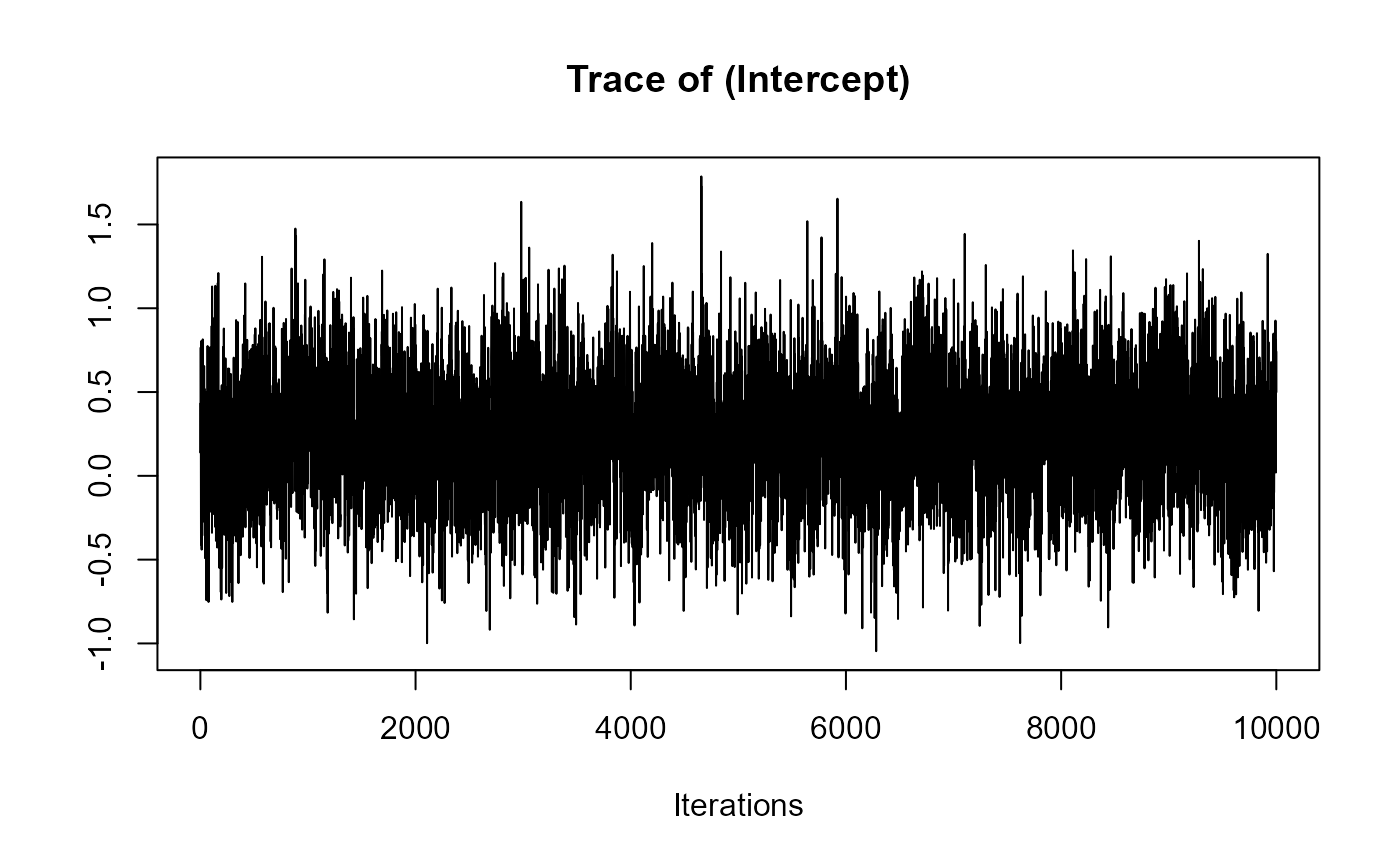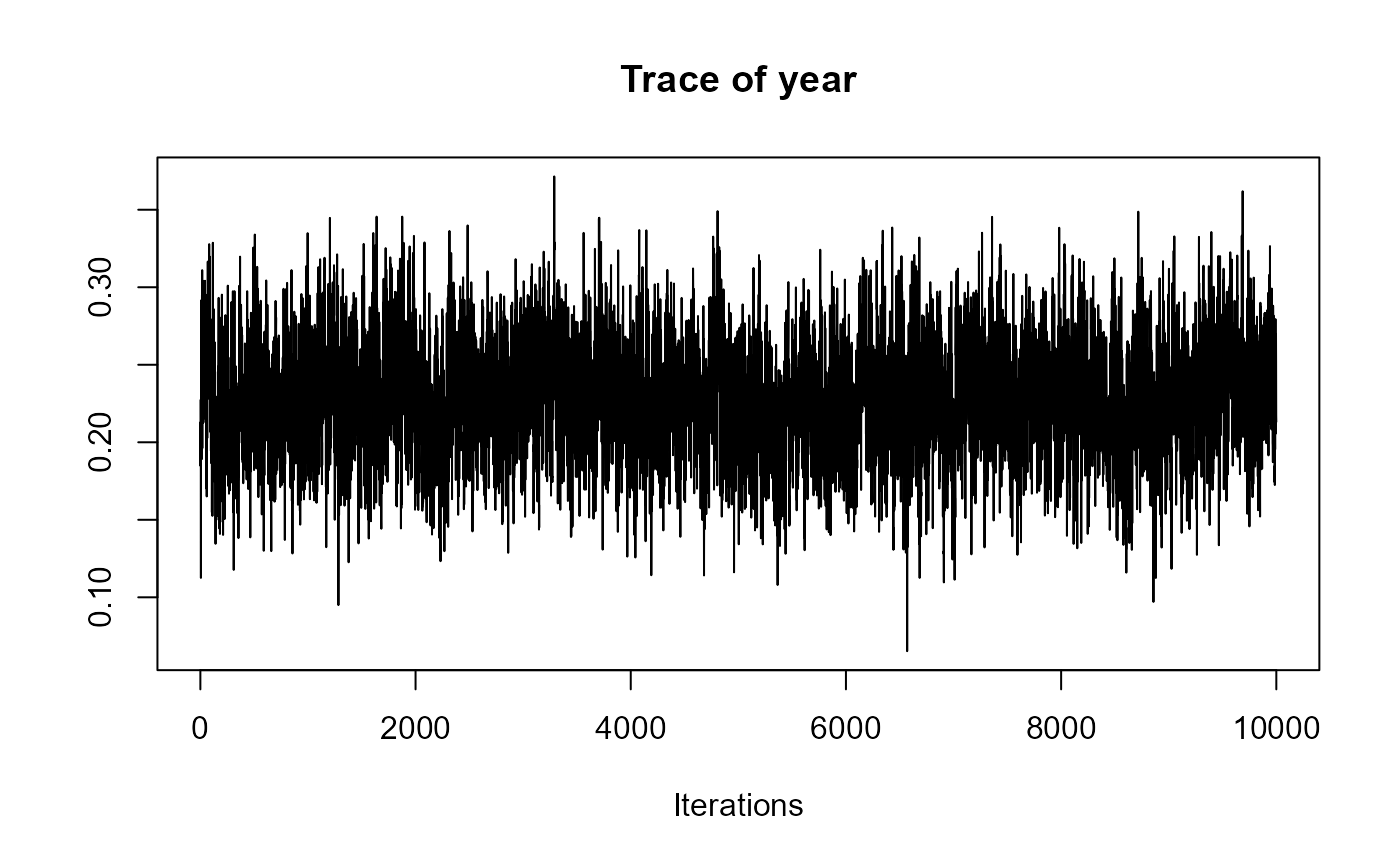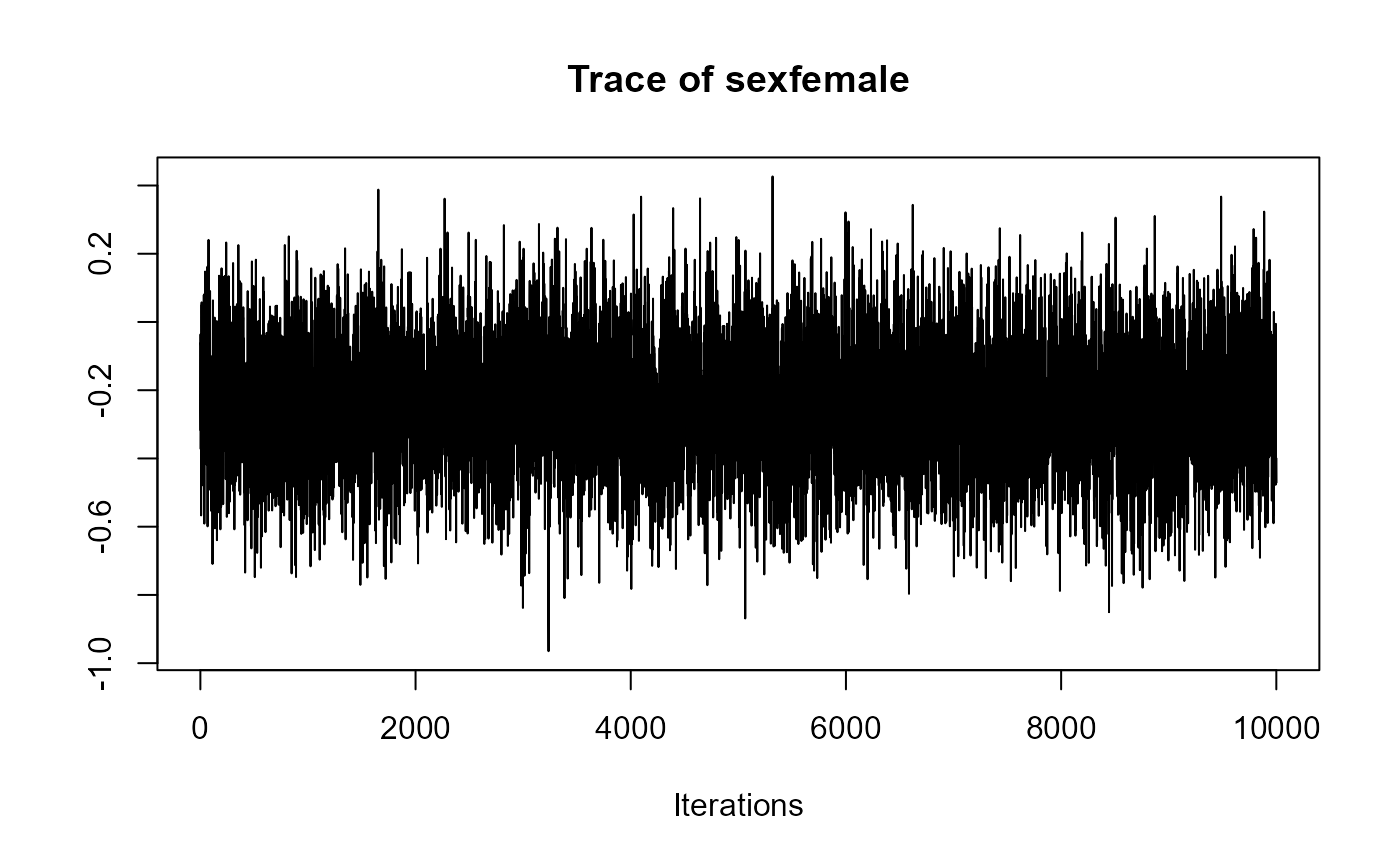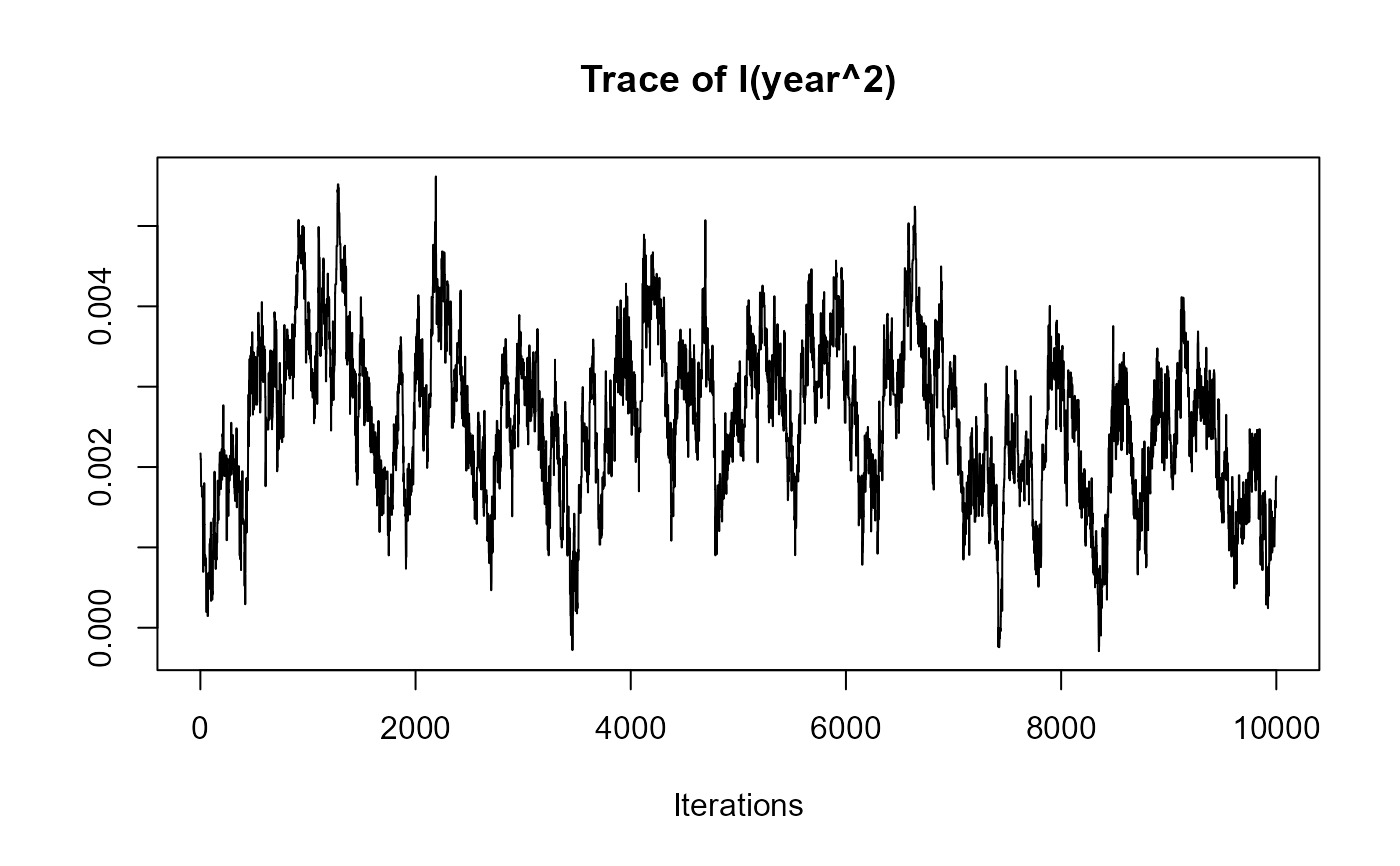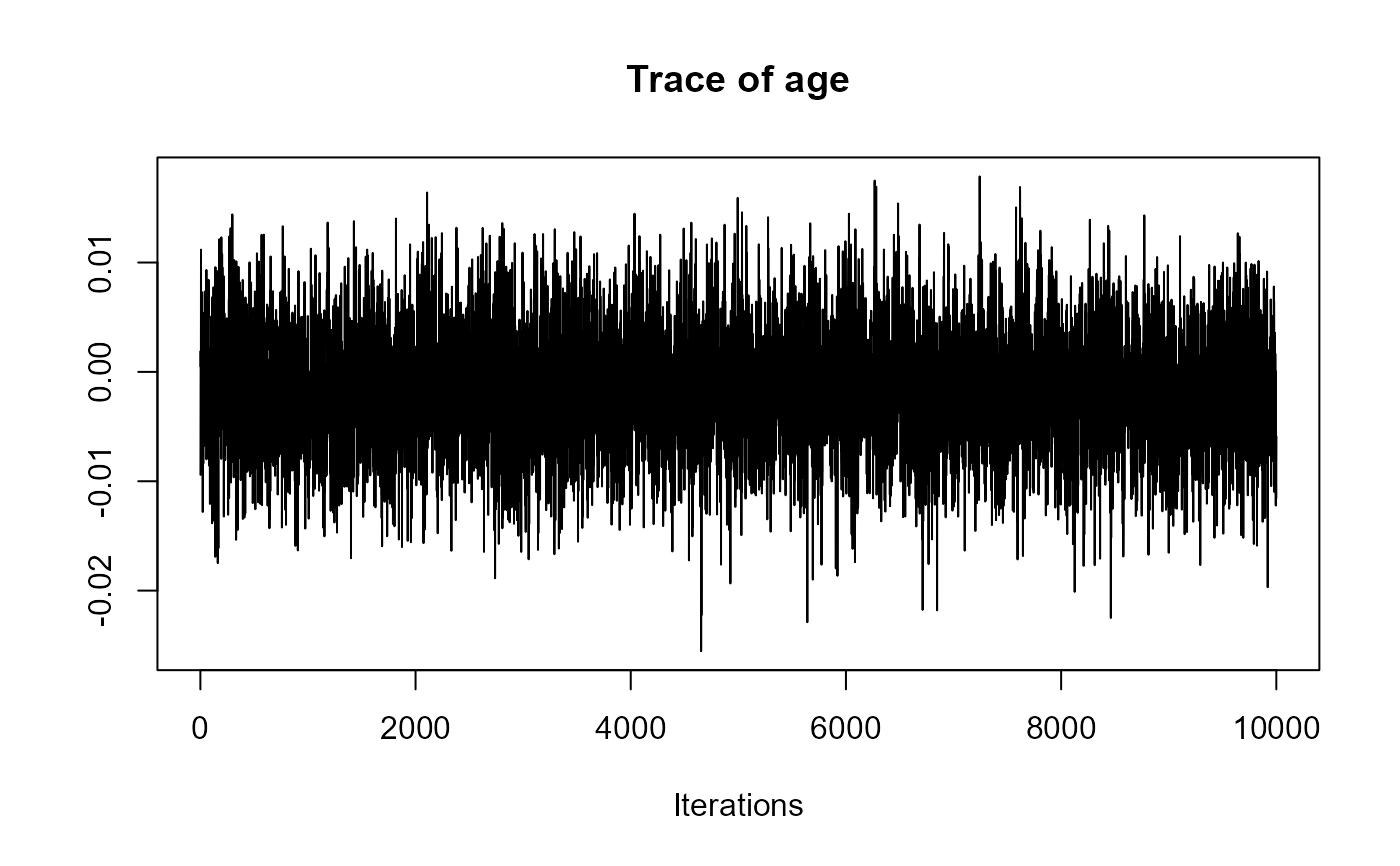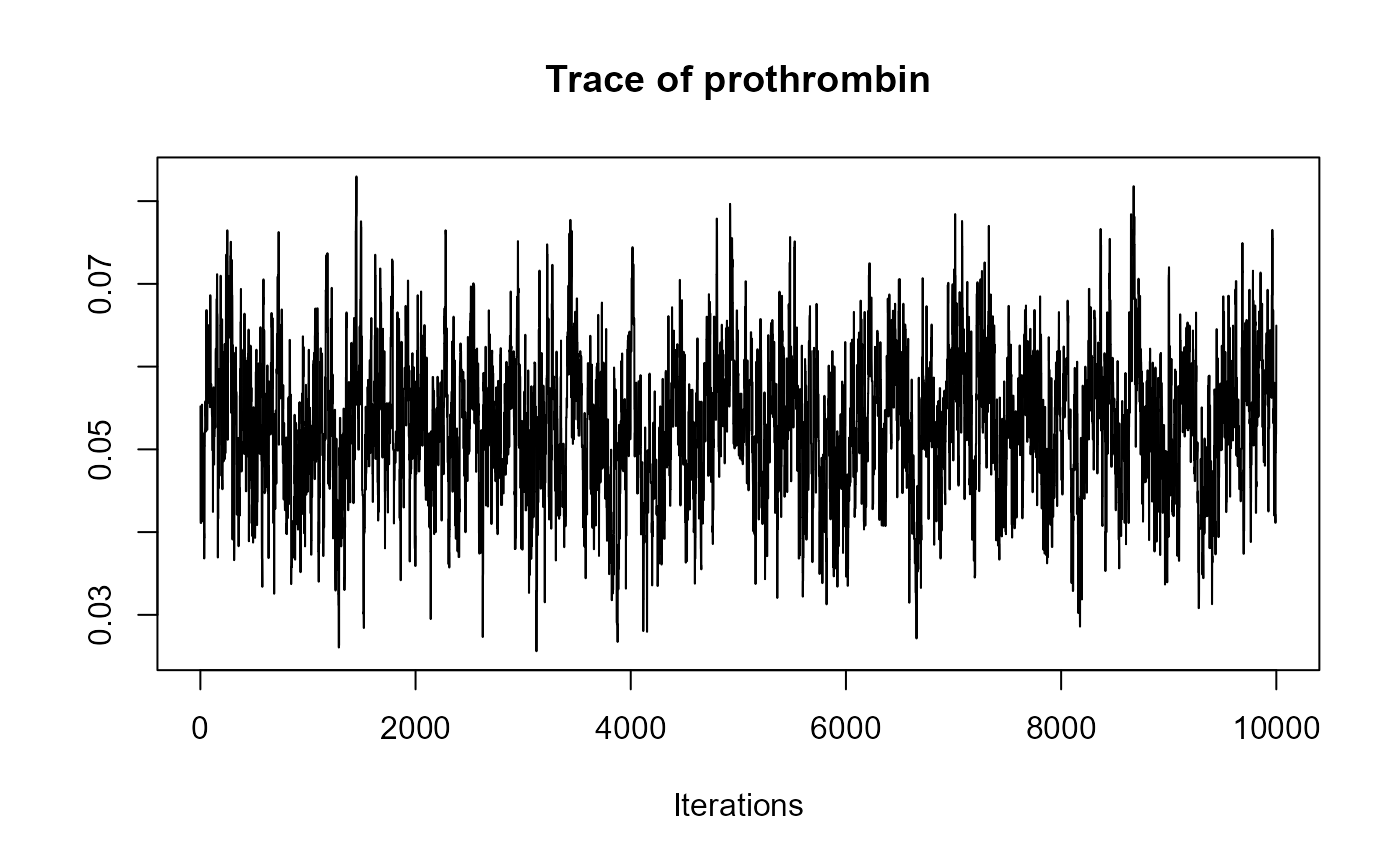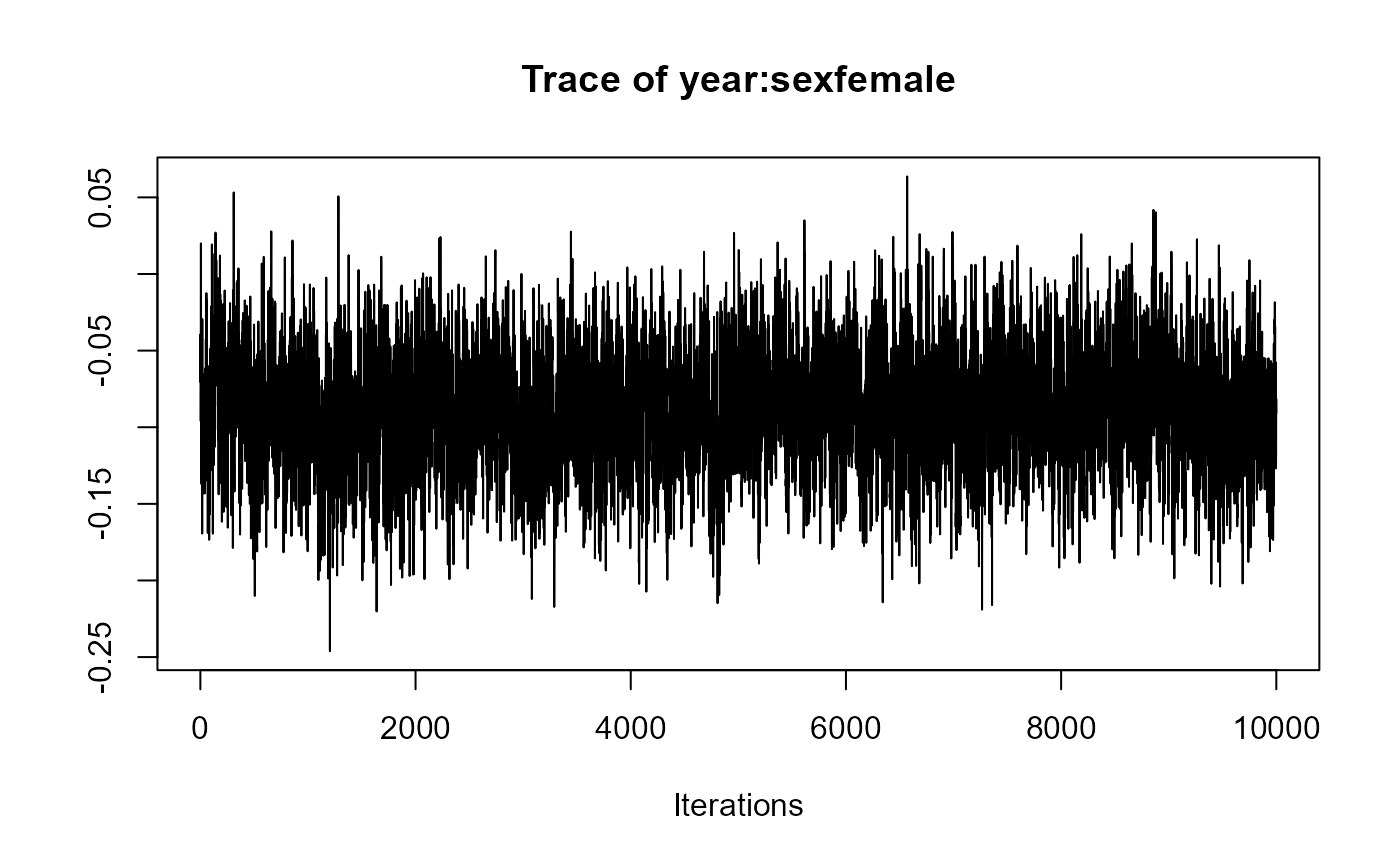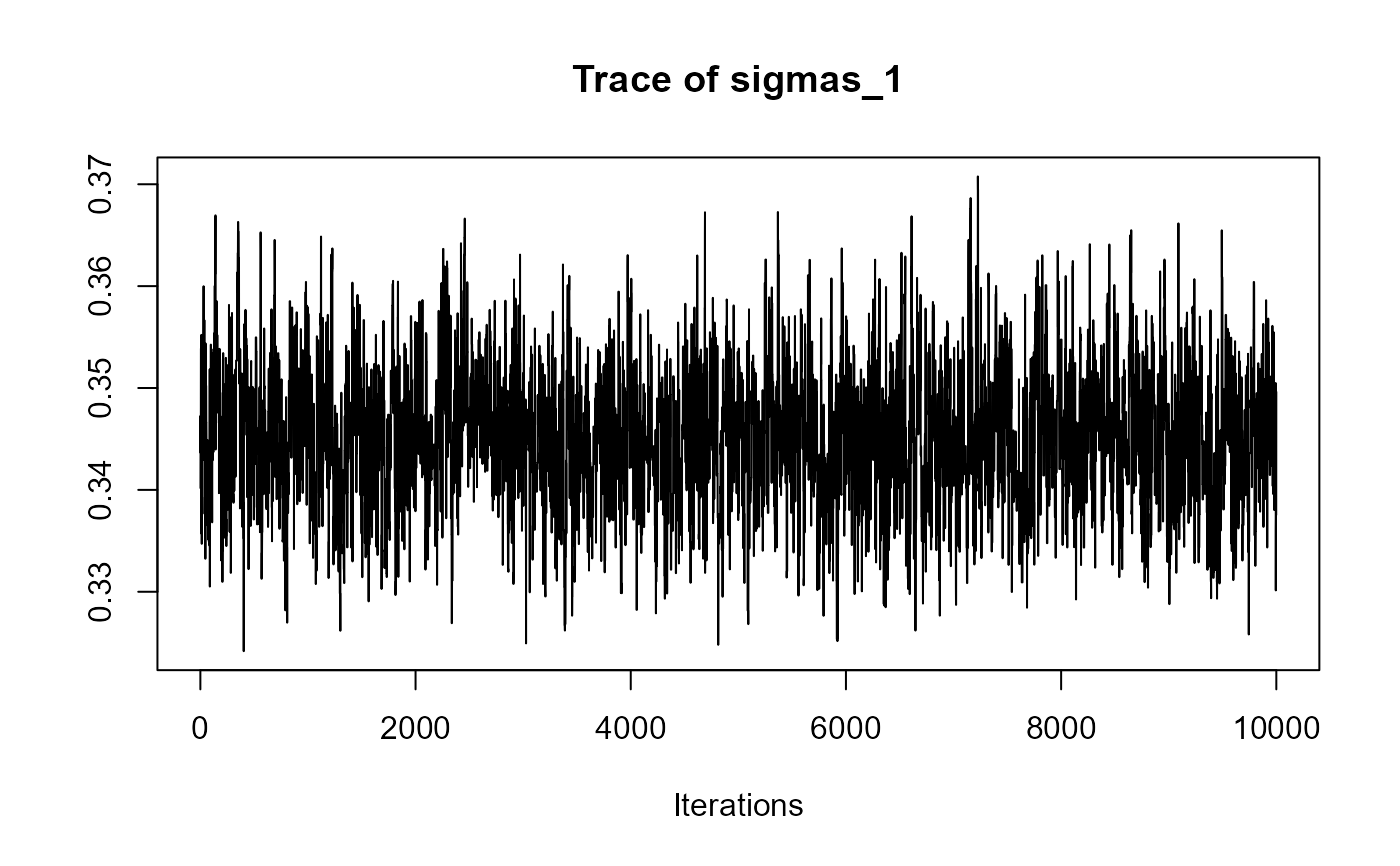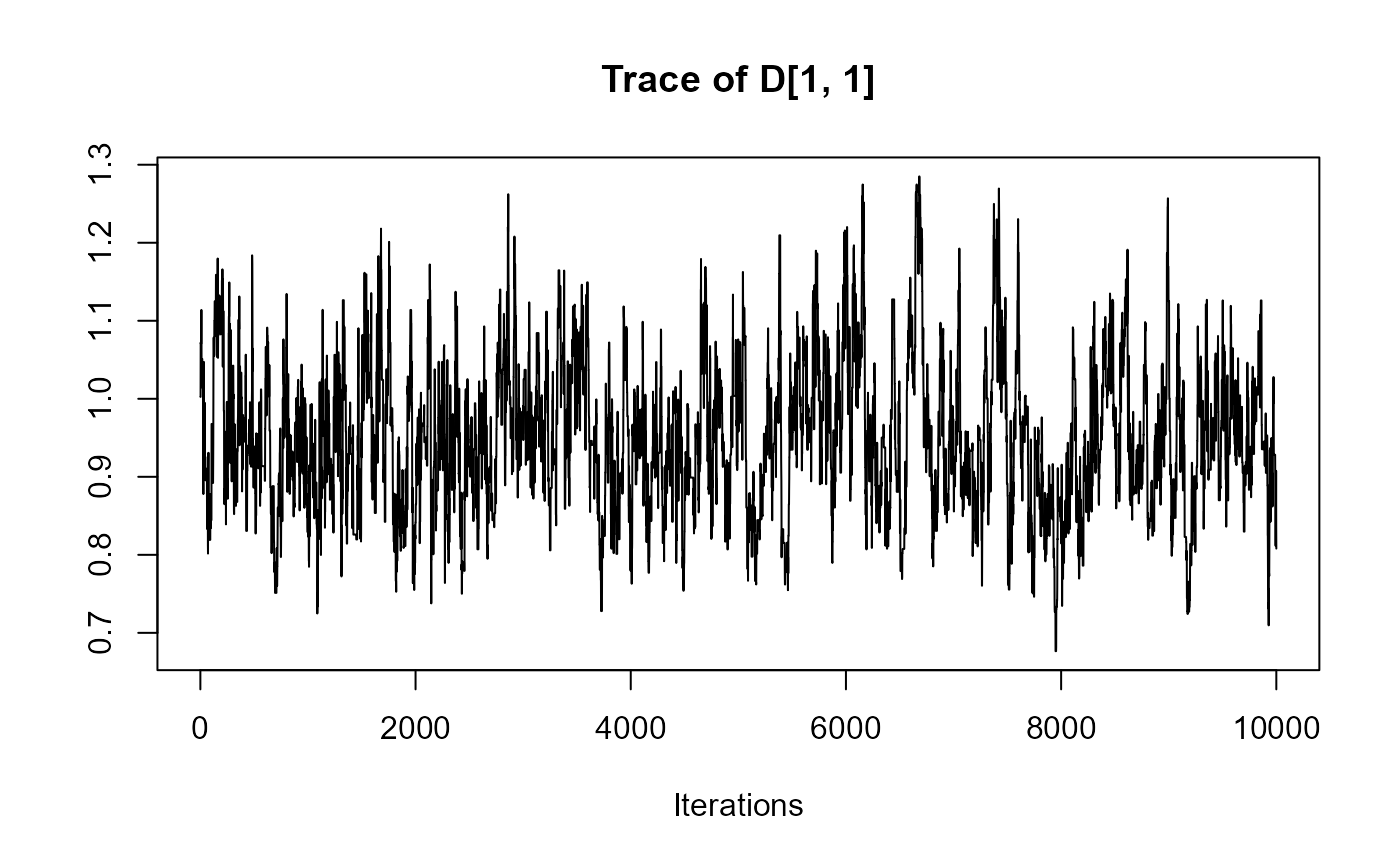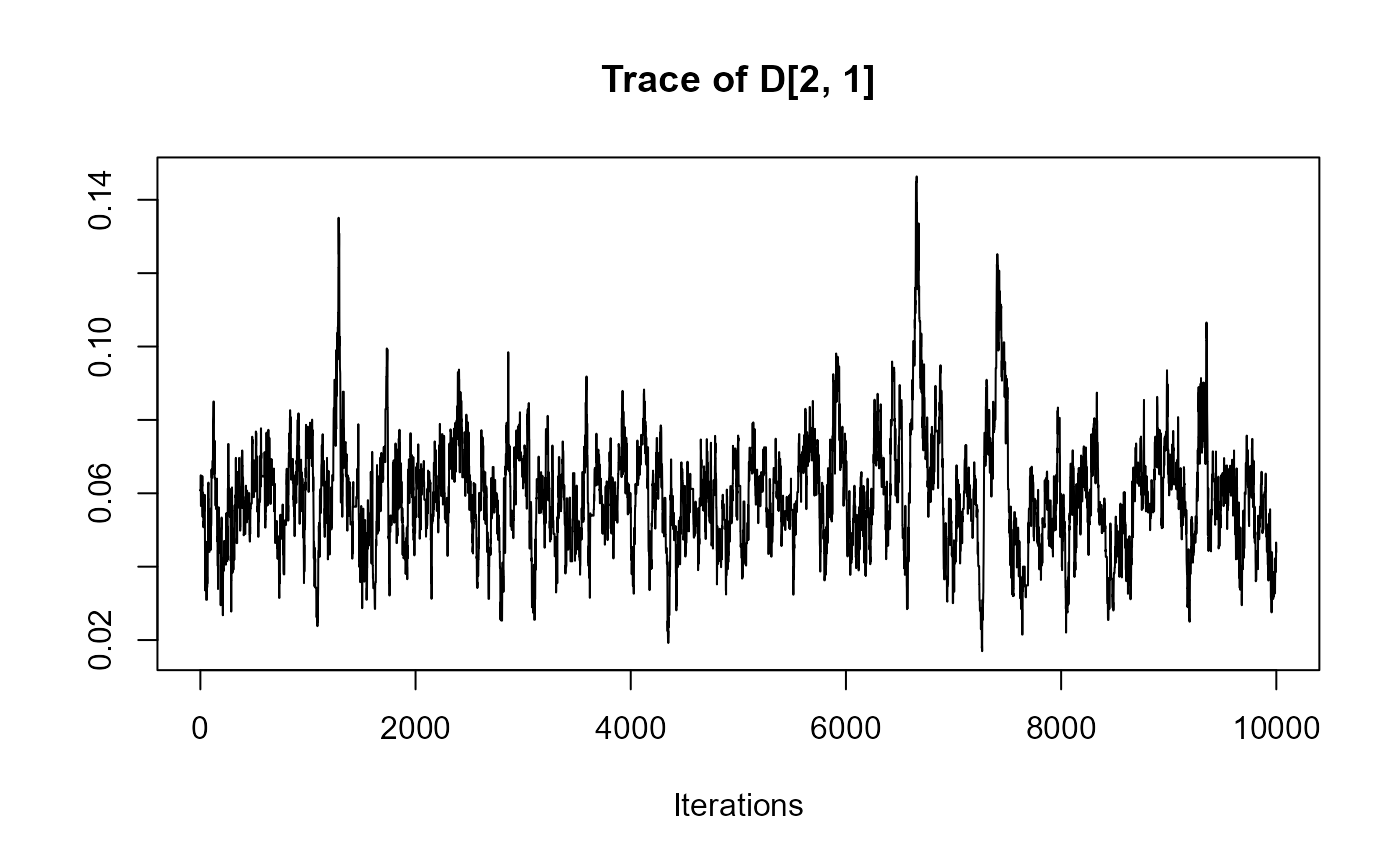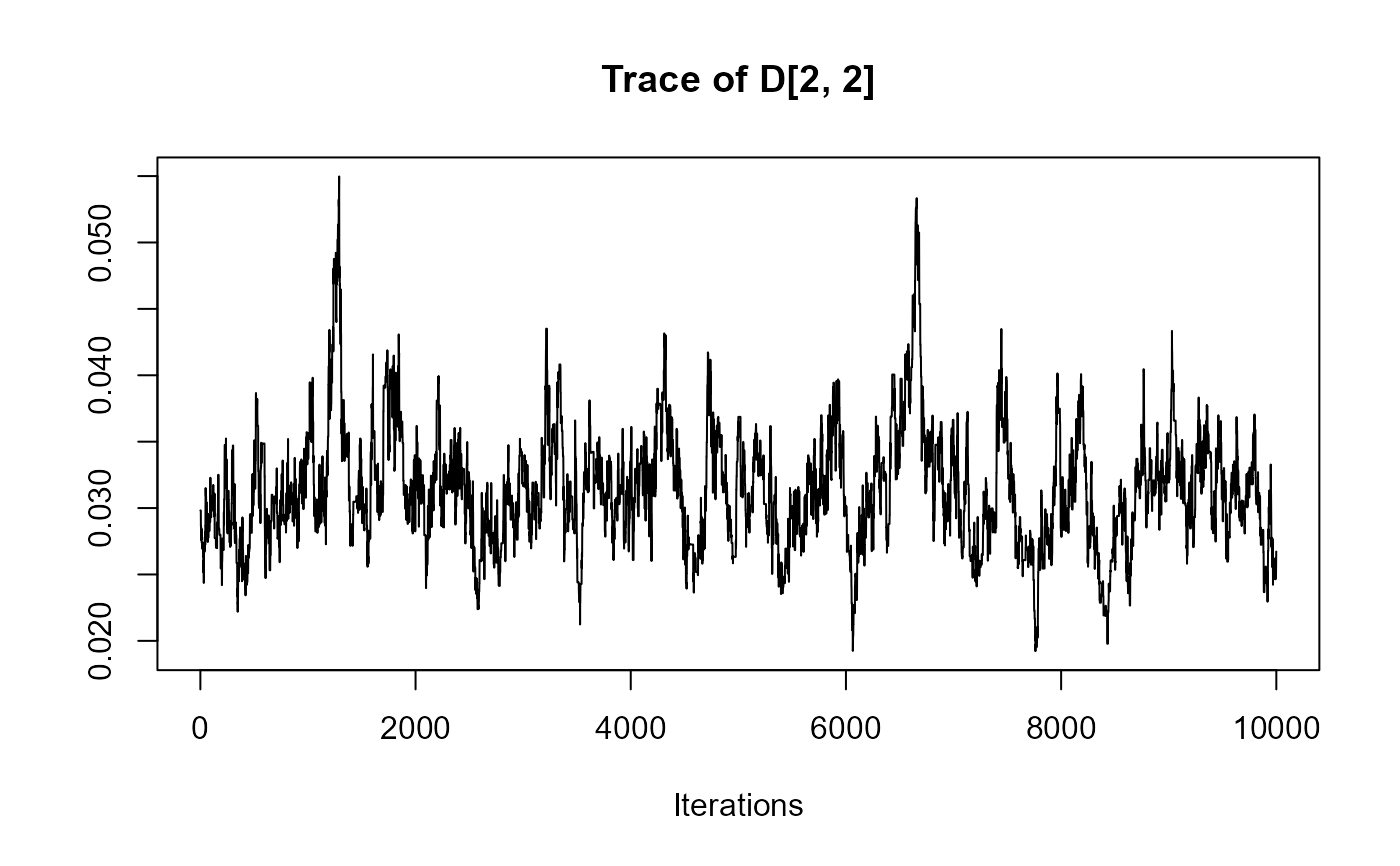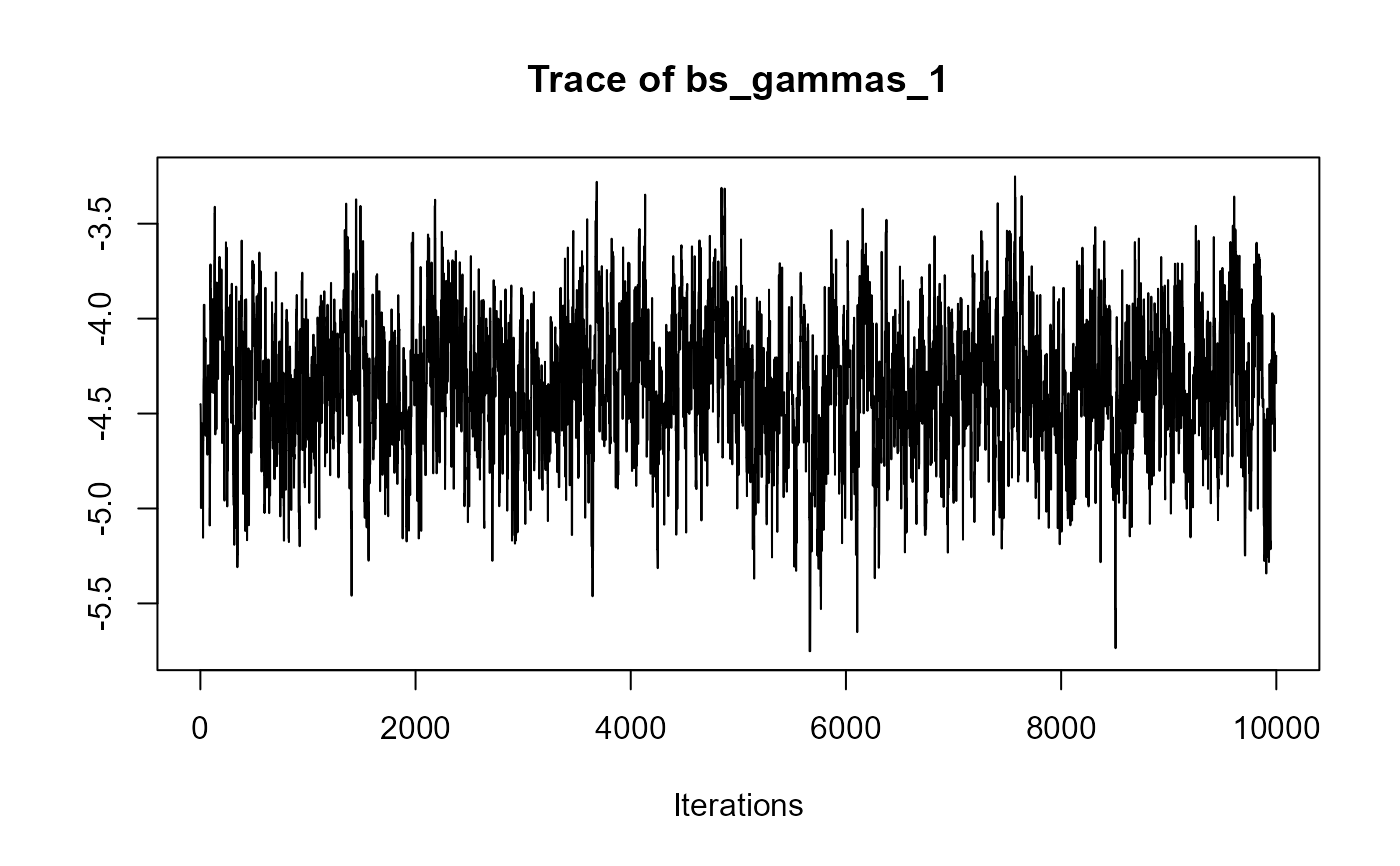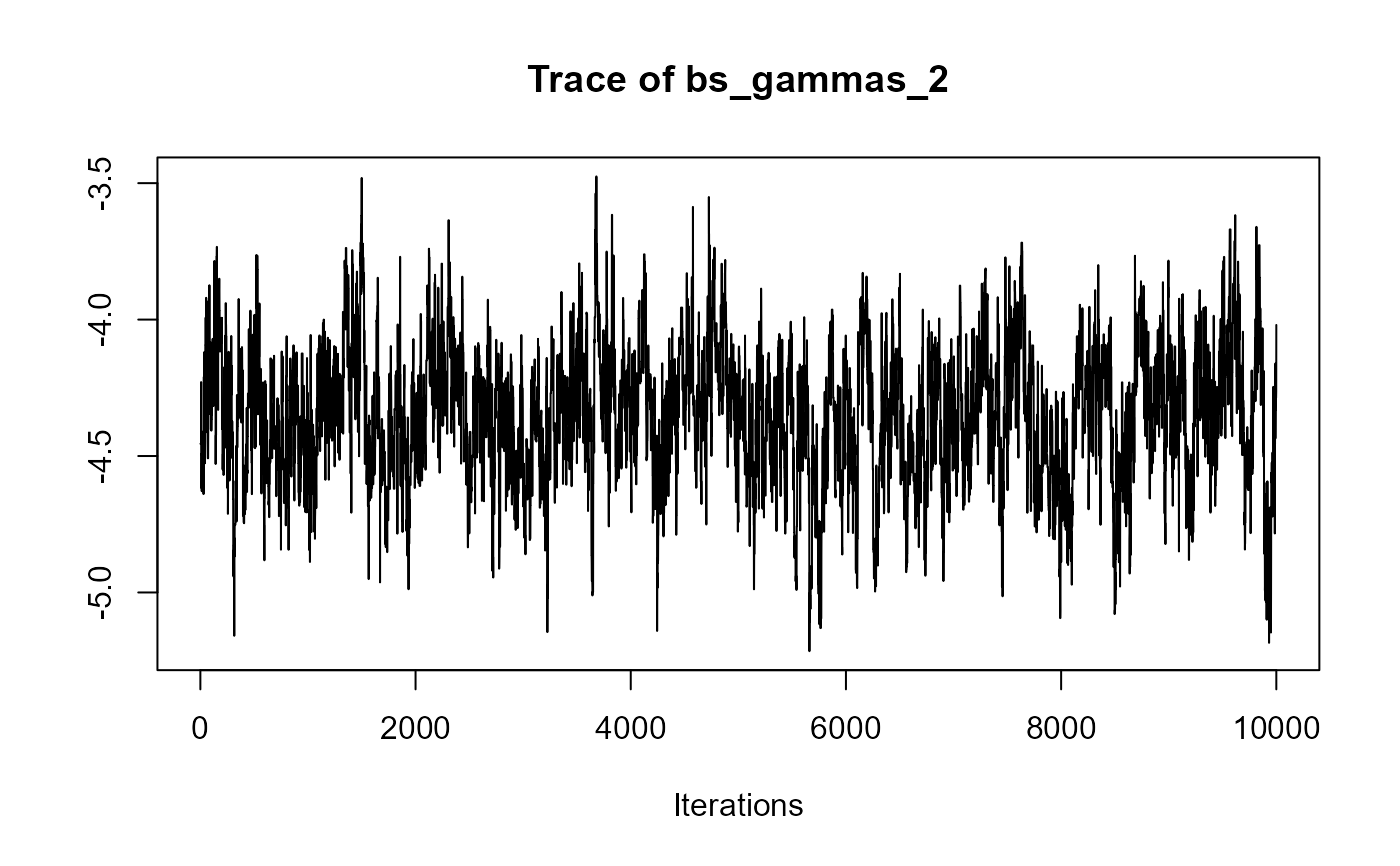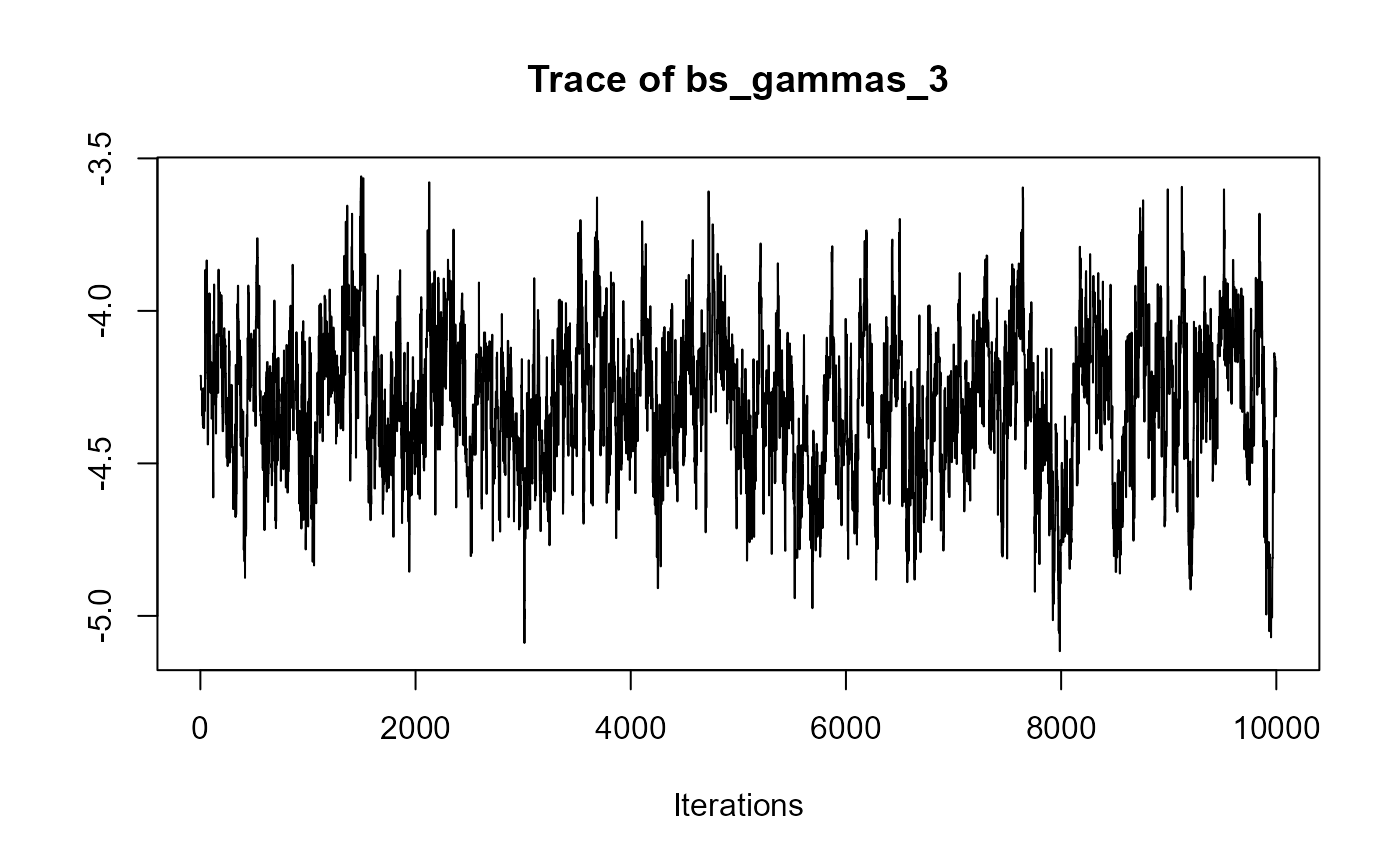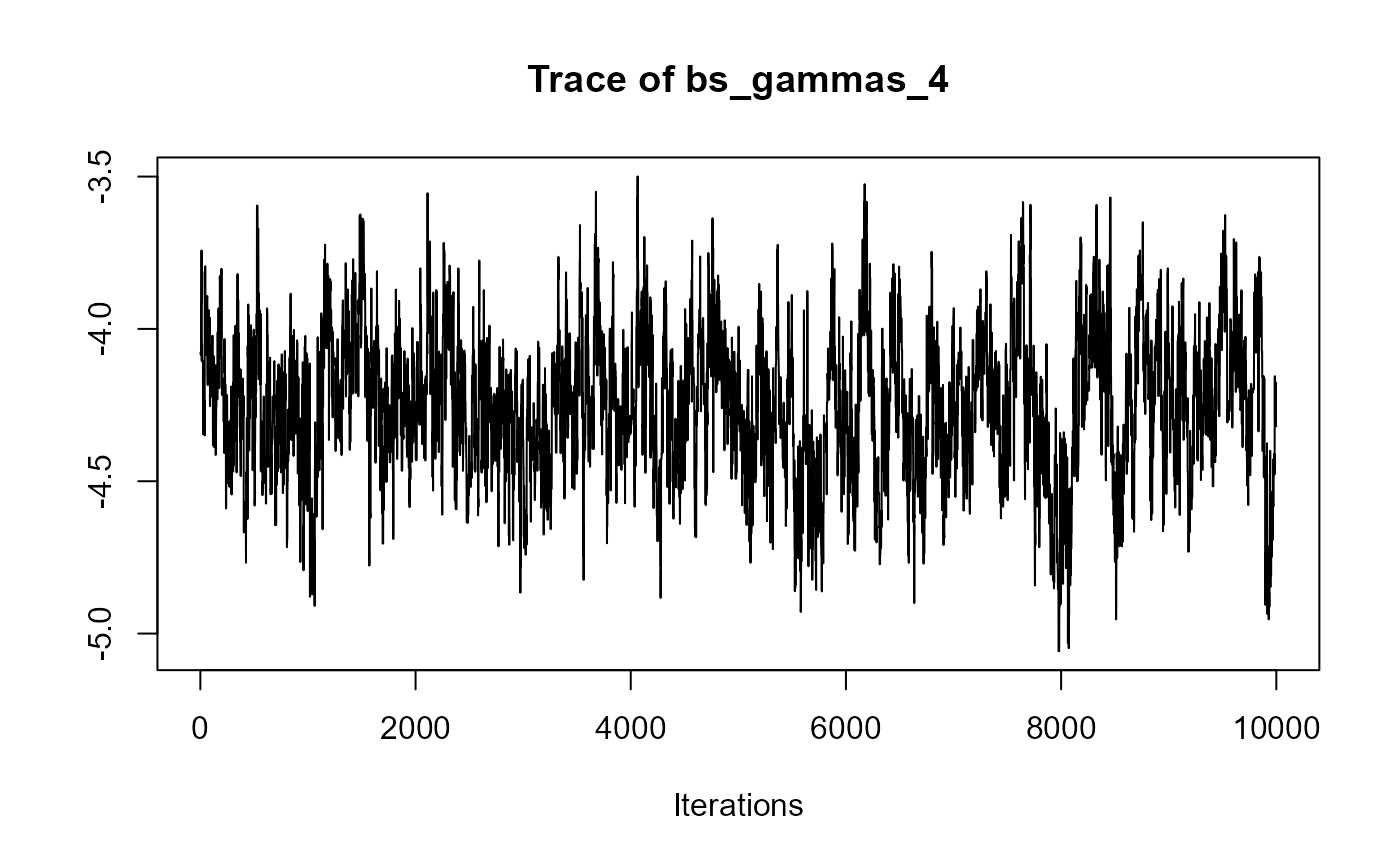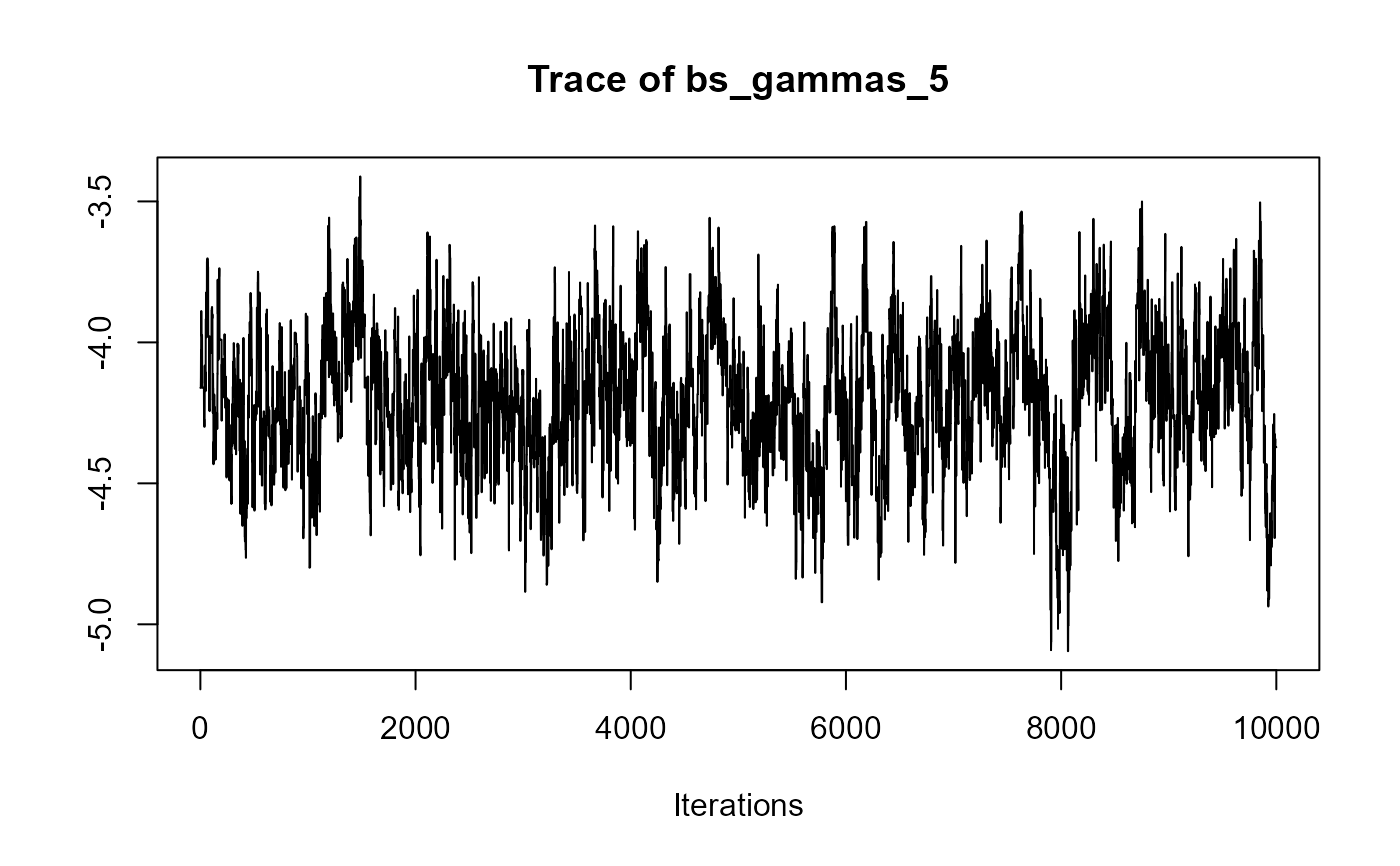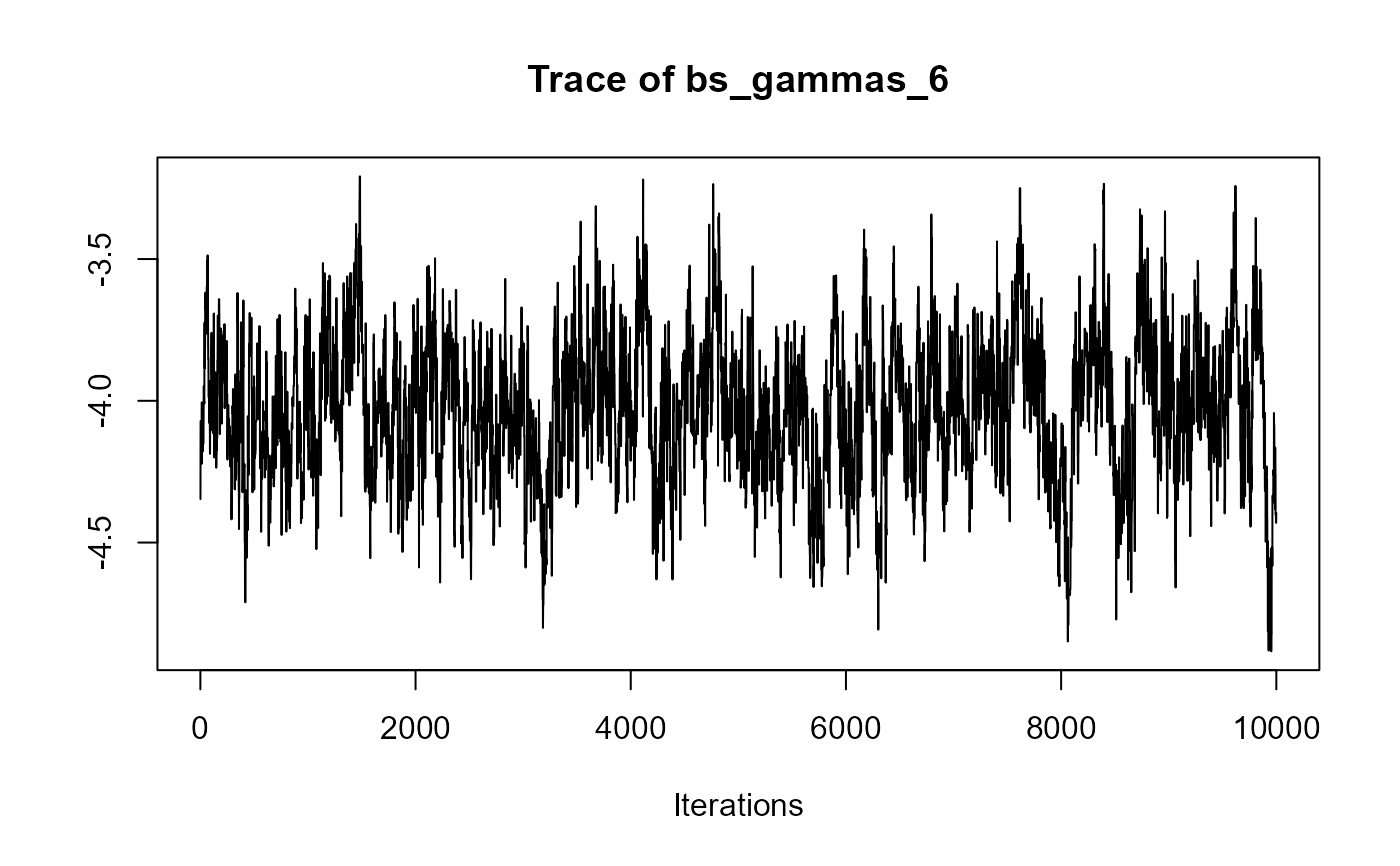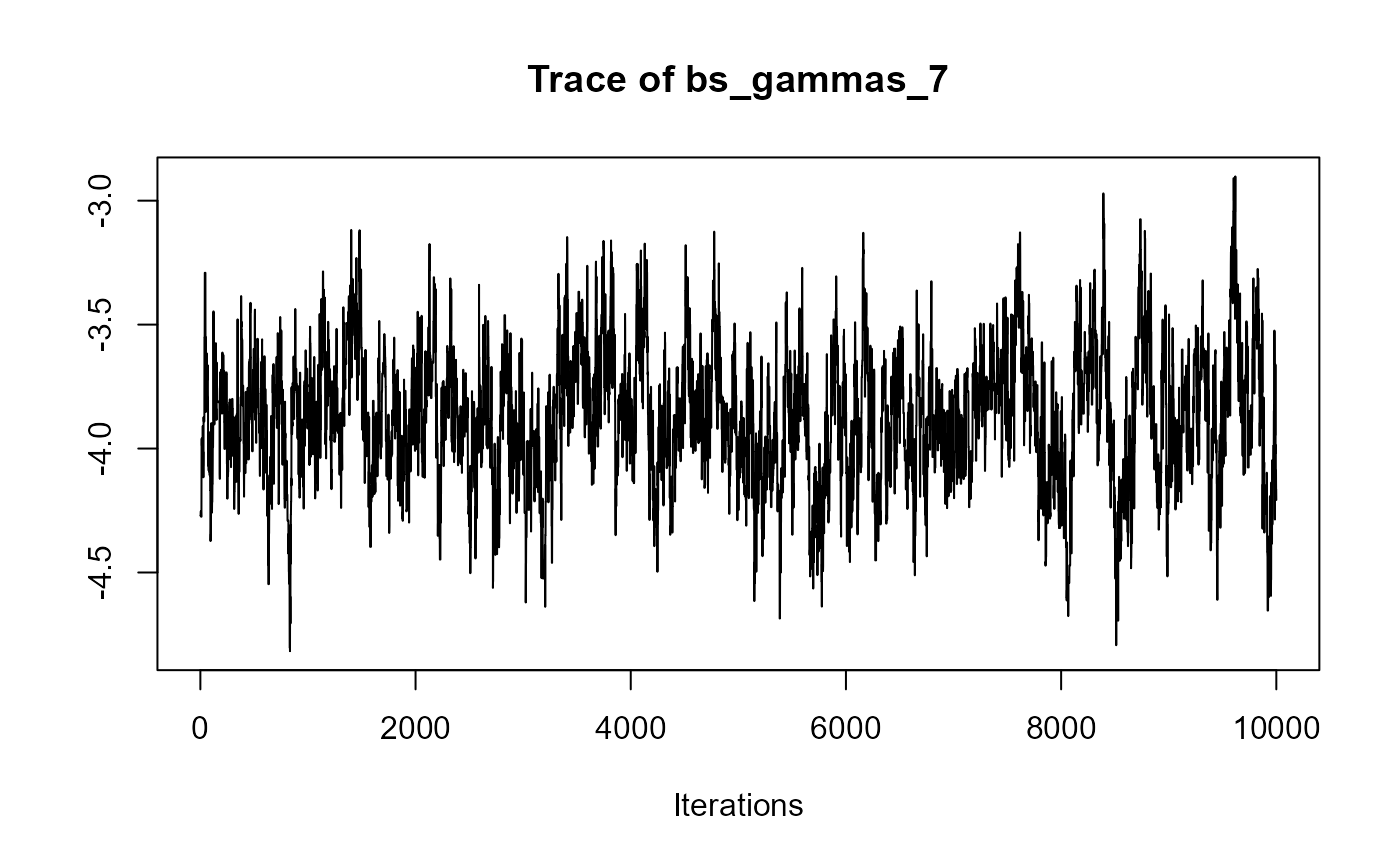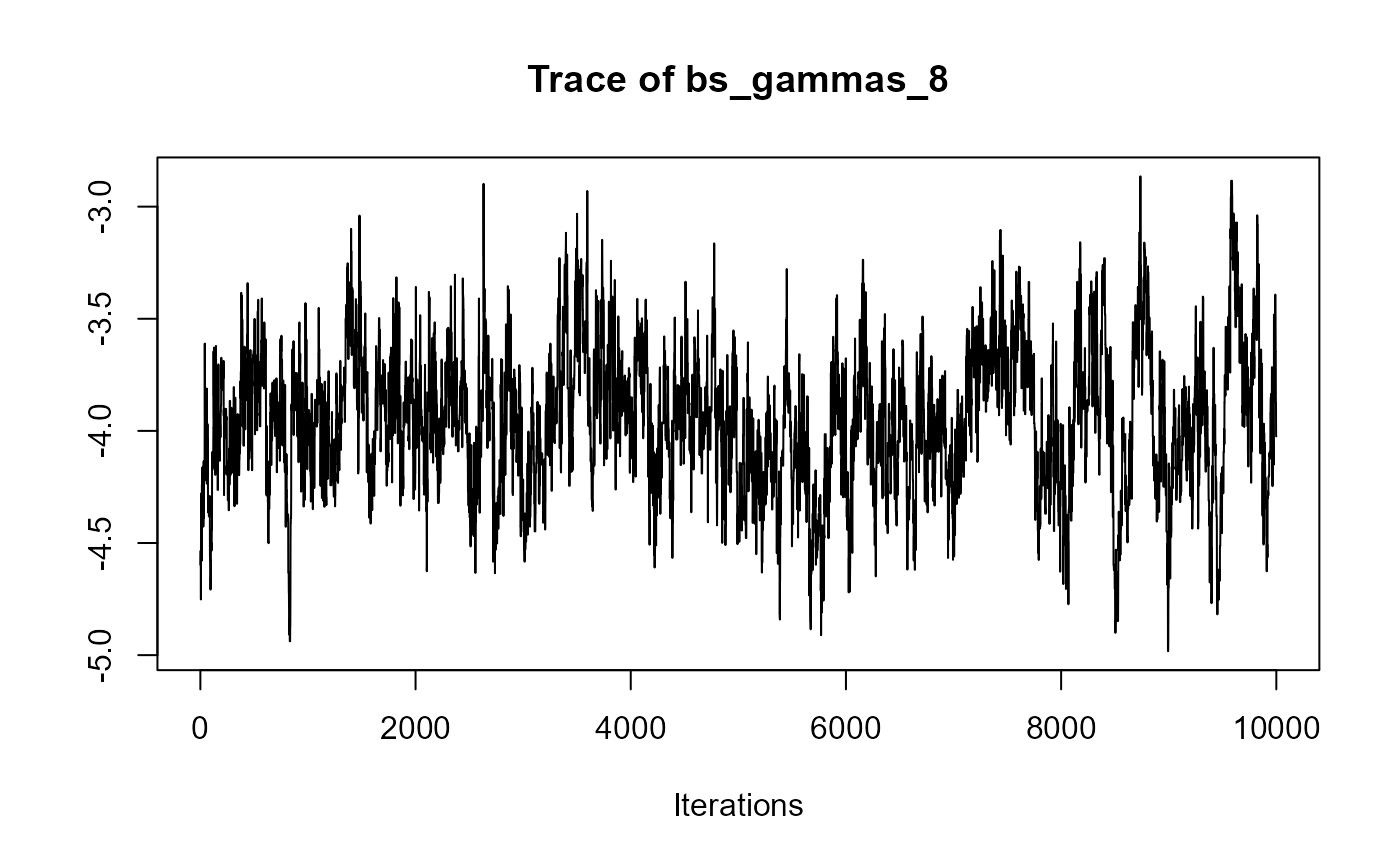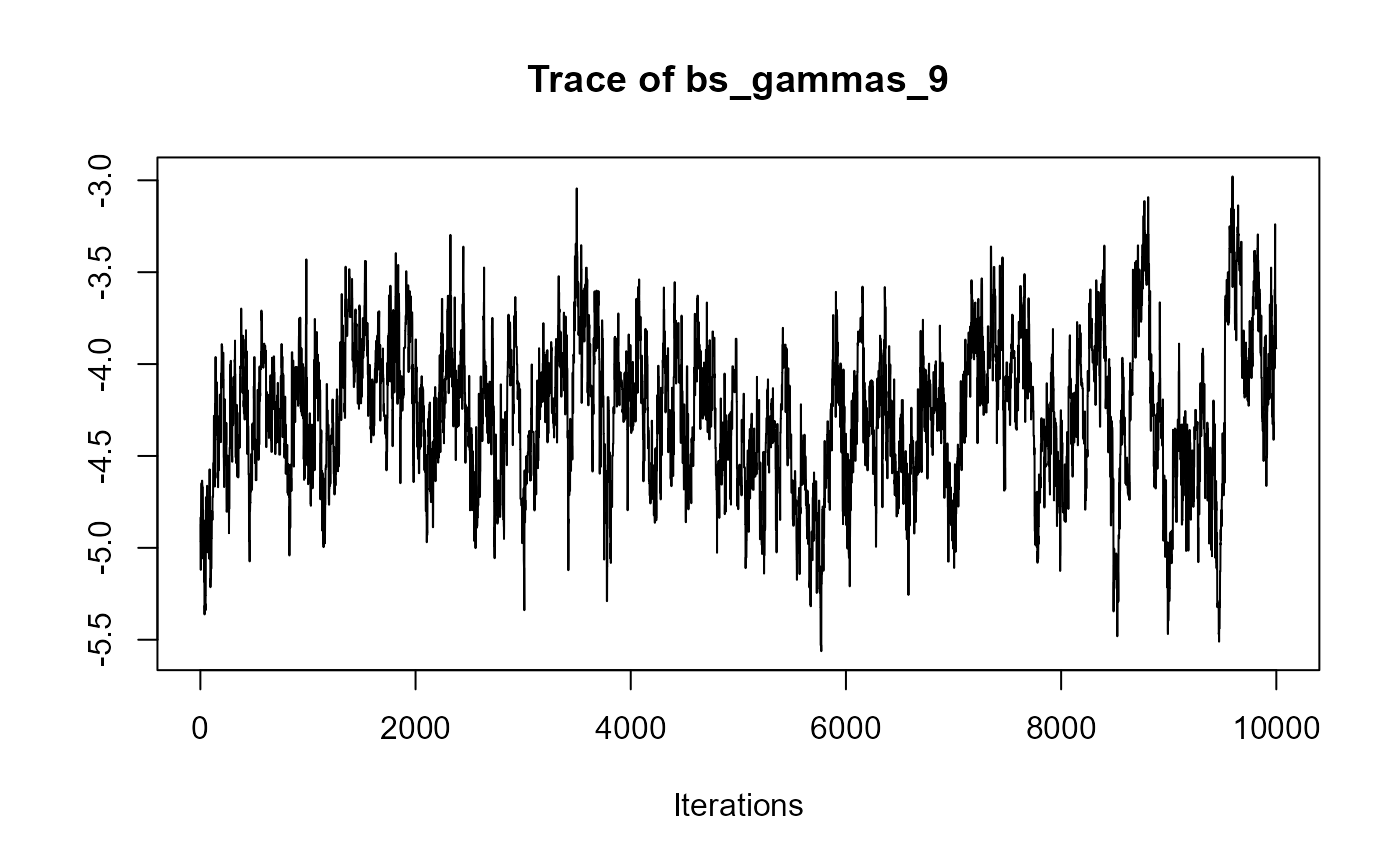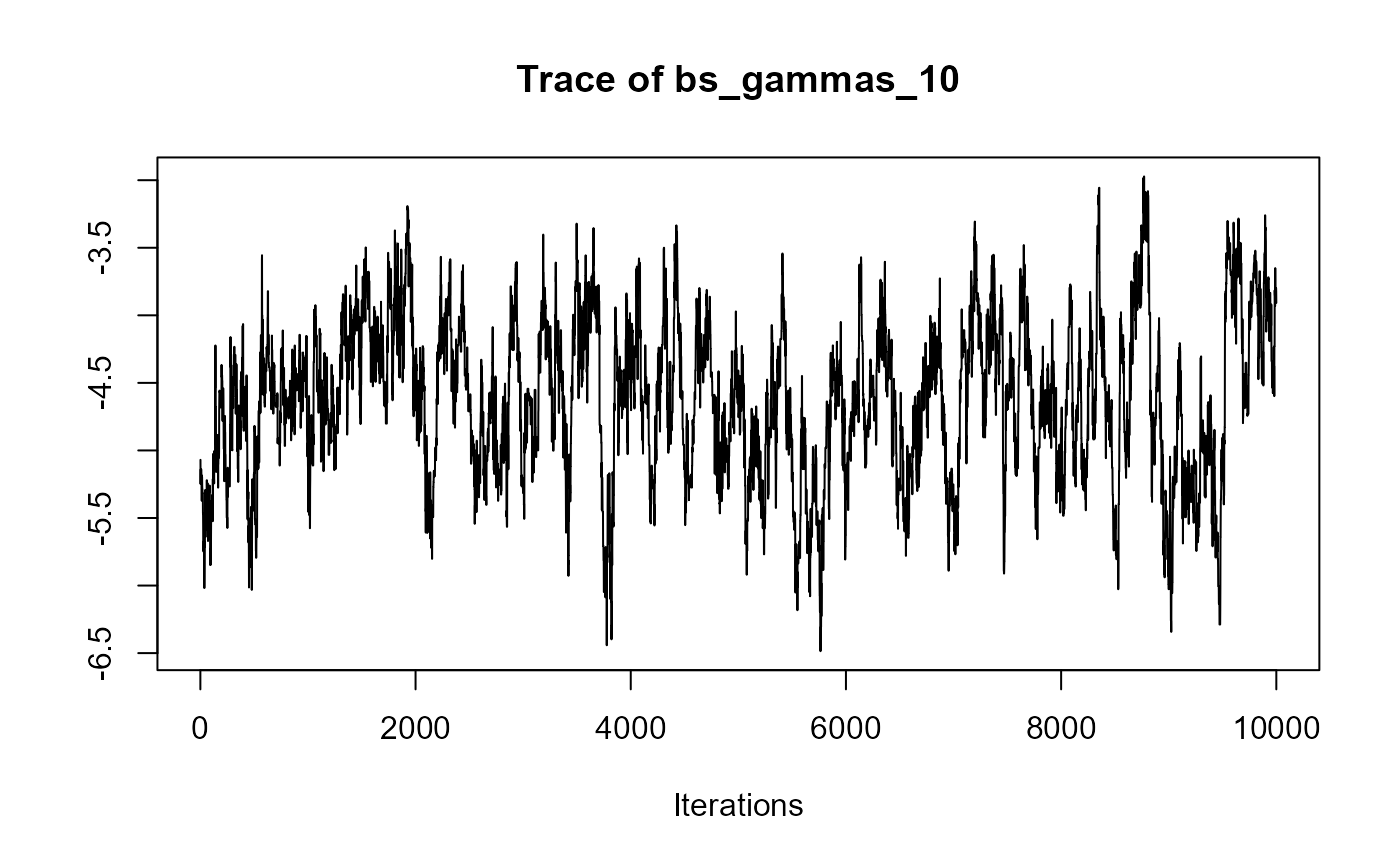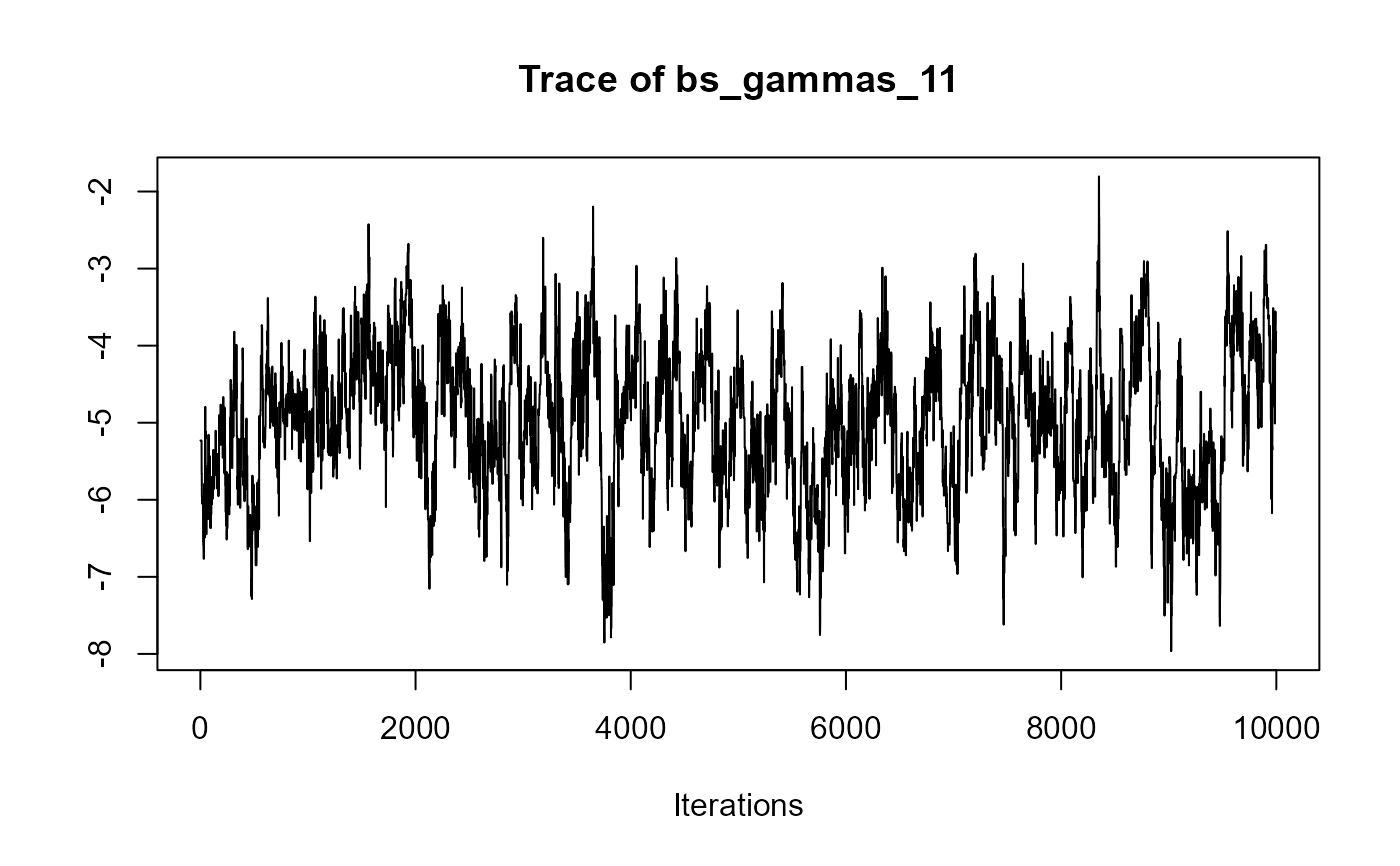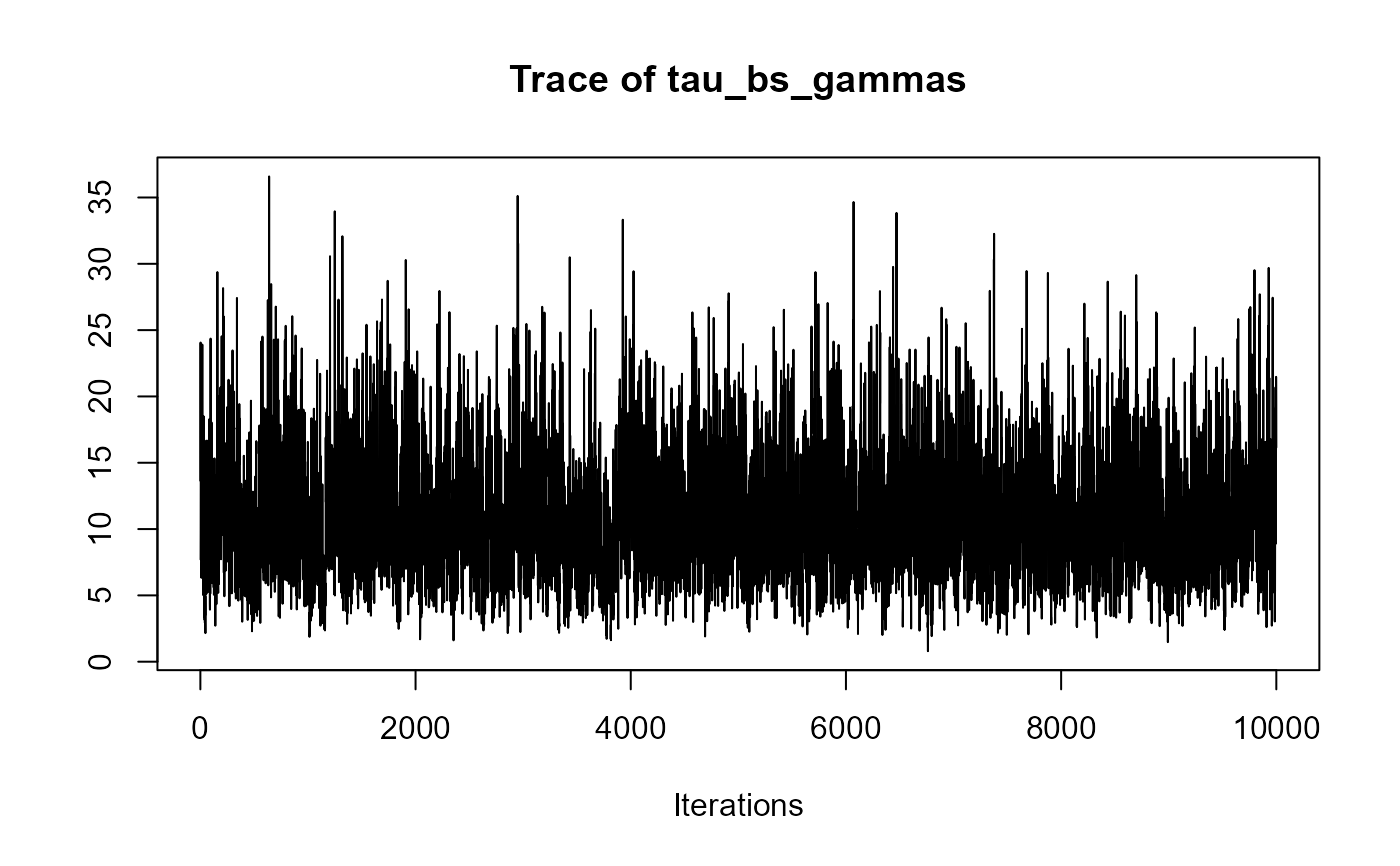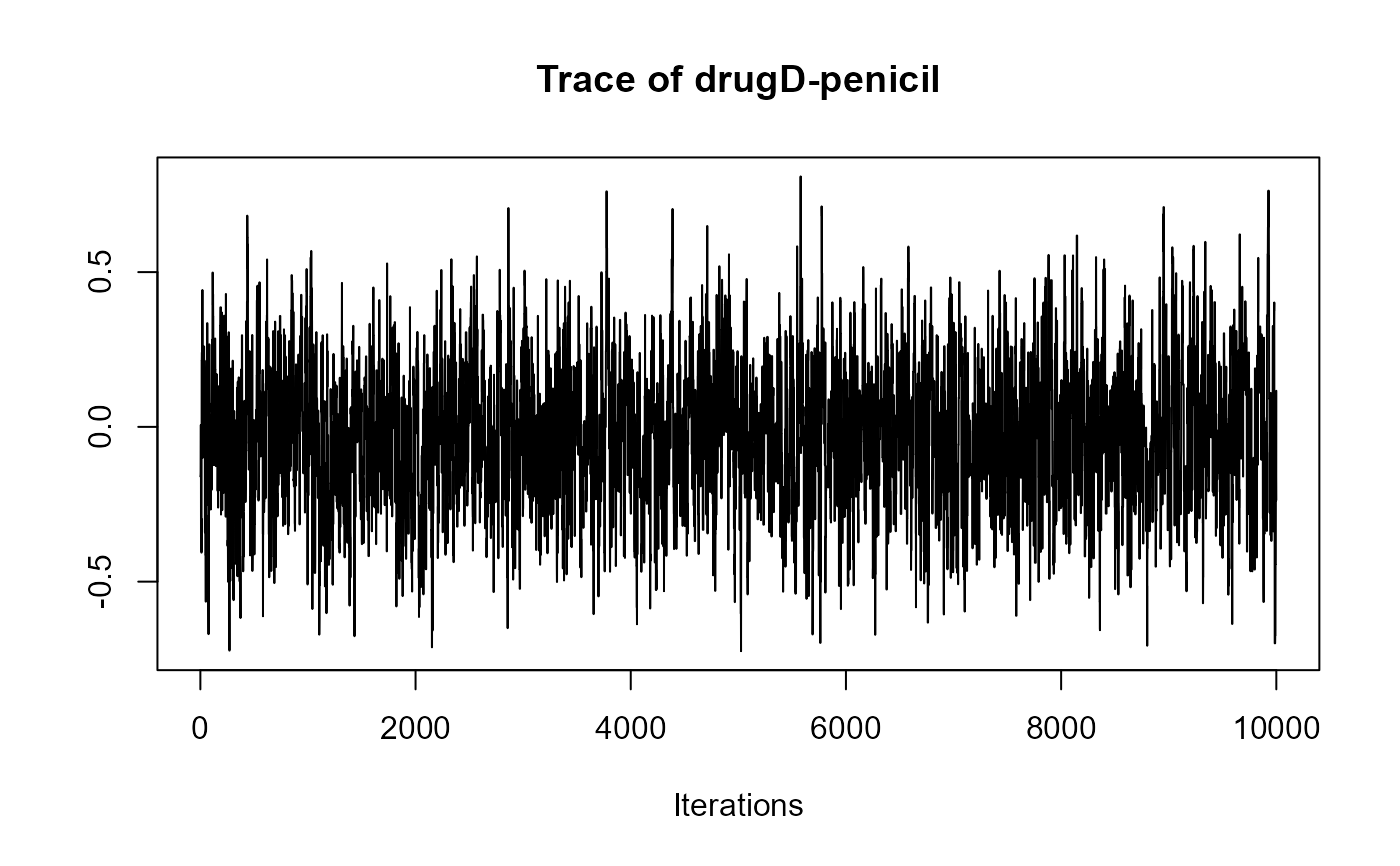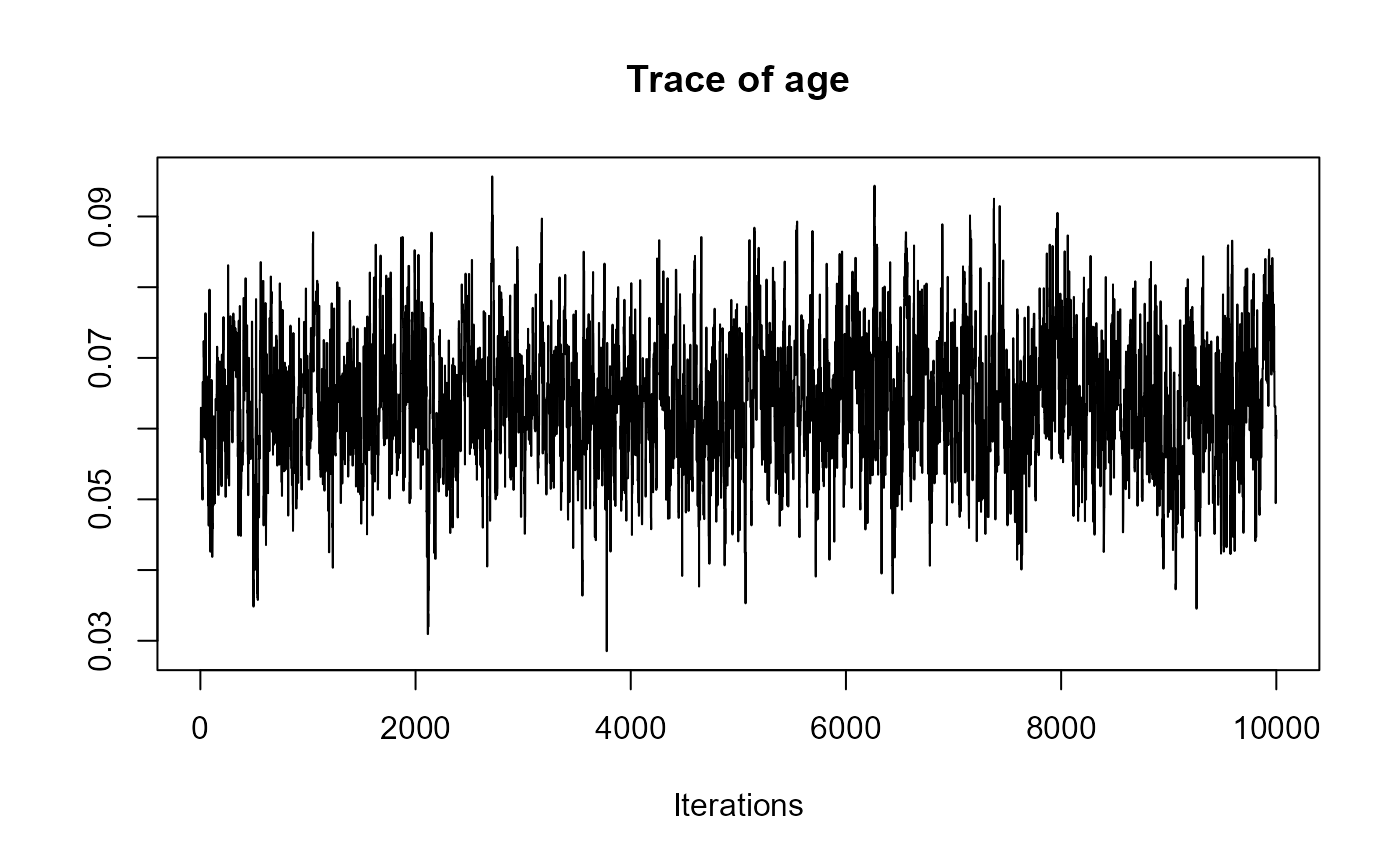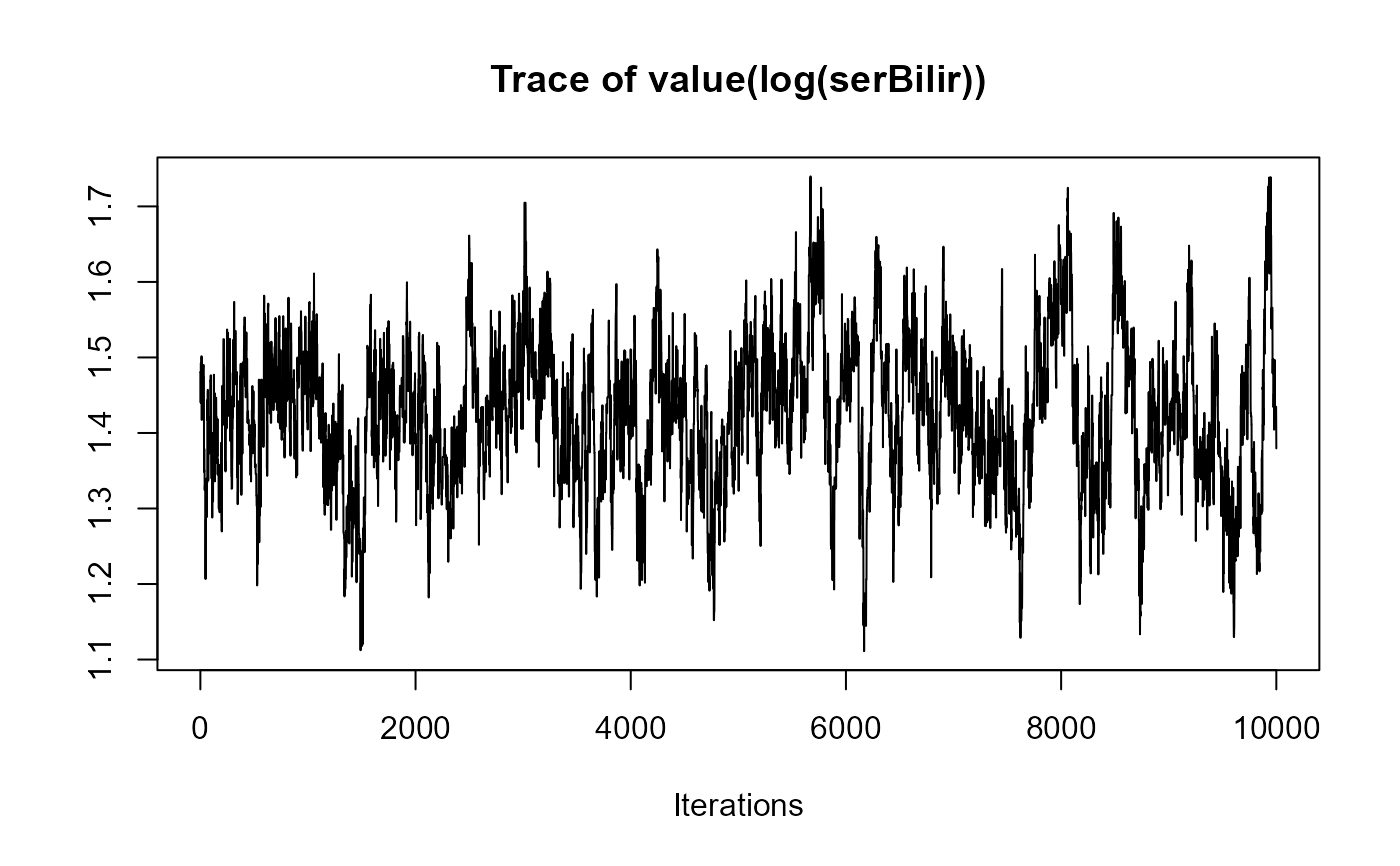################################################################################

##########################################################################
# Multivariate joint model for serum bilirubin, hepatomegaly and ascites #
# 1 continuous outcome, 2 categorical outcomes                           #
##########################################################################

#  Fit the mixed-effects models using lme() for continuous
# outcomes and mixed_model() for categorical outcomes.
fm1 <- lme(fixed = log(serBilir) ~ year * sex,
random = ~ year | id, data = pbc2)

fm2 <- mixed_model(hepatomegaly ~ sex + age + year, data = pbc2,
random = ~ year | id, family = binomial())

fm3 <- mixed_model(ascites ~ year + age, data = pbc2,
random = ~ year | id, family = binomial())

#  Save all the fitted mixed-effects models in a list.
Mixed <- list(fm1, fm2, fm3)

#  Fit a Cox model, specifying the baseline covariates to be included in the
# joint model.
fCox1 <- coxph(Surv(years, status2) ~ drug + age, data = pbc2.id)

#  The joint model is fitted using a call to jm() i.e.,
joint_model_fit_2 <- jm(fCox1, Mixed, time_var = "year",
n_chains = 1L, n_iter = 11000L, n_burnin = 1000L)
summary(joint_model_fit_2)
#>
#> Call:
#> jm(Surv_object = fCox1, Mixed_objects = Mixed, time_var = "year",
#>     n_chains = 1L, n_iter = 11000L, n_burnin = 1000L)
#>
#> Data Descriptives:
#> Number of Groups: 312		Number of events: 140 (44.9%)
#> Number of Observations:
#>   log(serBilir): 1945
#>   hepatomegaly: 1884
#>   ascites: 1885
#>
#>                  DIC     WAIC      LPML
#> marginal    6650.752 6955.396 -3874.228
#> conditional 9040.038 8779.507 -4844.055
#>
#> Random-effects covariance matrix:
#>
#>        StdDev   Corr
#> (Intr) 0.9896 (Intr)   year (Intr)    year  (Intr)
#> year   0.1781 0.3957
#> (Intr) 3.4268 0.5286 0.3397
#> year   0.6086 0.0319 0.3590 -0.3524
#> (Intr) 2.7396 0.6318 0.4985 0.5804  -0.1207
#> year   0.4277 0.3790 0.6712 0.3281  0.3948  0.0052
#>
#> Survival Outcome:
#>                         Mean  StDev    2.5%  97.5%      P
#> drugD-penicil        -0.1941 0.2743 -0.7365 0.3402 0.4818
#> age                   0.0339 0.0156  0.0013 0.0622 0.0422
#> value(log(serBilir))  0.7076 0.2285  0.2723 1.1372 0.0000
#> value(hepatomegaly)  -0.0611 0.0930 -0.2418 0.1270 0.5118
#> value(ascites)        0.5787 0.2138  0.2343 1.0622 0.0000
#>
#> Longitudinal Outcome: log(serBilir) (family = gaussian, link = identity)
#>                   Mean  StDev    2.5%   97.5%      P
#> (Intercept)     0.6634 0.1425  0.3852  0.9443 0.0000
#> year            0.2468 0.0276  0.1925  0.3005 0.0000
#> sexfemale      -0.1968 0.1472 -0.4919  0.0859 0.1788
#> year:sexfemale -0.0627 0.0289 -0.1198 -0.0063 0.0262
#> sigma           0.3482 0.0067  0.3355  0.3619 0.0000
#>
#> Longitudinal Outcome: hepatomegaly (family = binomial, link = logit)
#>                Mean  StDev    2.5%  97.5%      P
#> (Intercept)  0.1019 1.0481 -1.9302 2.1987 0.9258
#> sexfemale   -0.7782 0.5311 -1.8329 0.2479 0.1392
#> age          0.0146 0.0171 -0.0196 0.0479 0.3816
#> year         0.2549 0.0761  0.1122 0.4108 0.0006
#>
#> Longitudinal Outcome: ascites (family = binomial, link = logit)
#>                Mean  StDev     2.5%   97.5% P
#> (Intercept) -8.7919 1.0133 -10.7422 -6.8467 0
#> year         0.5683 0.0658   0.4425  0.6912 0
#> age          0.0788 0.0164   0.0474  0.1108 0
#>
#> MCMC summary:
#> chains: 1
#> iterations per chain: 11000
#> burn-in per chain: 1000
#> thinning: 1
#> time: 1.4 min
traceplot(joint_model_fit_2)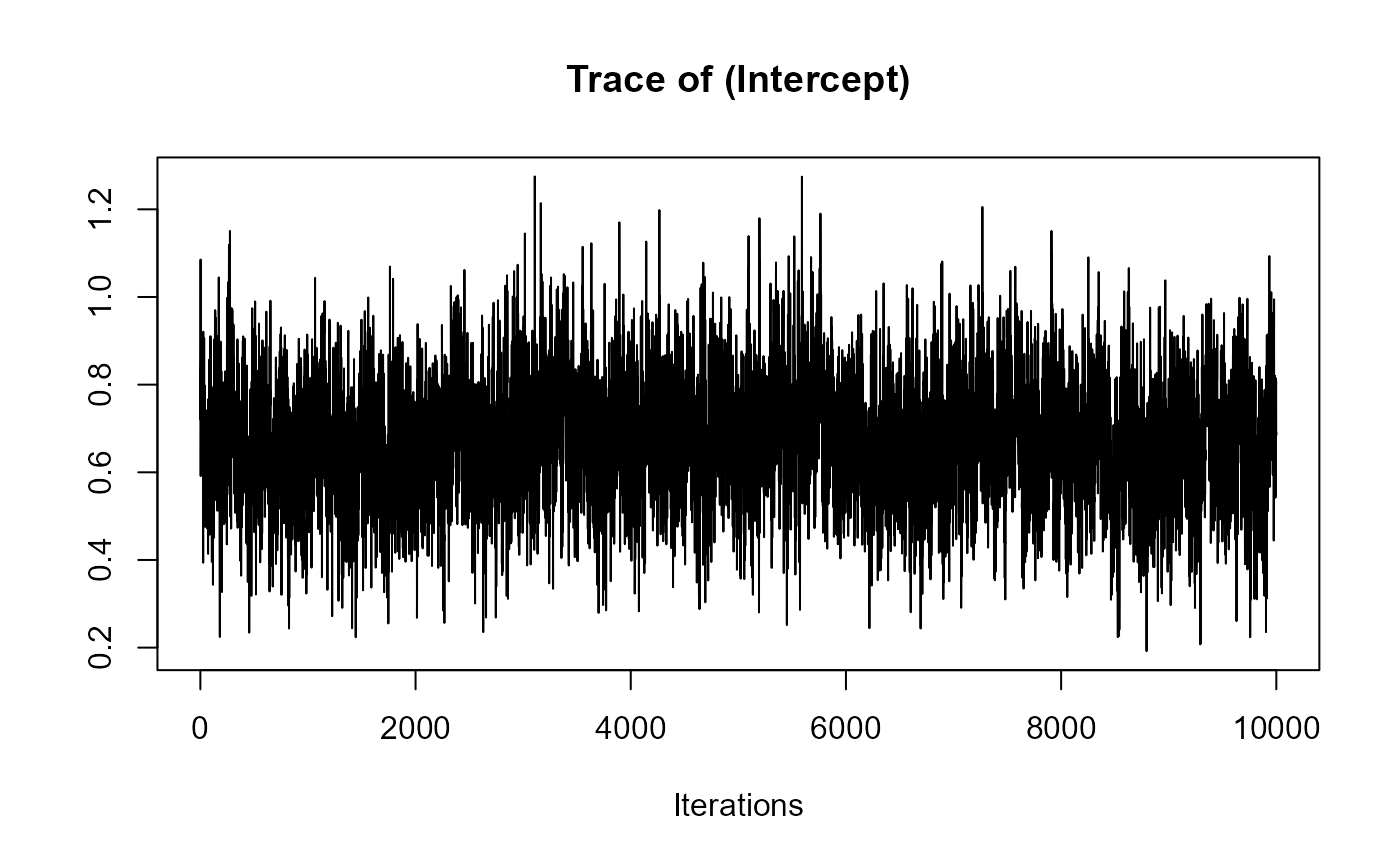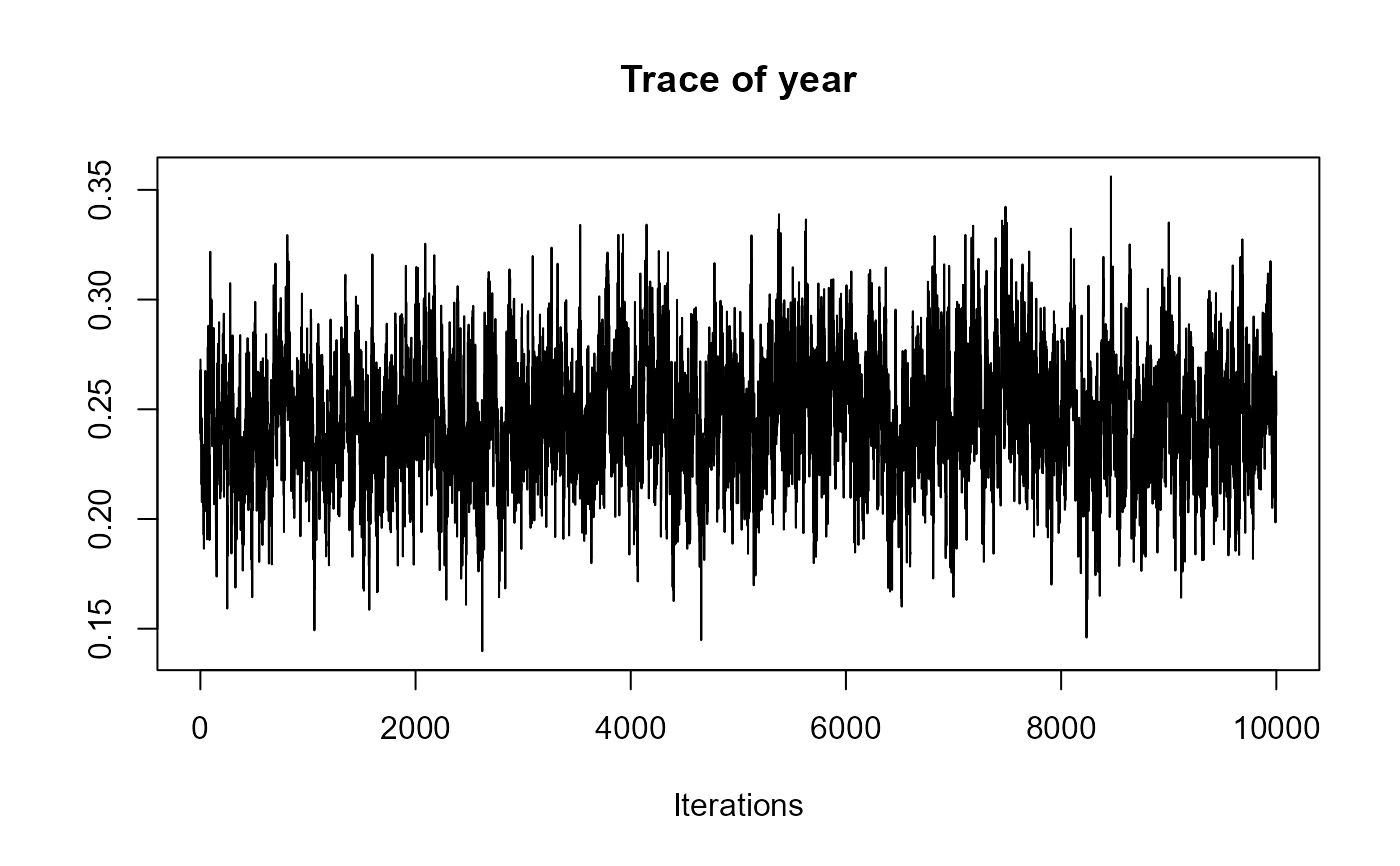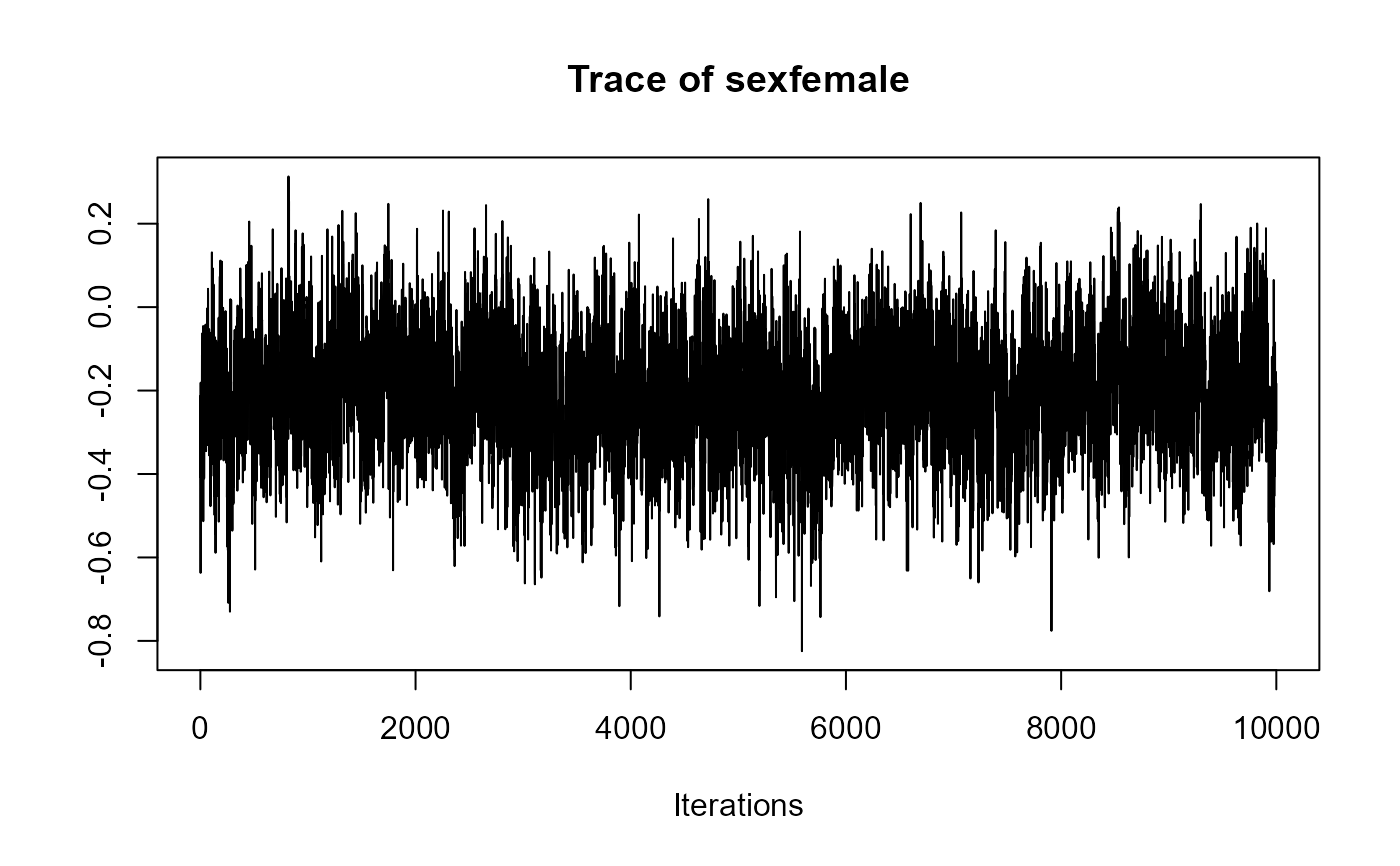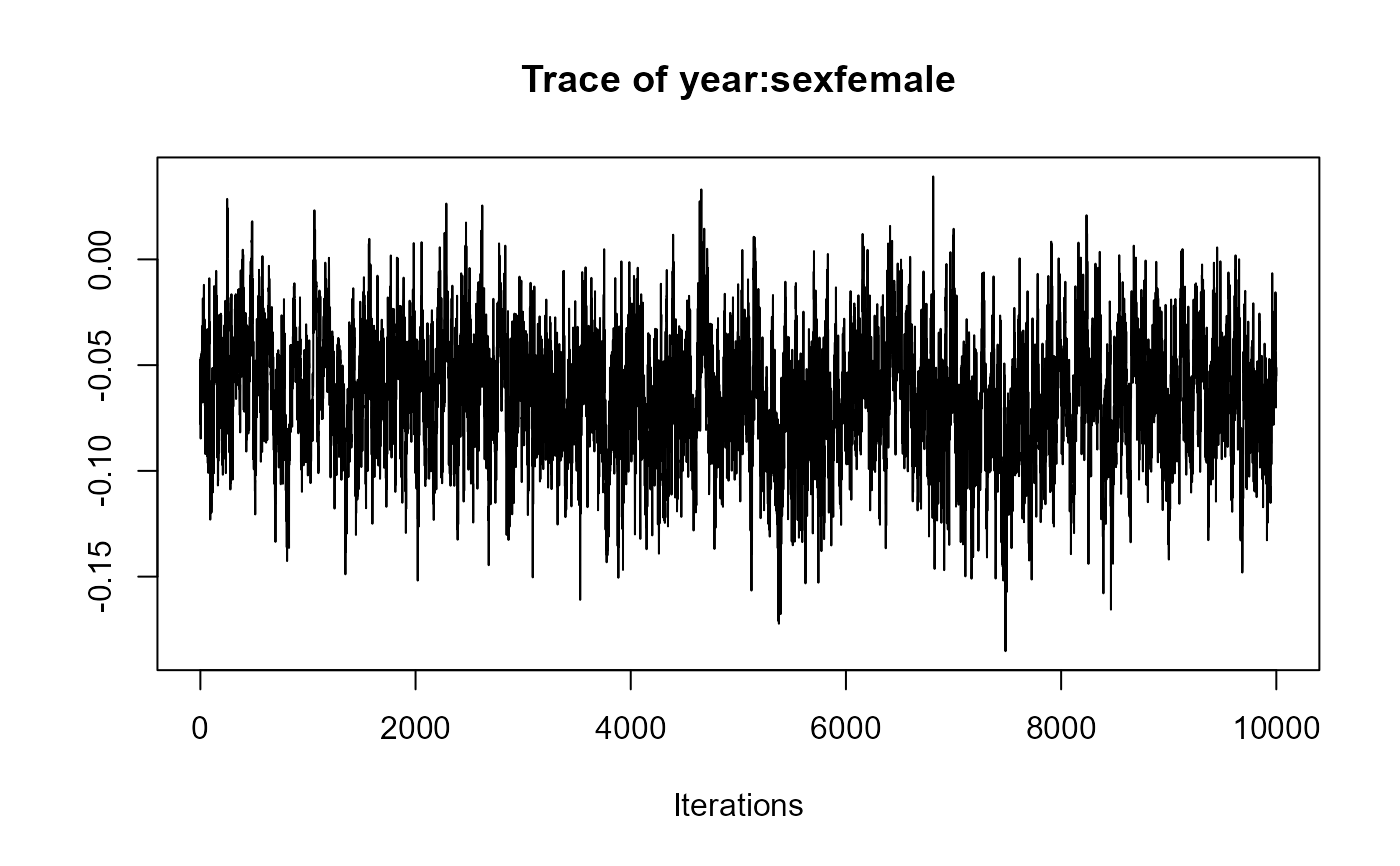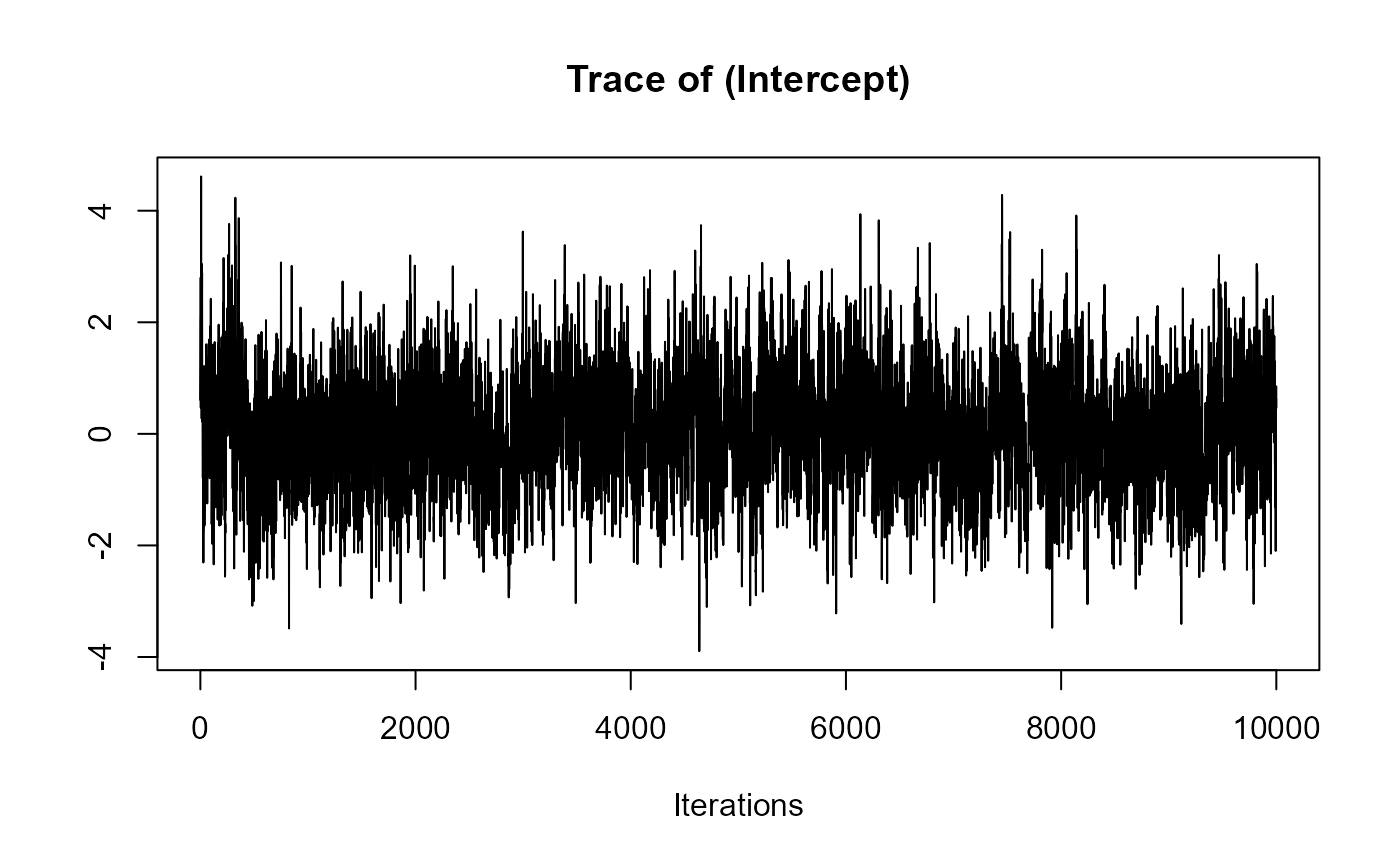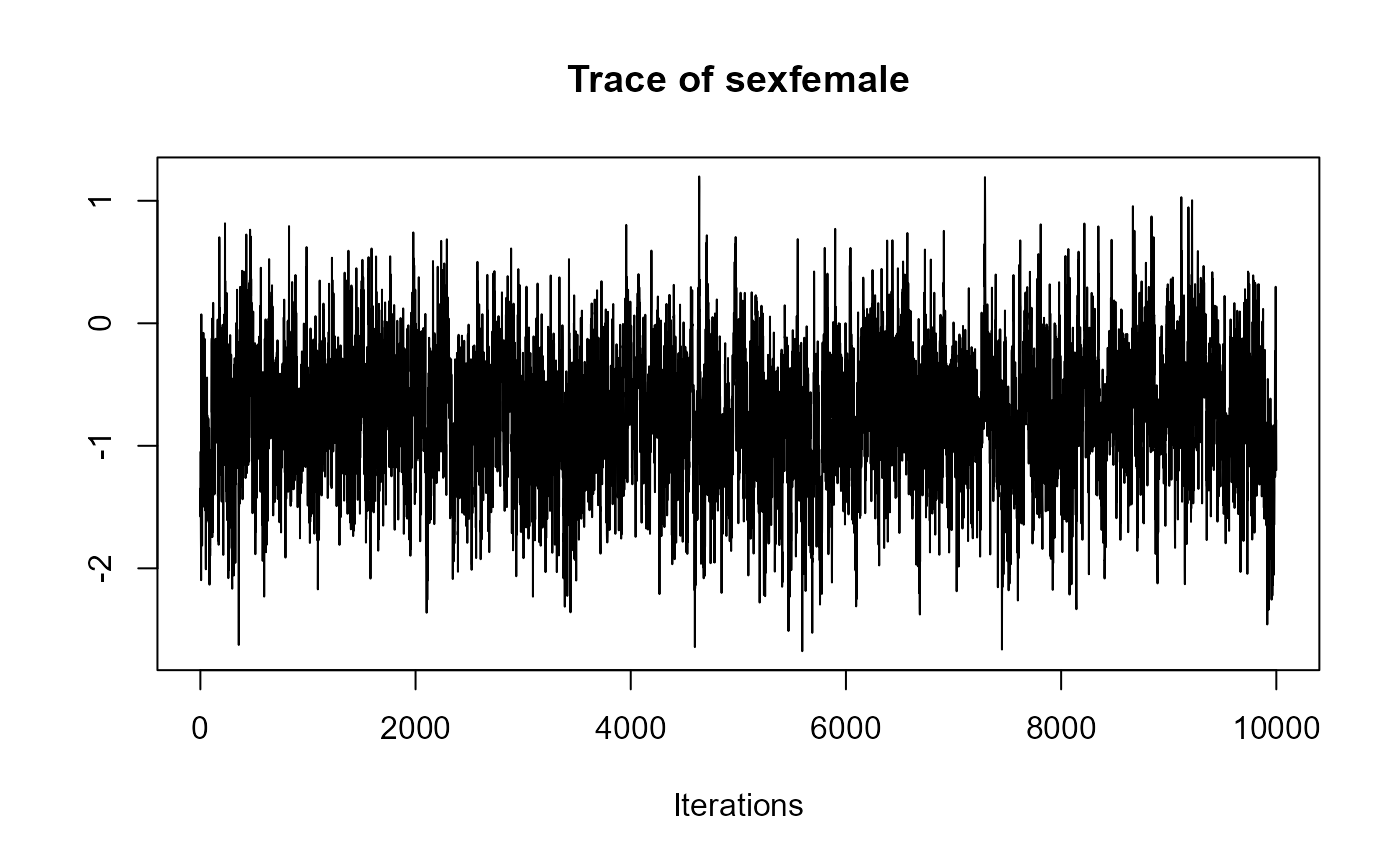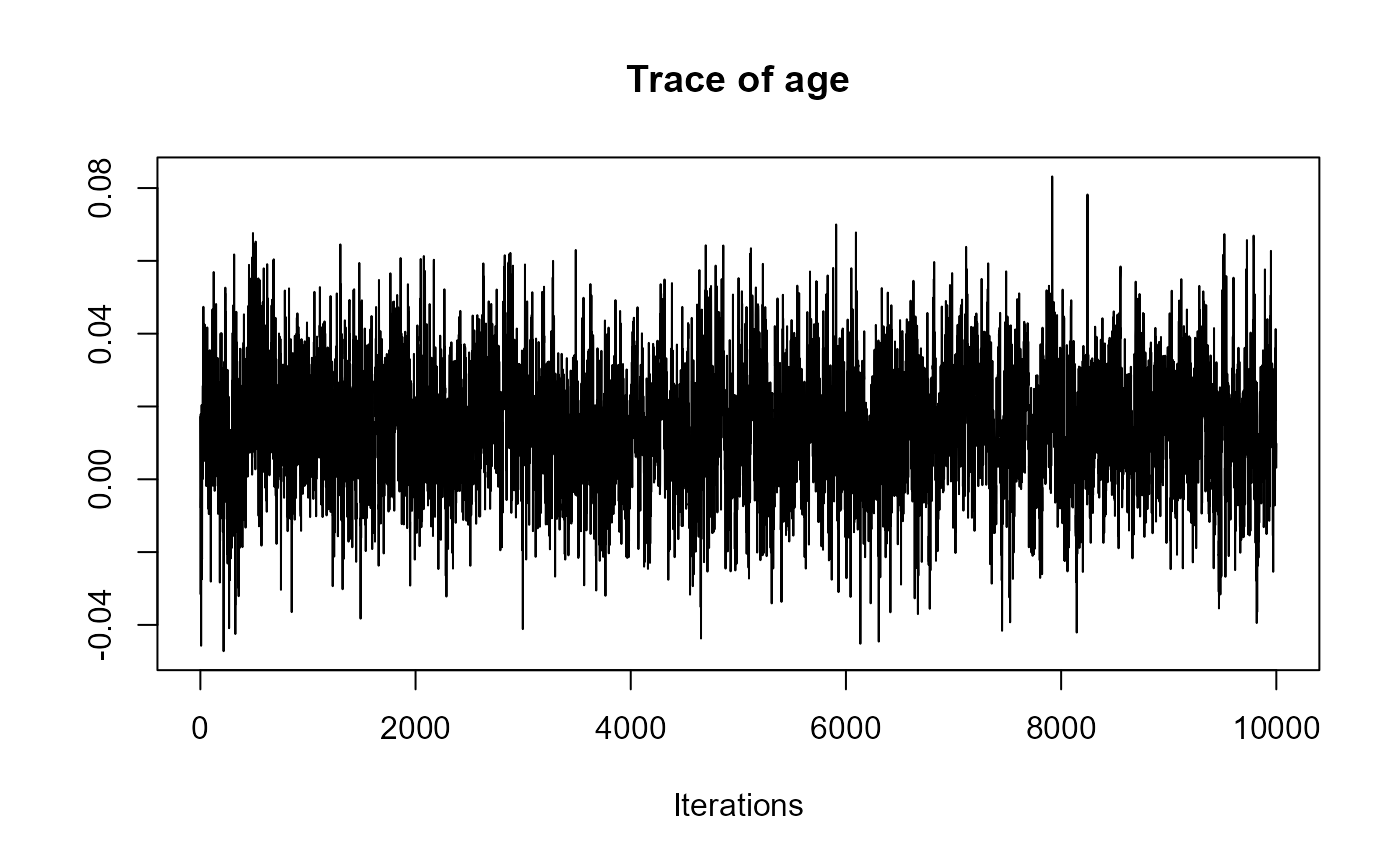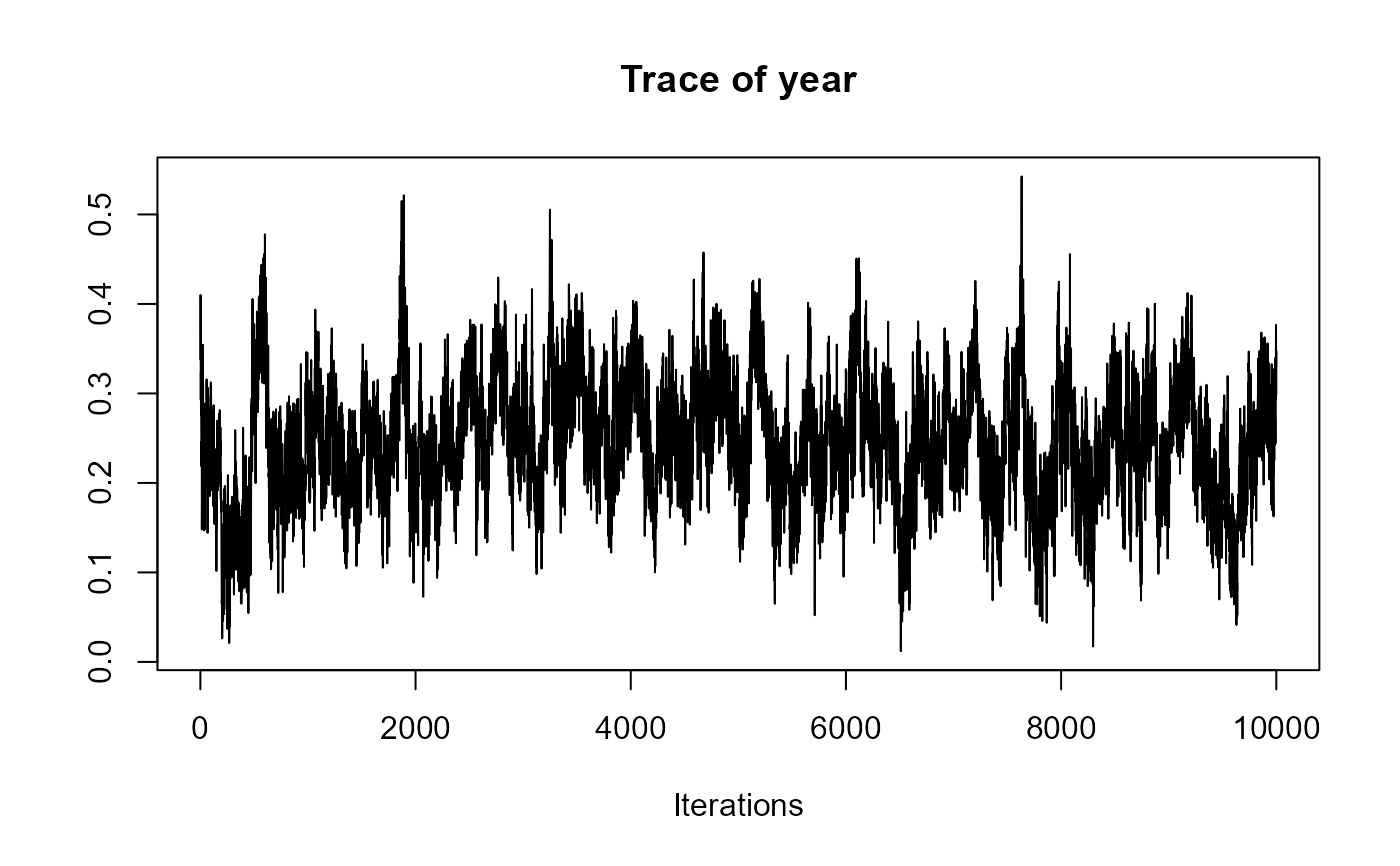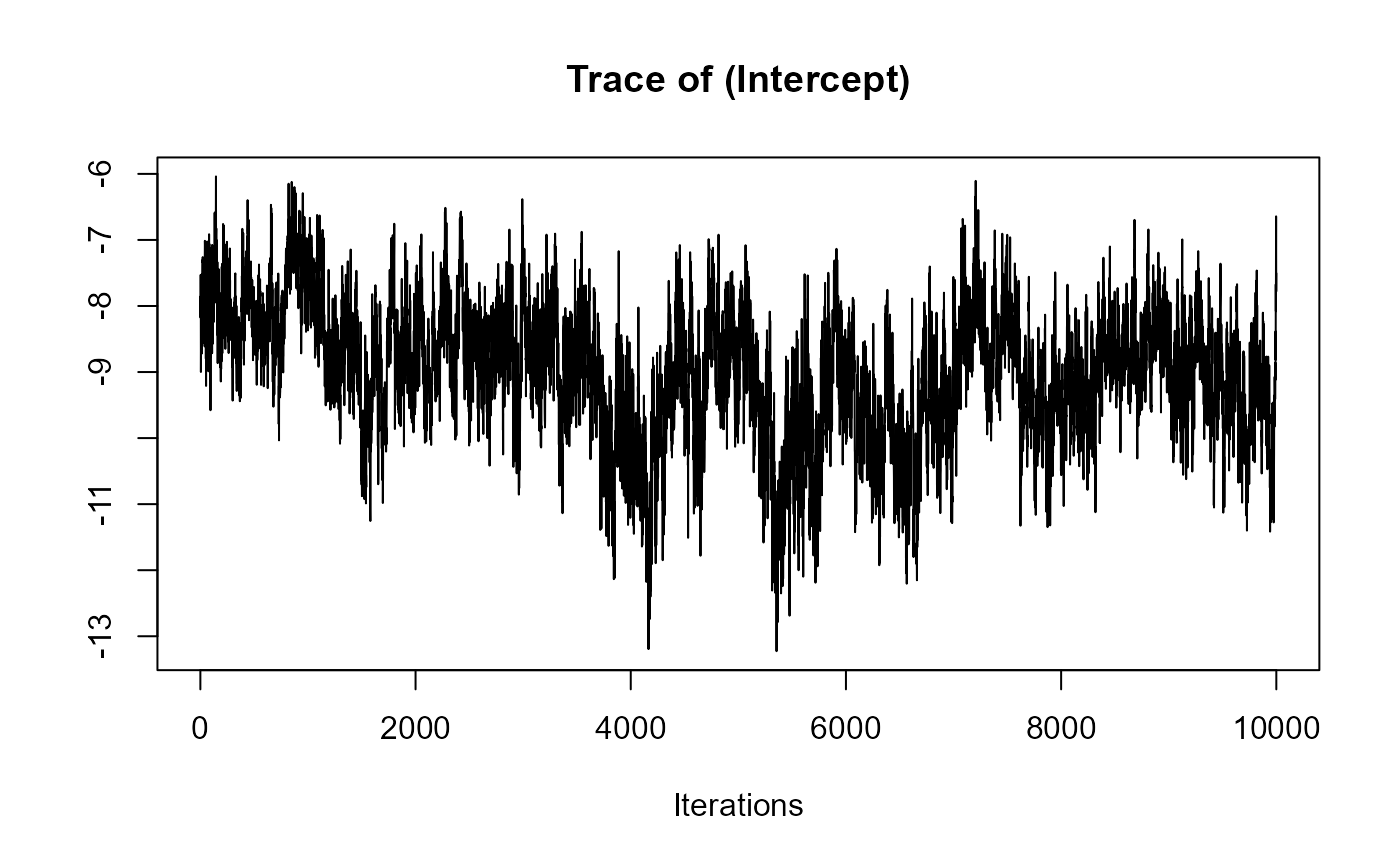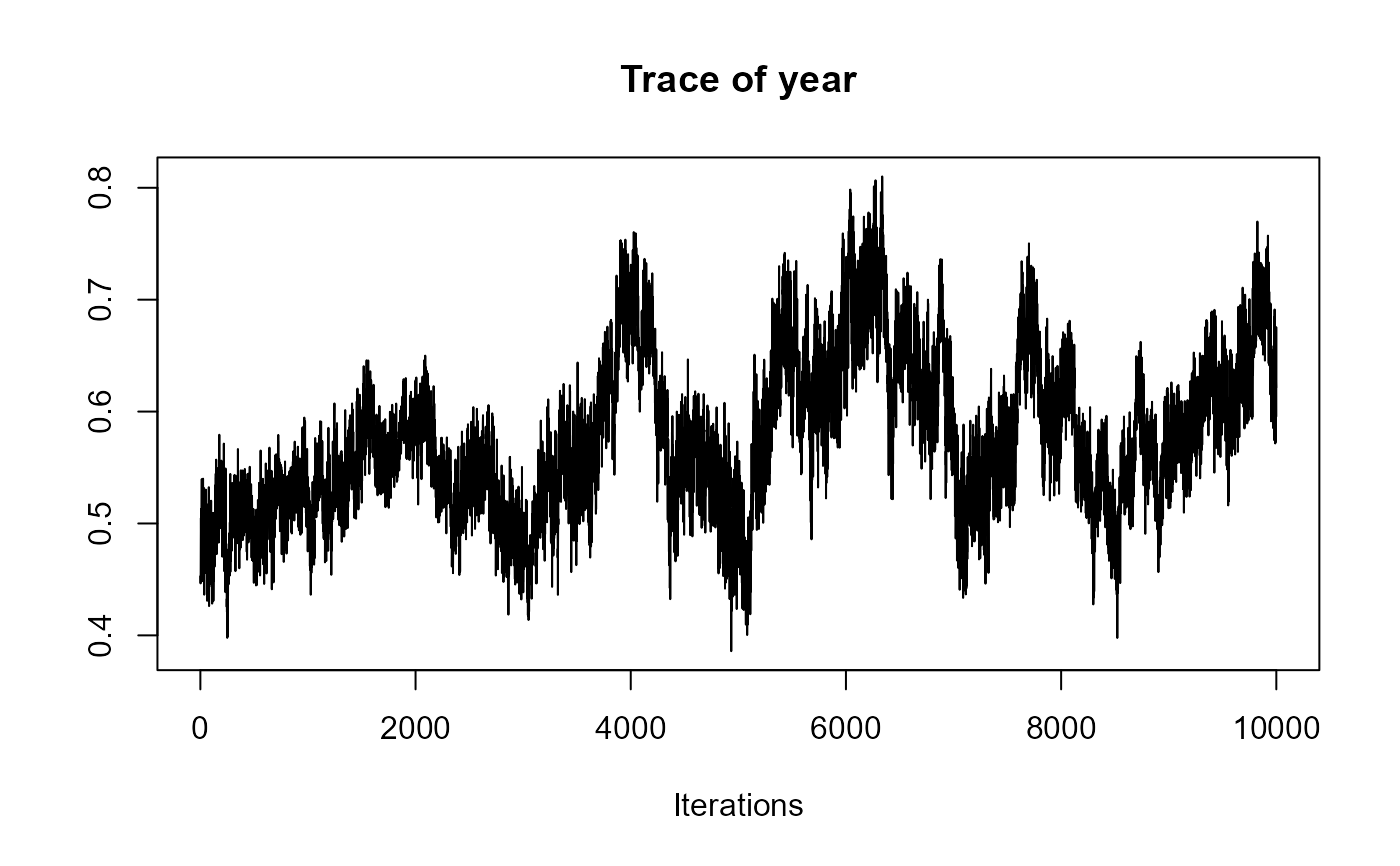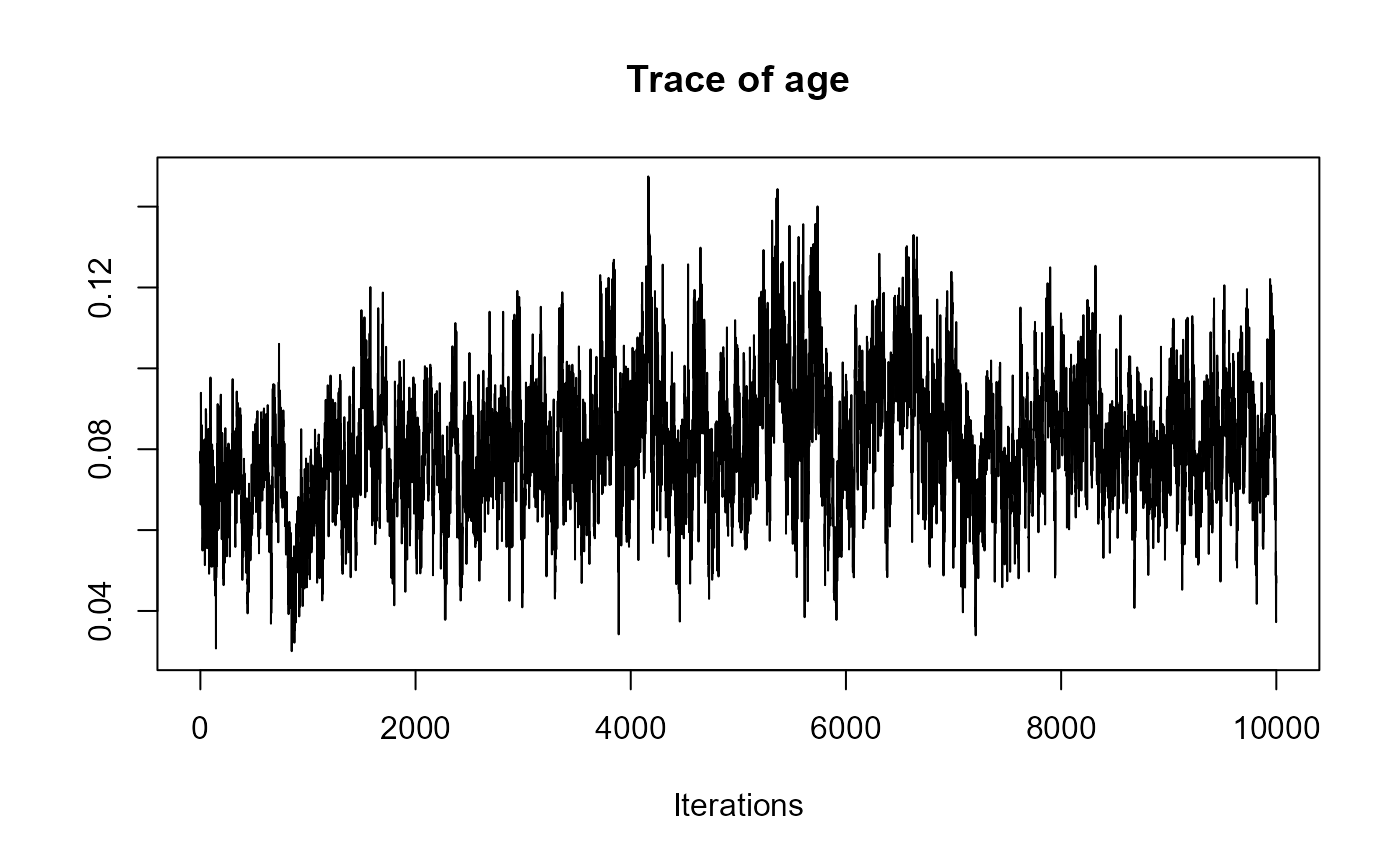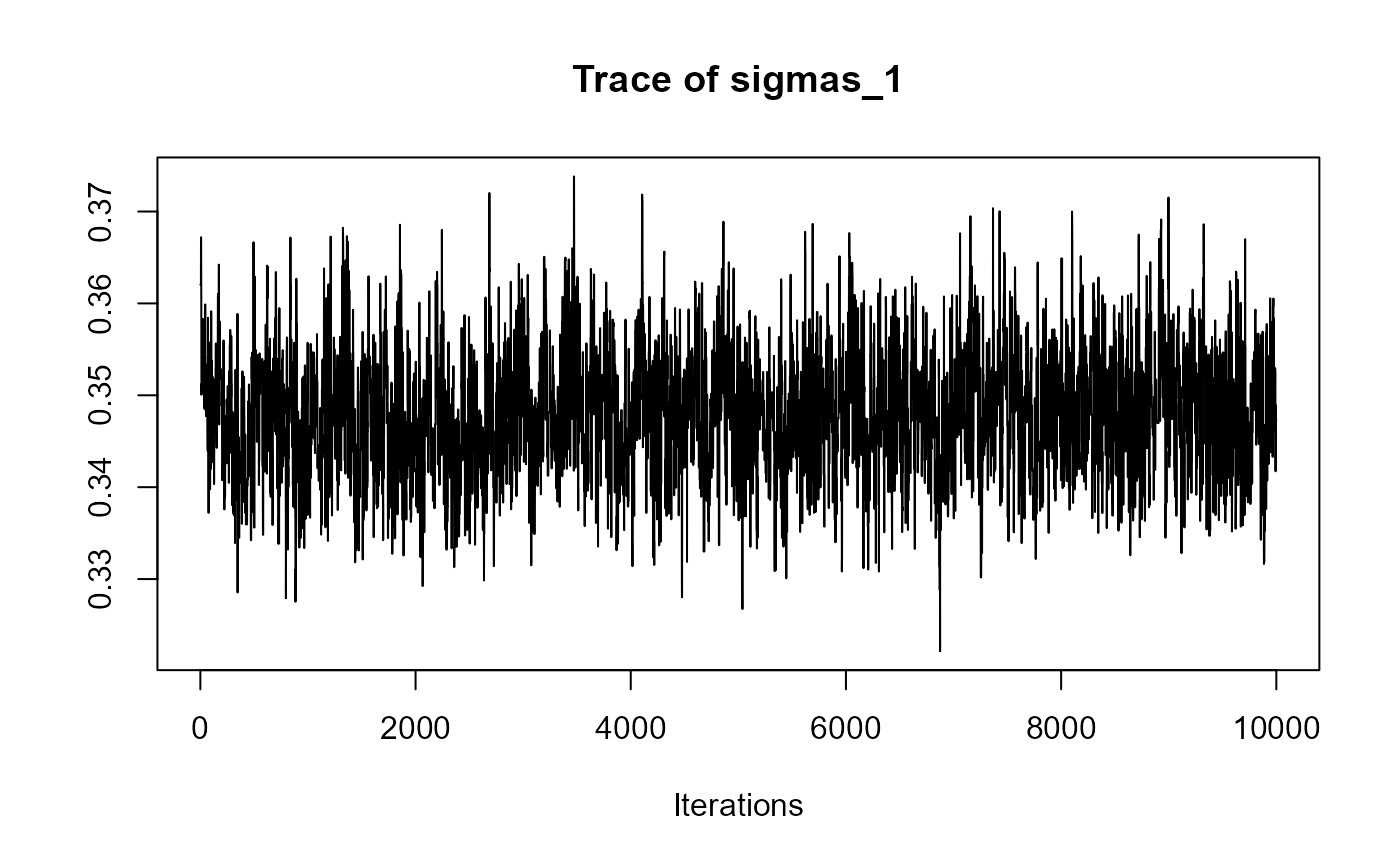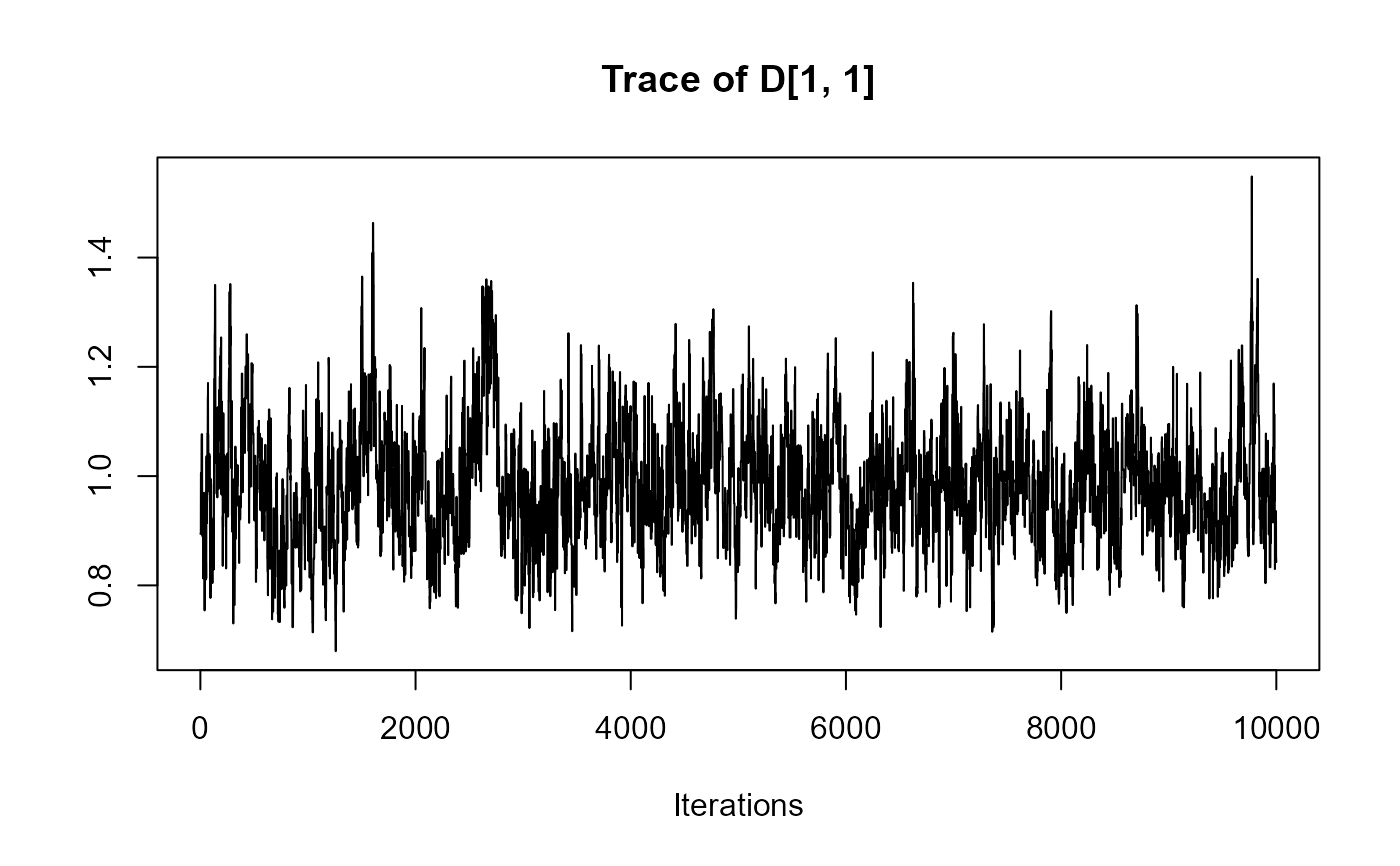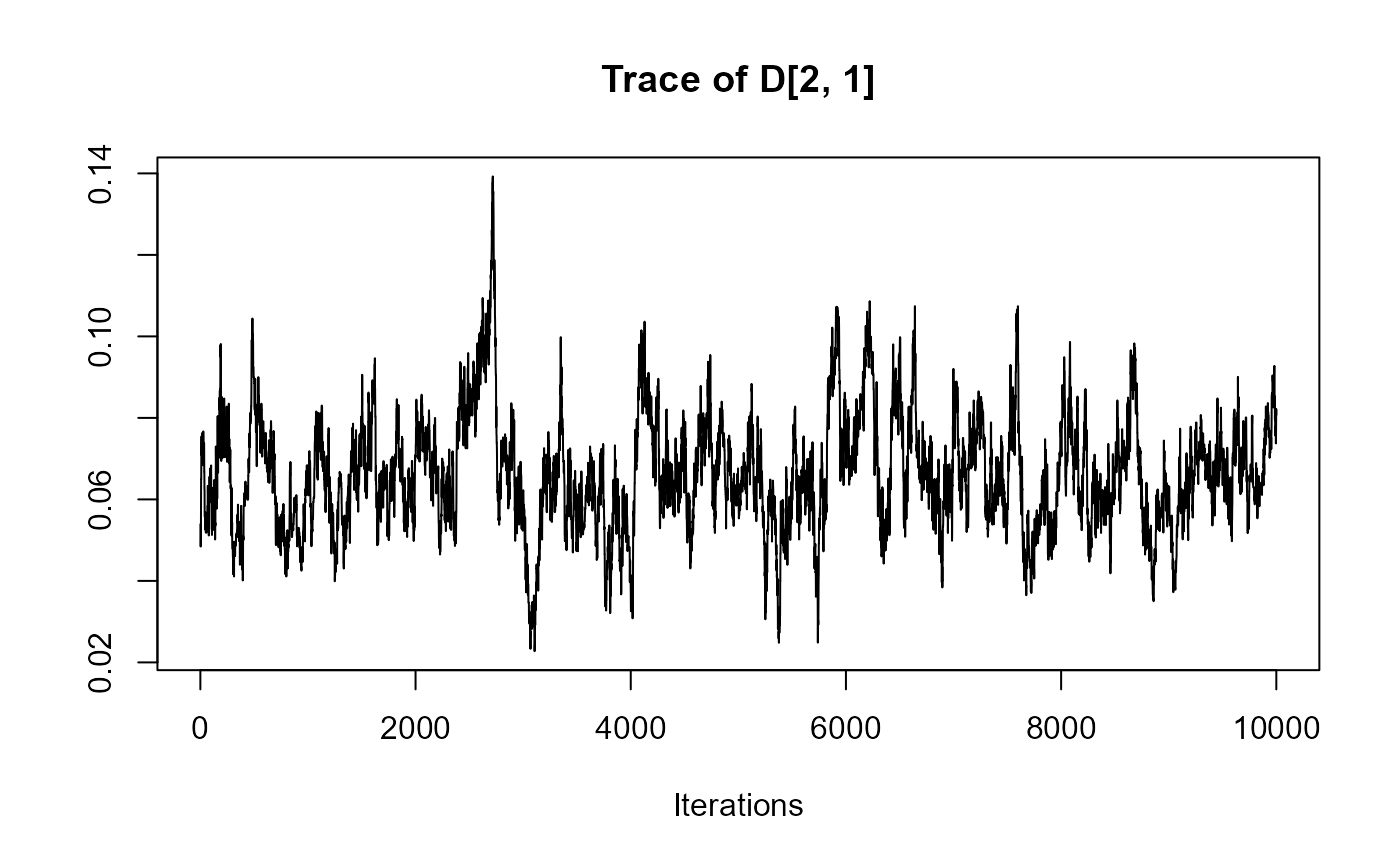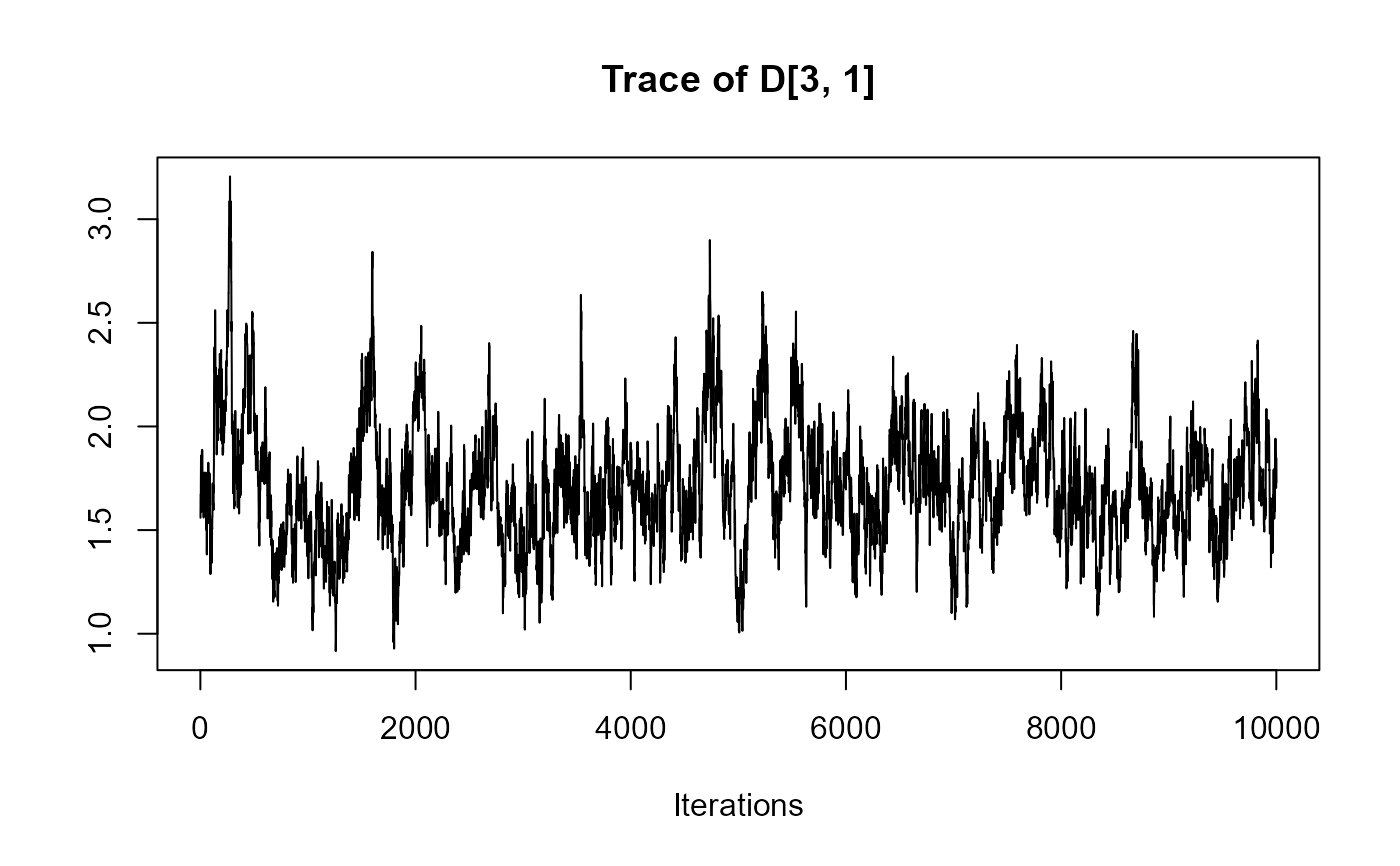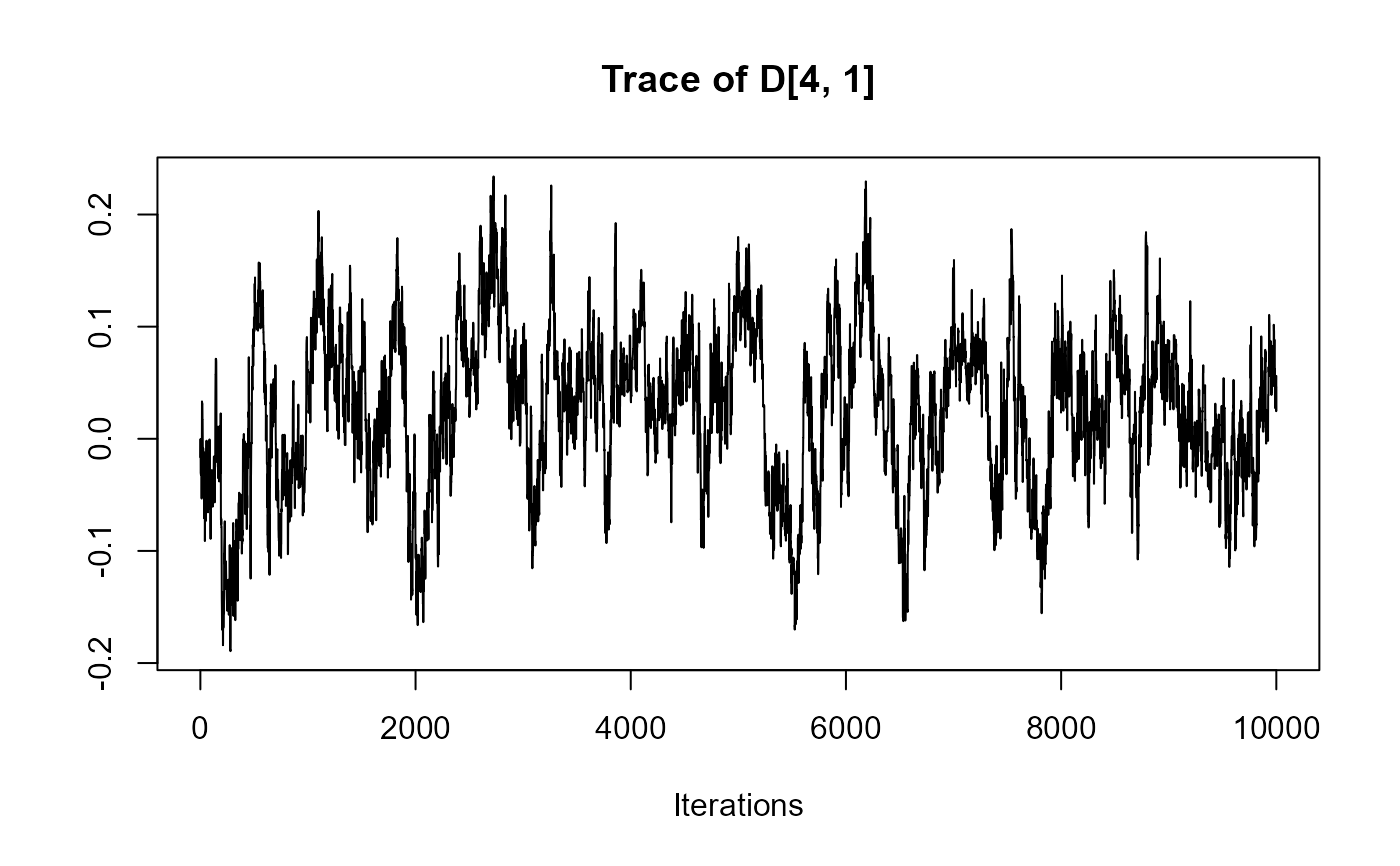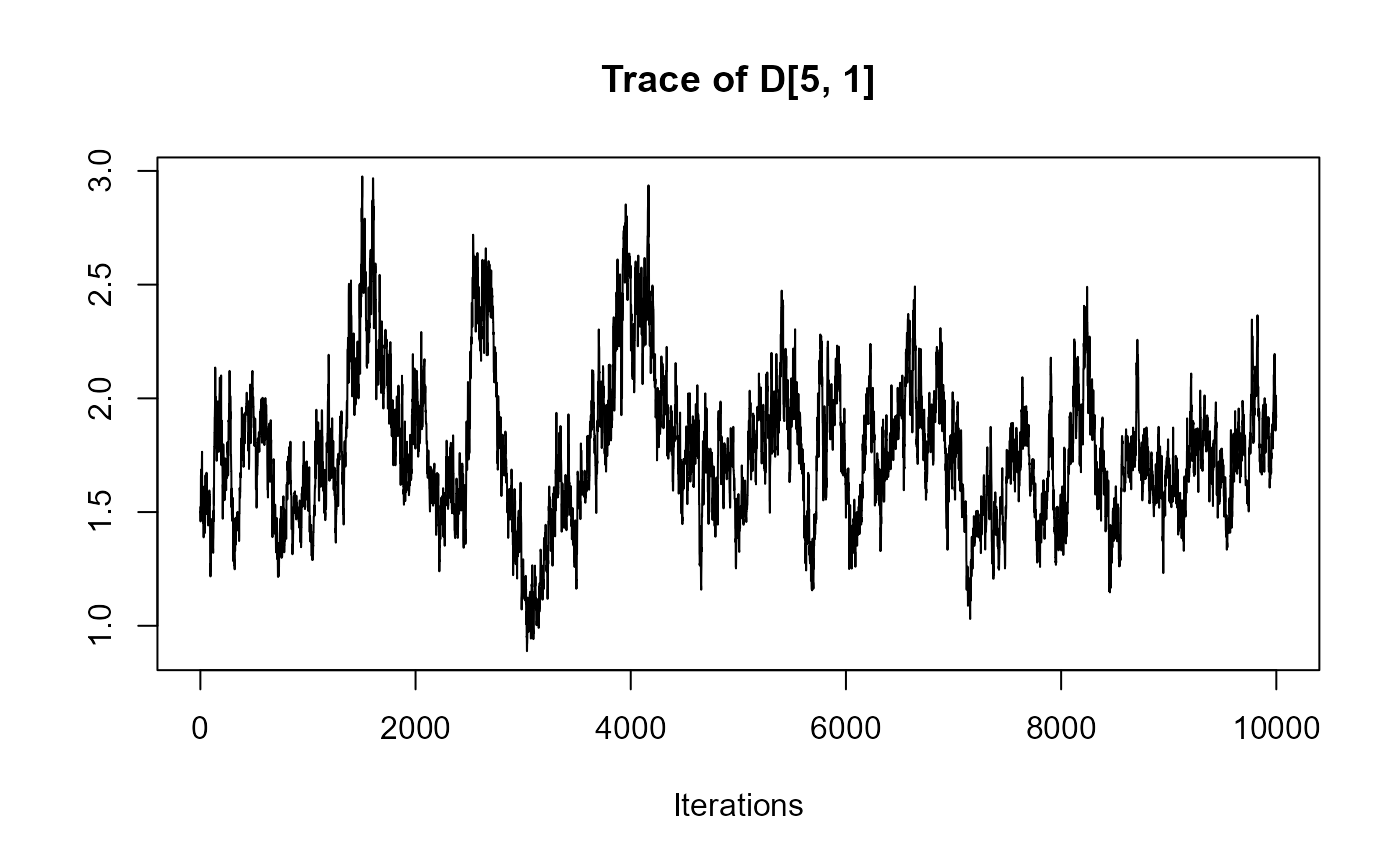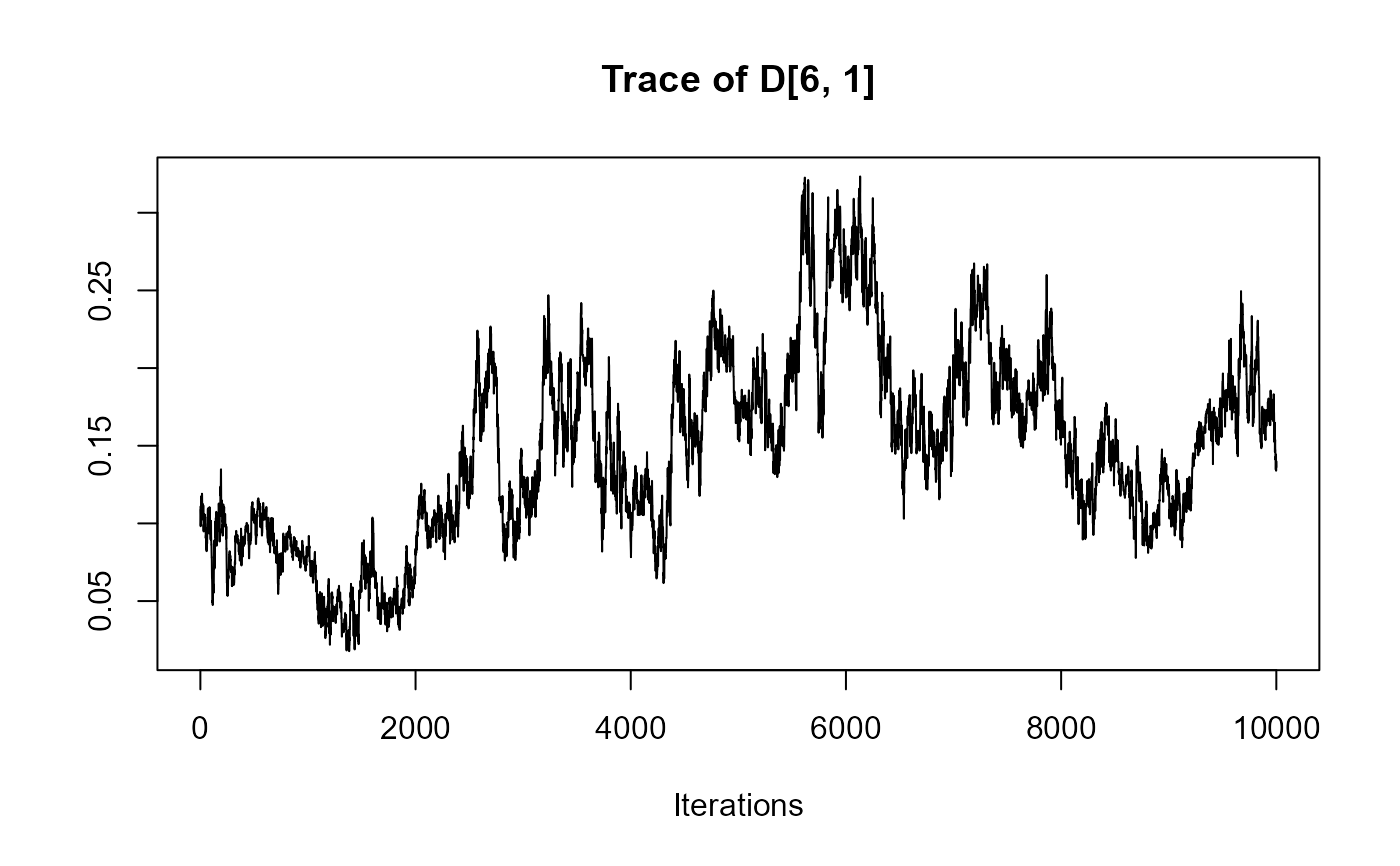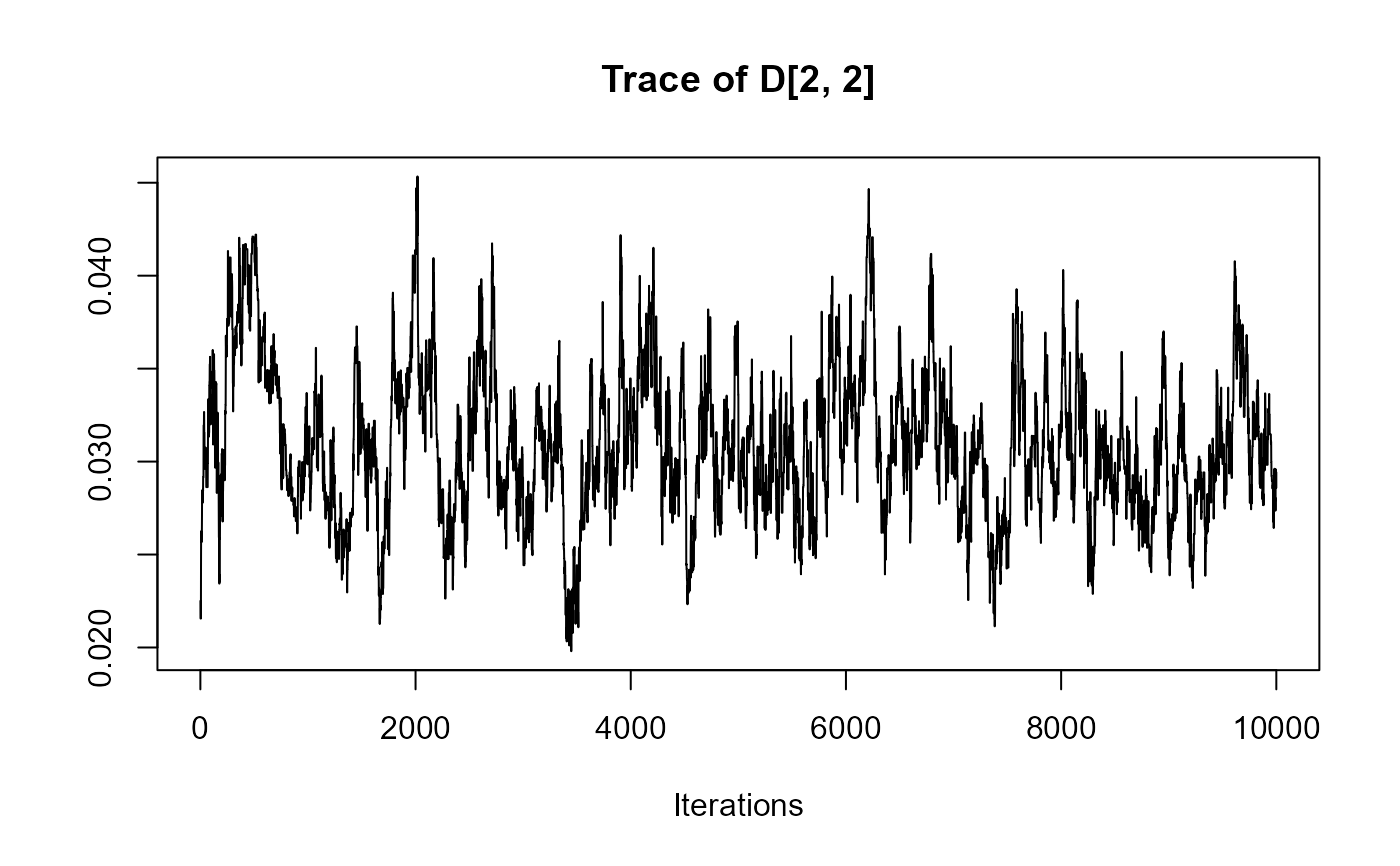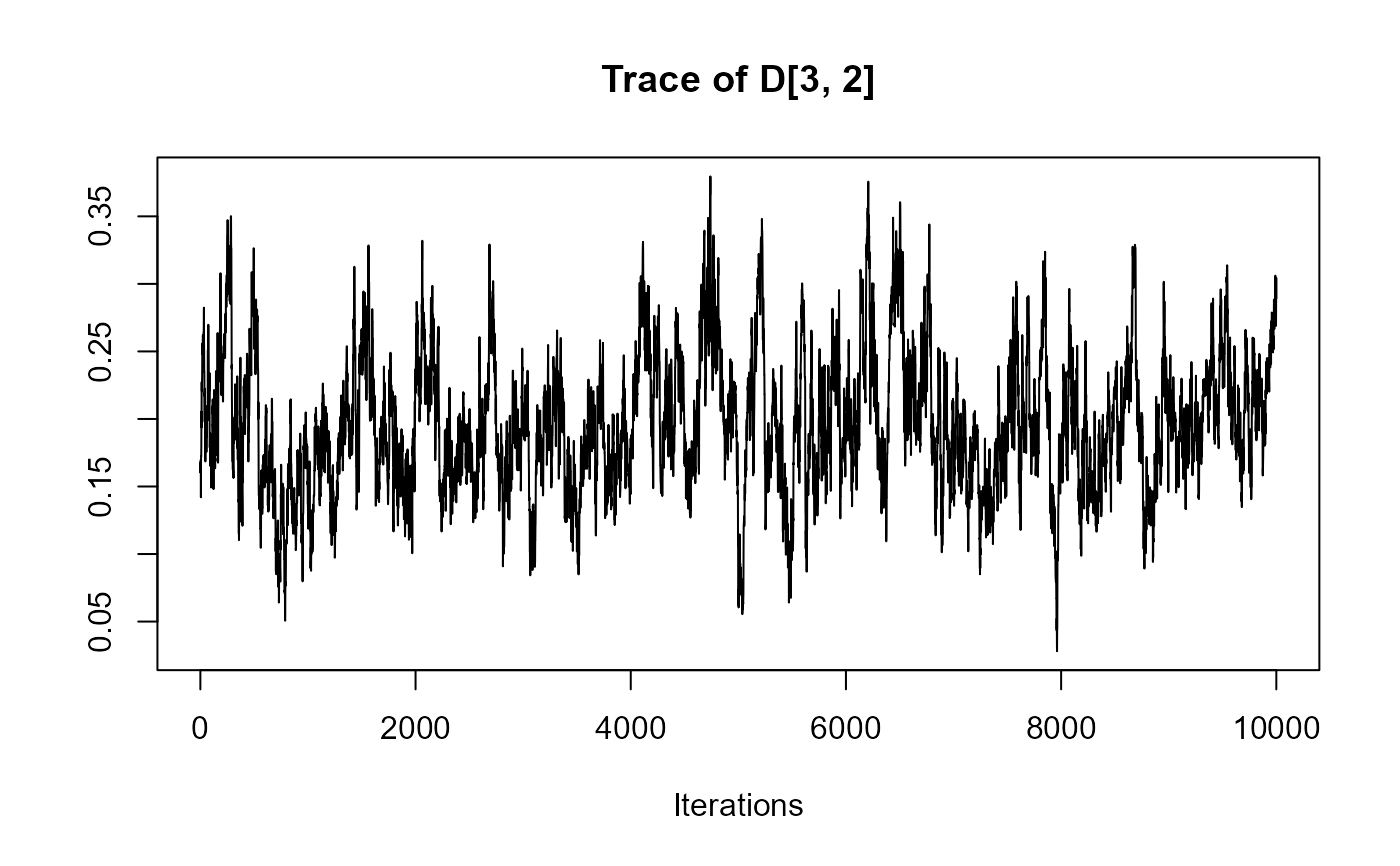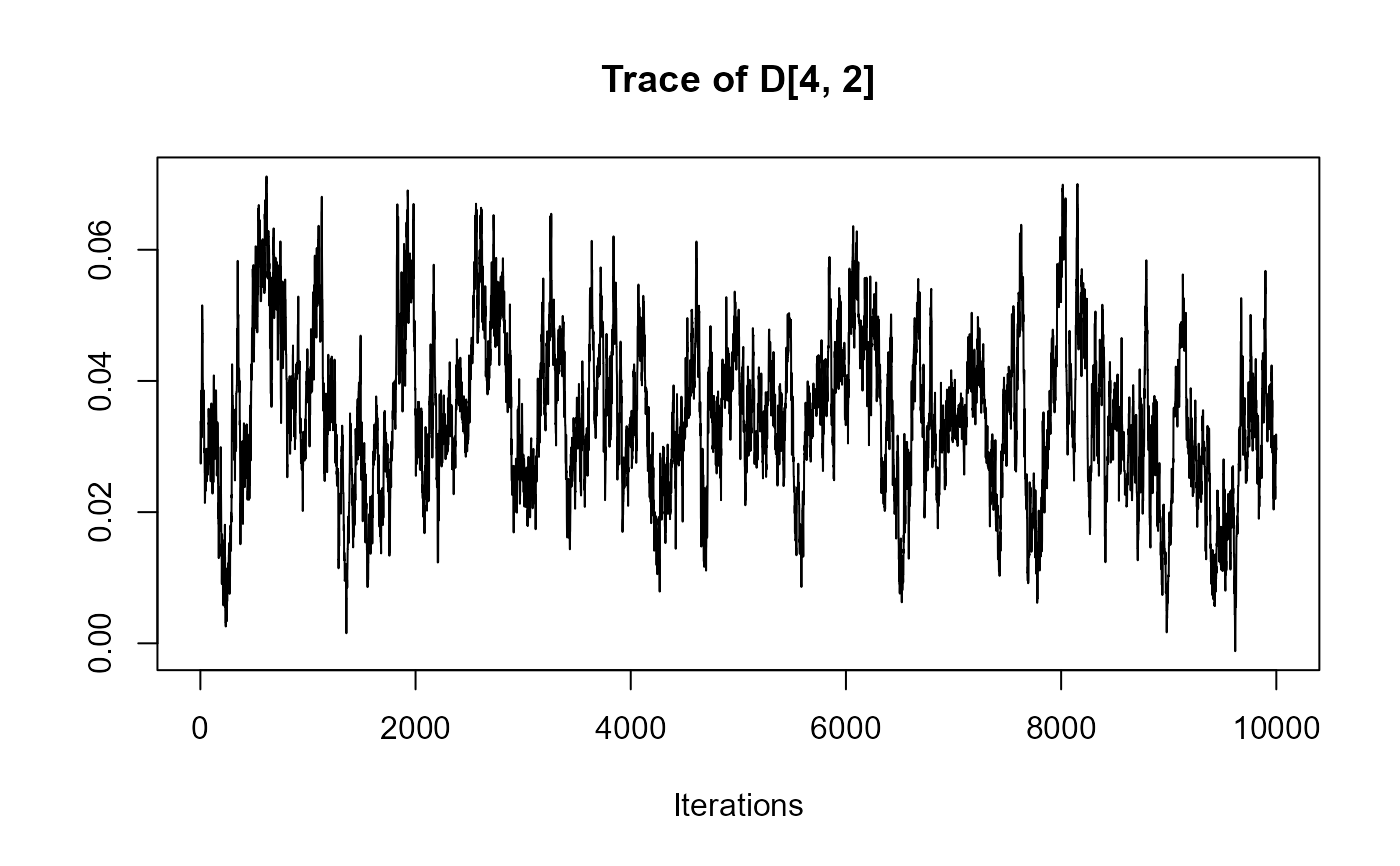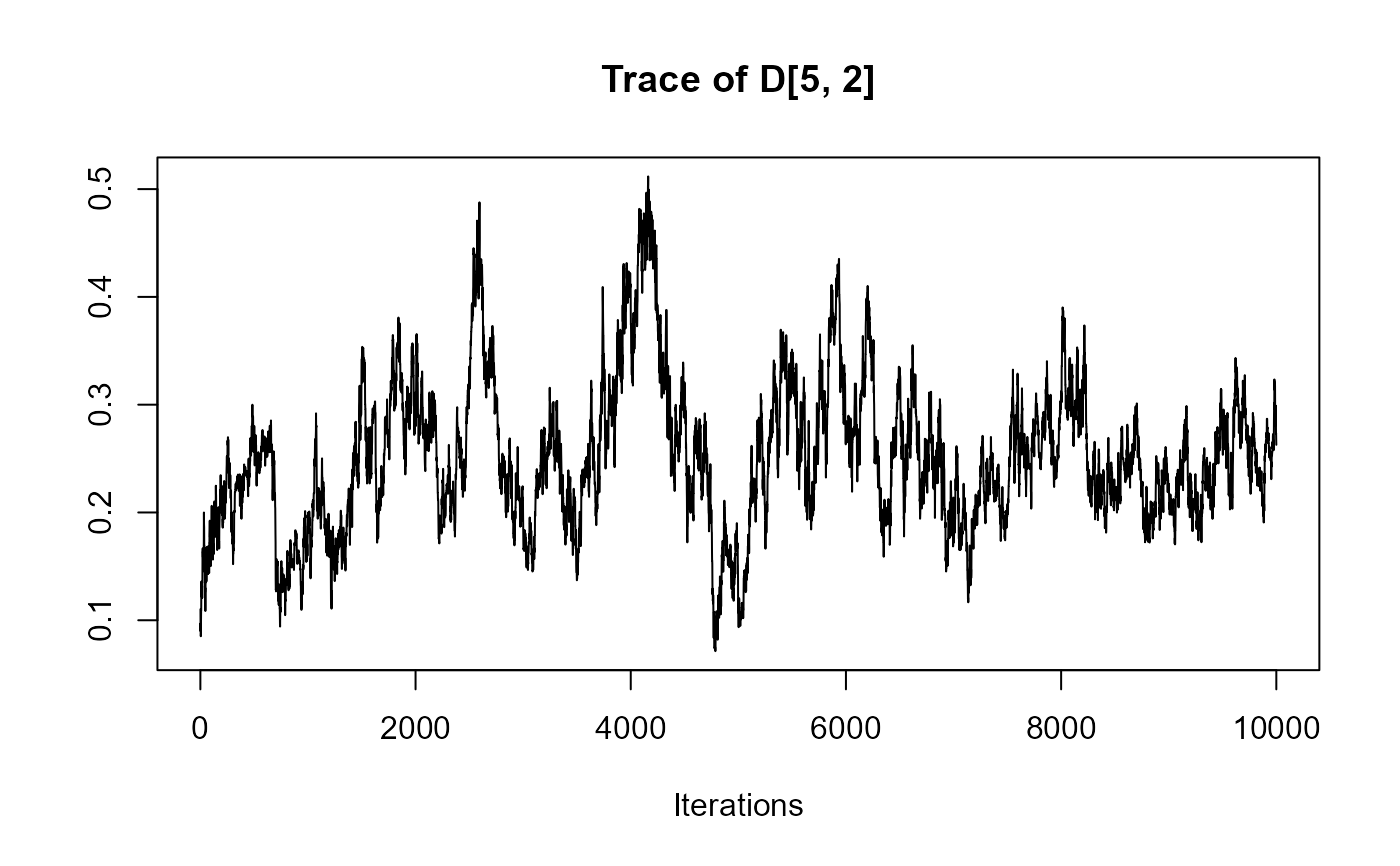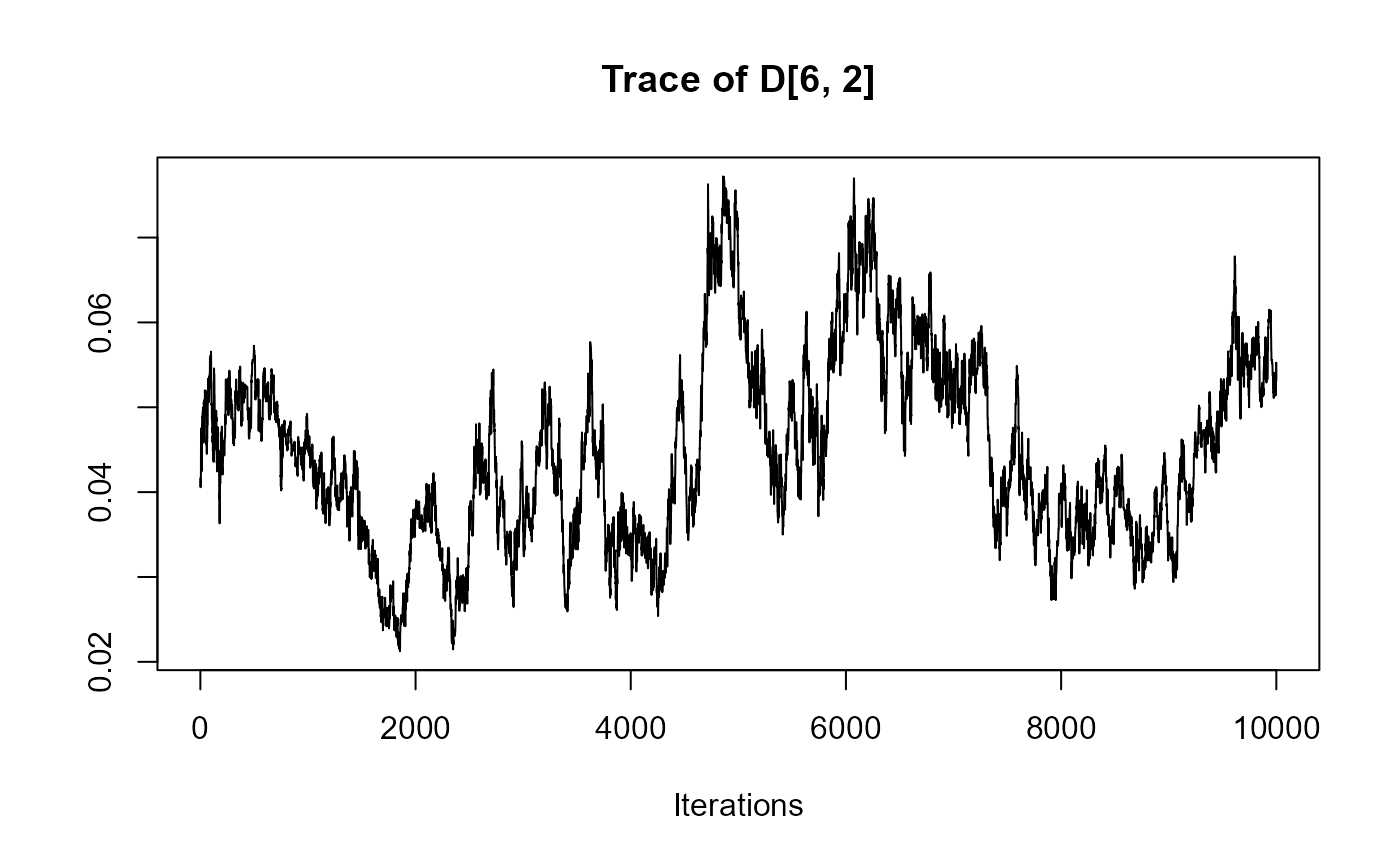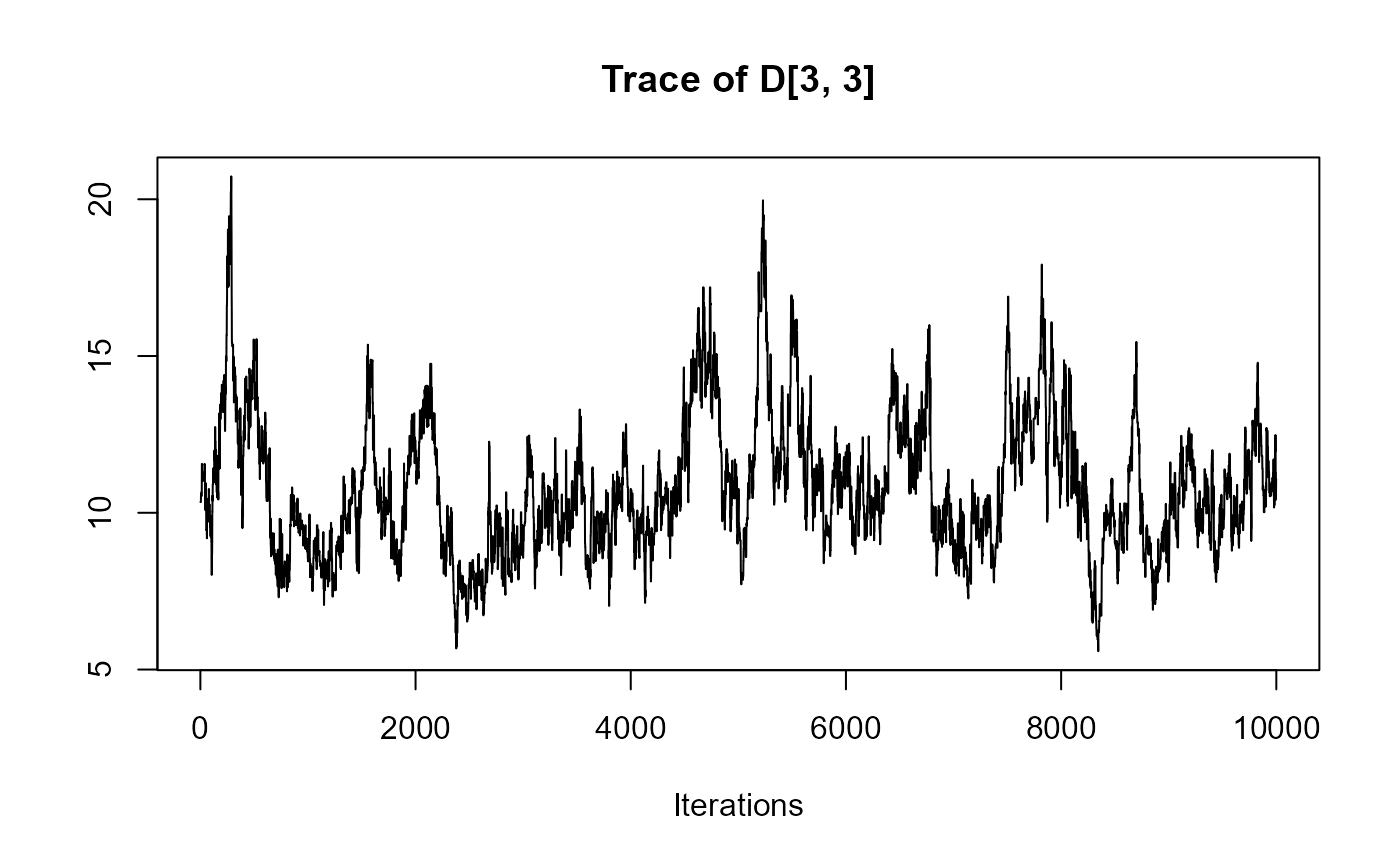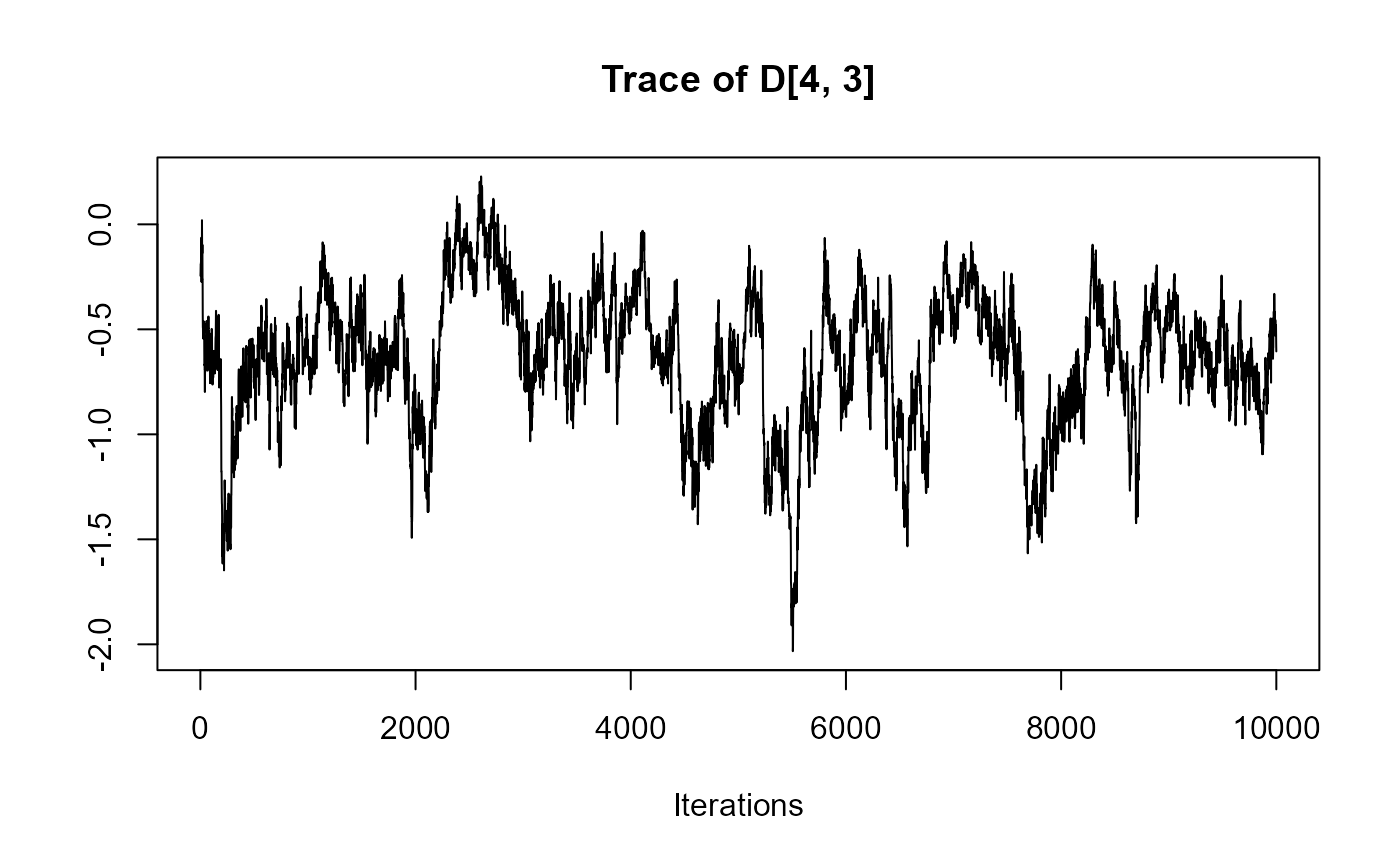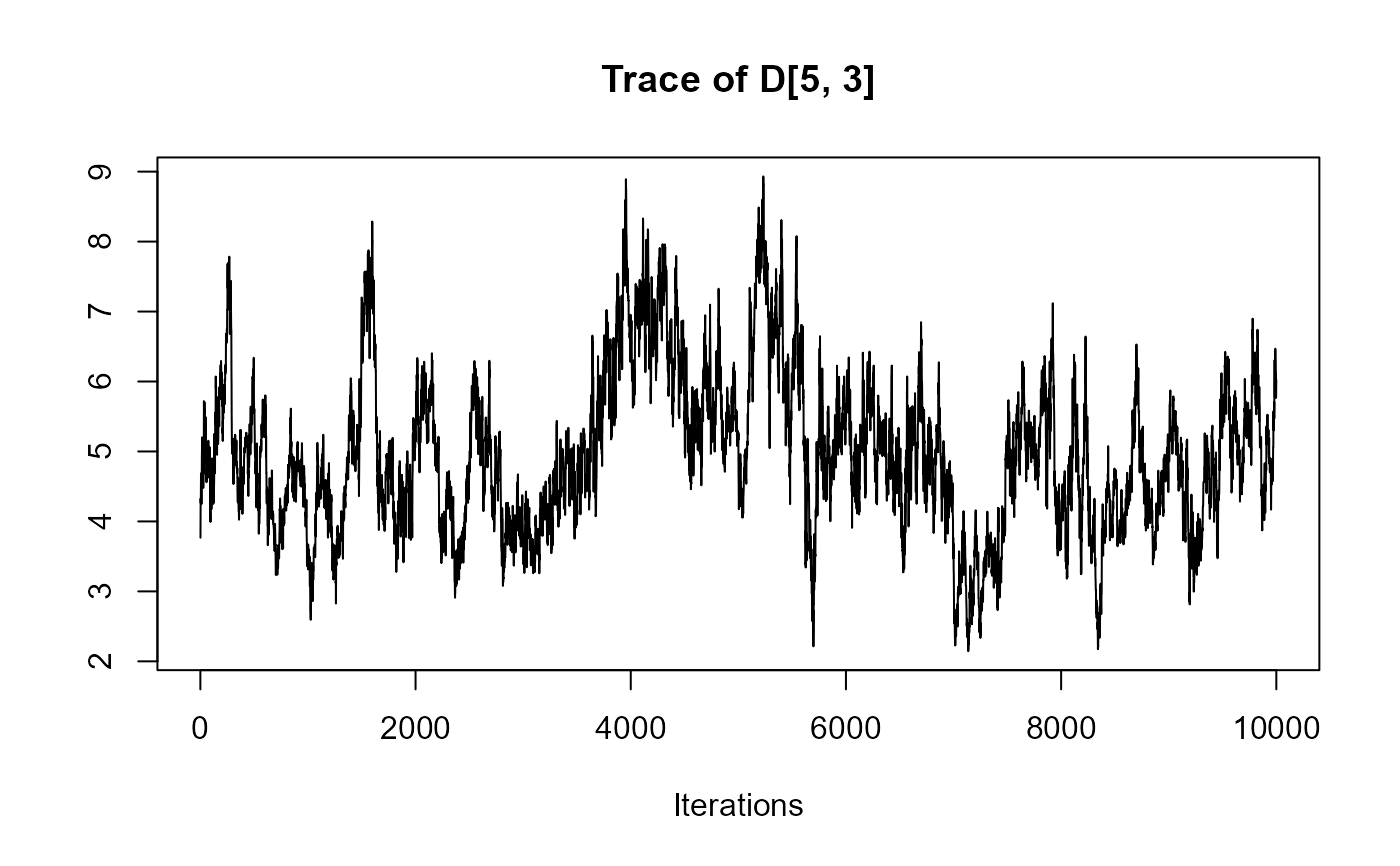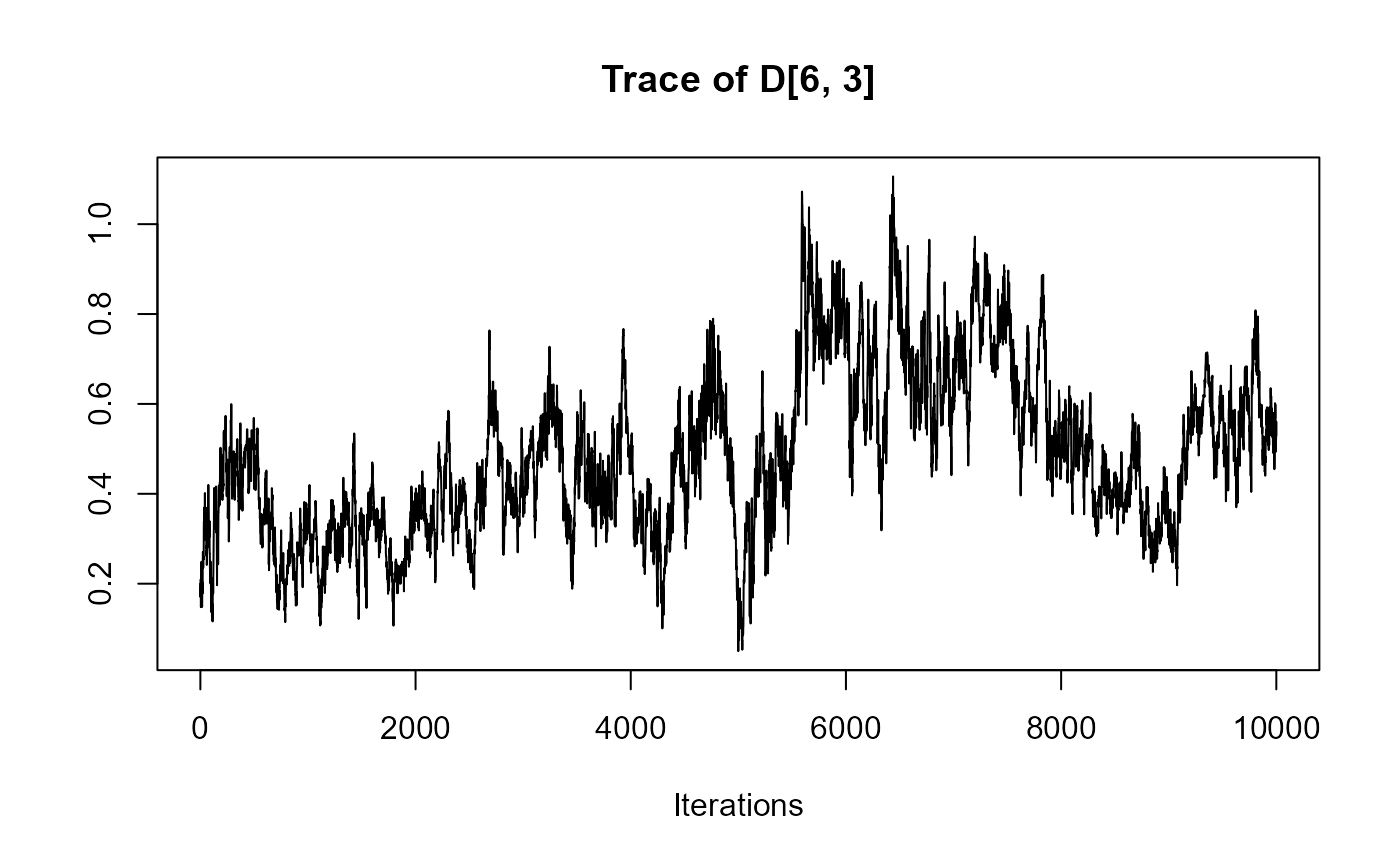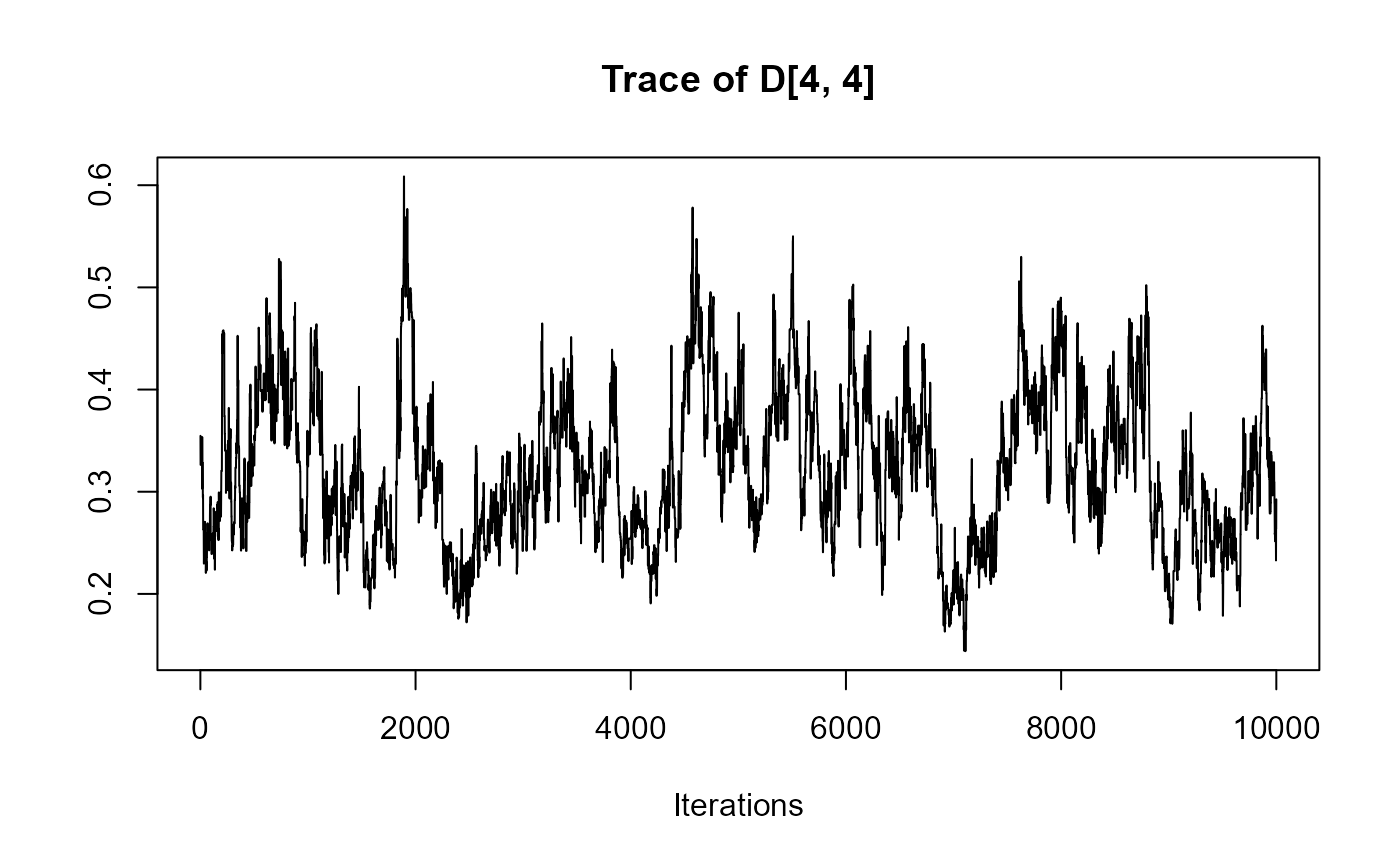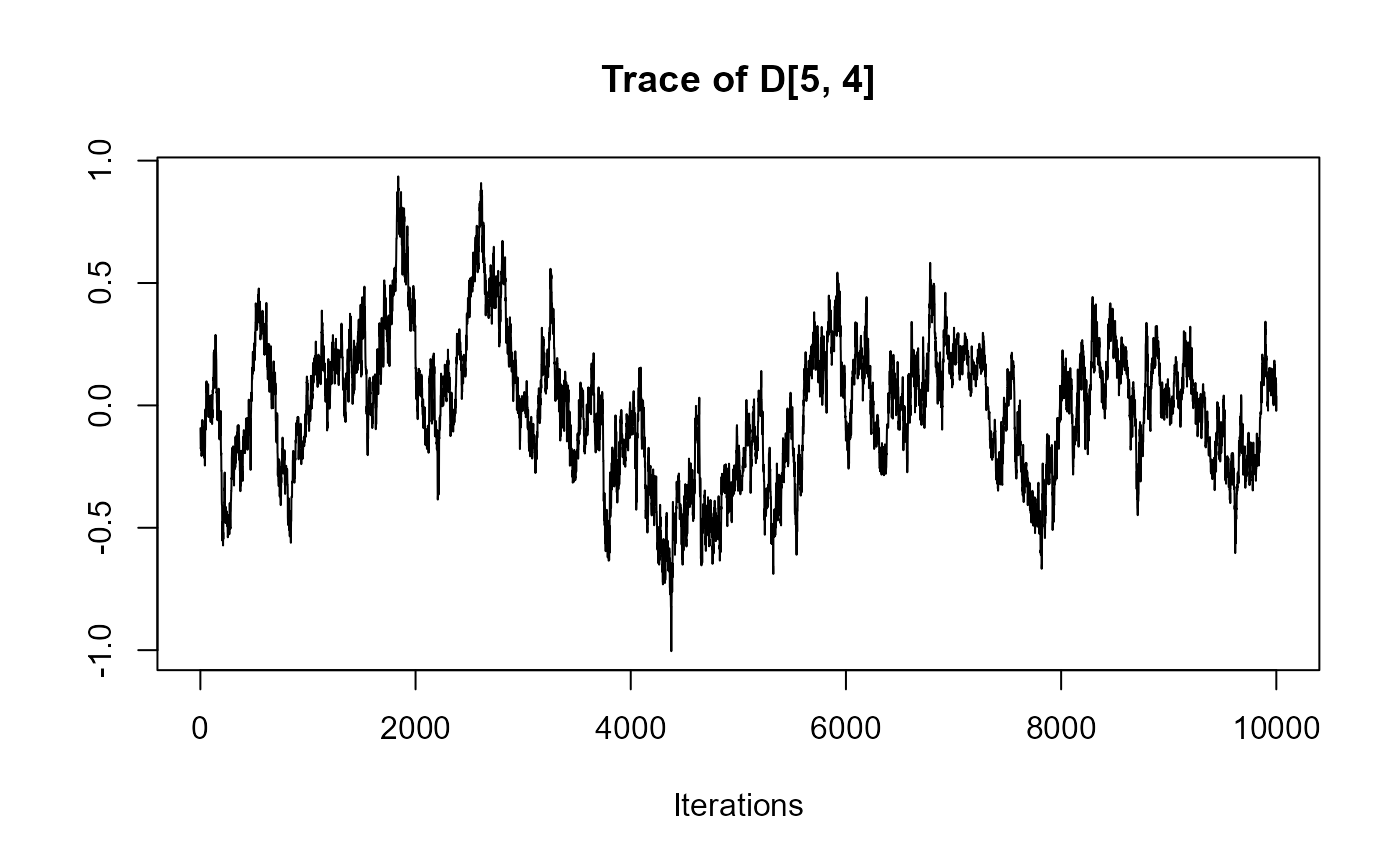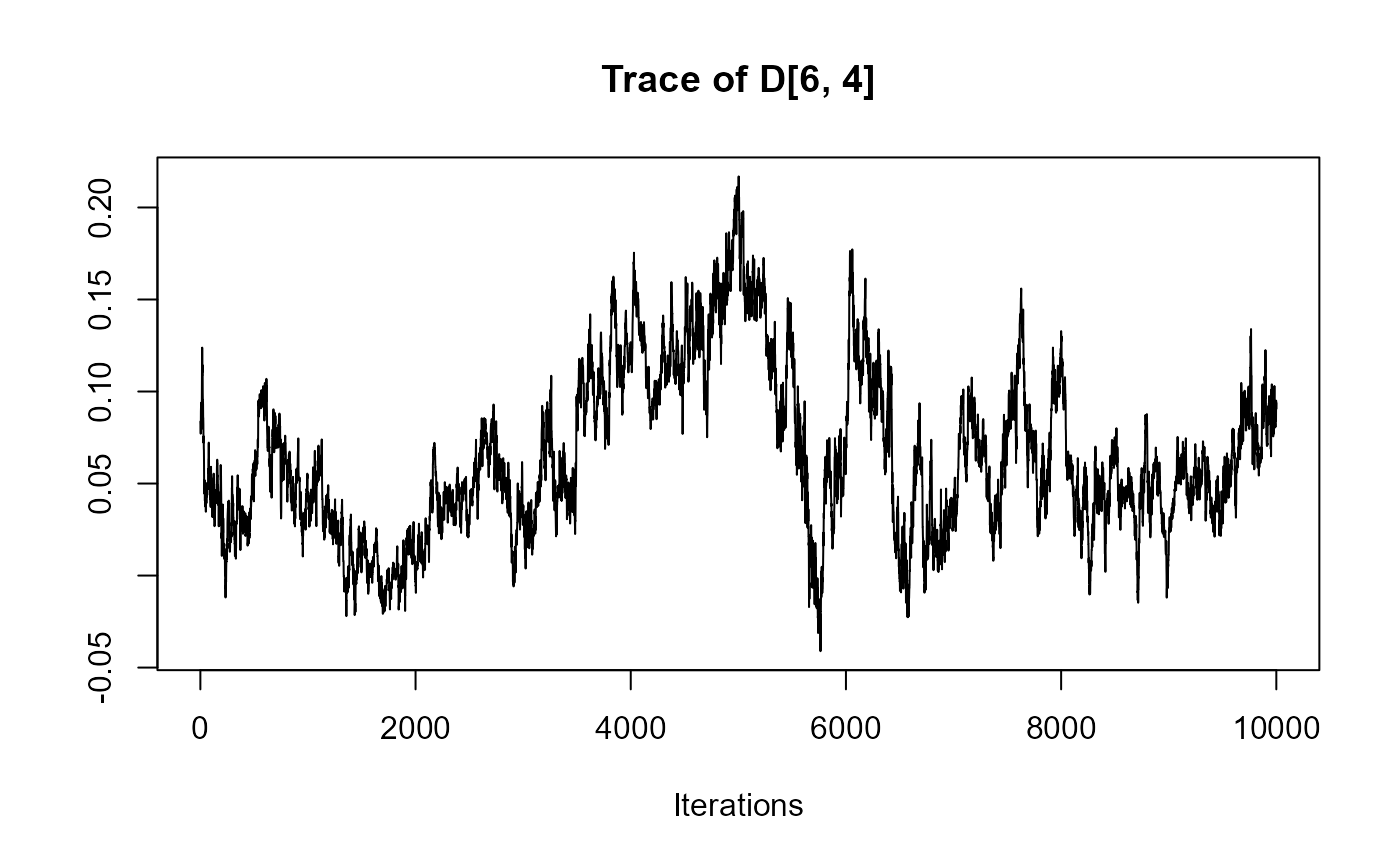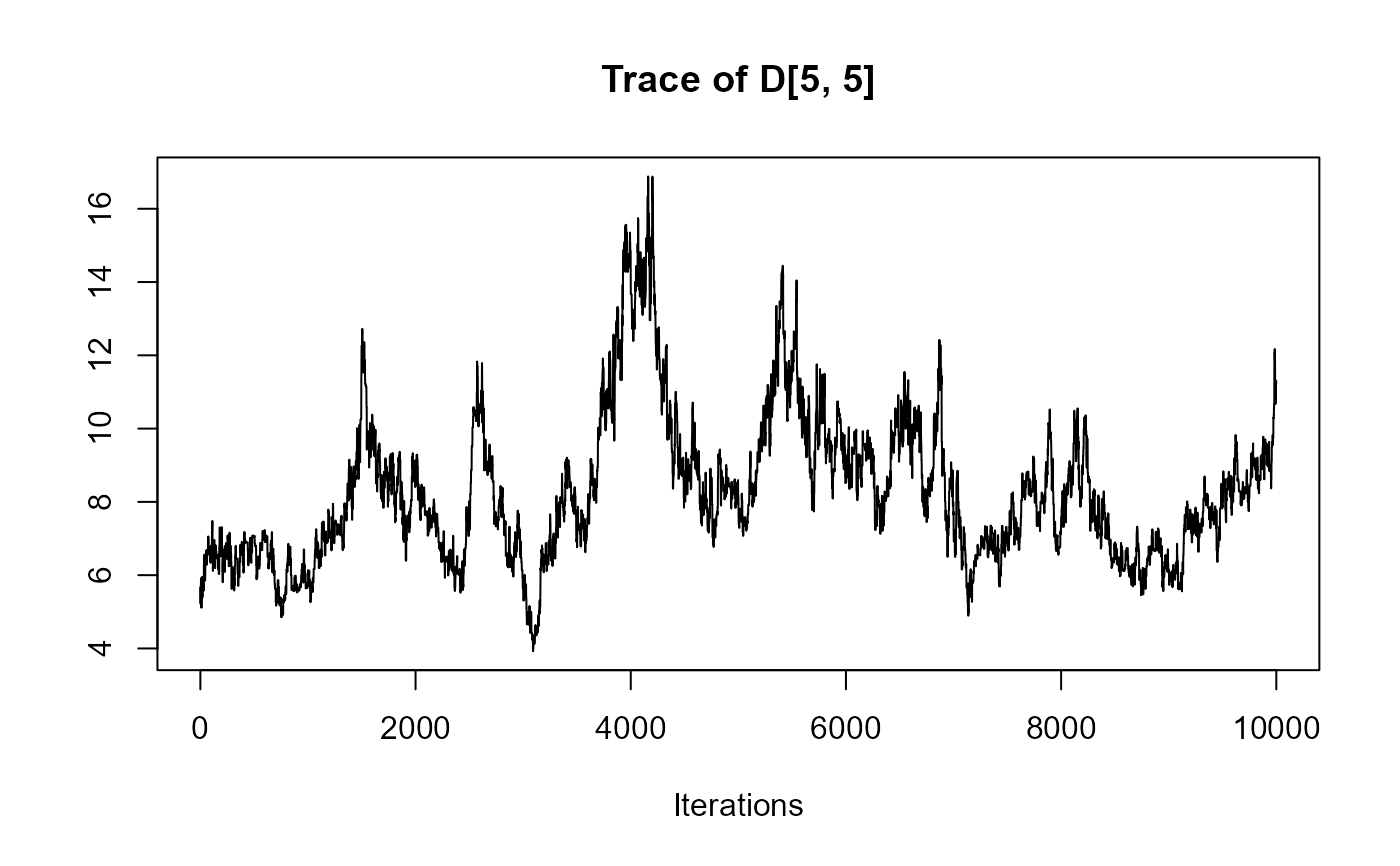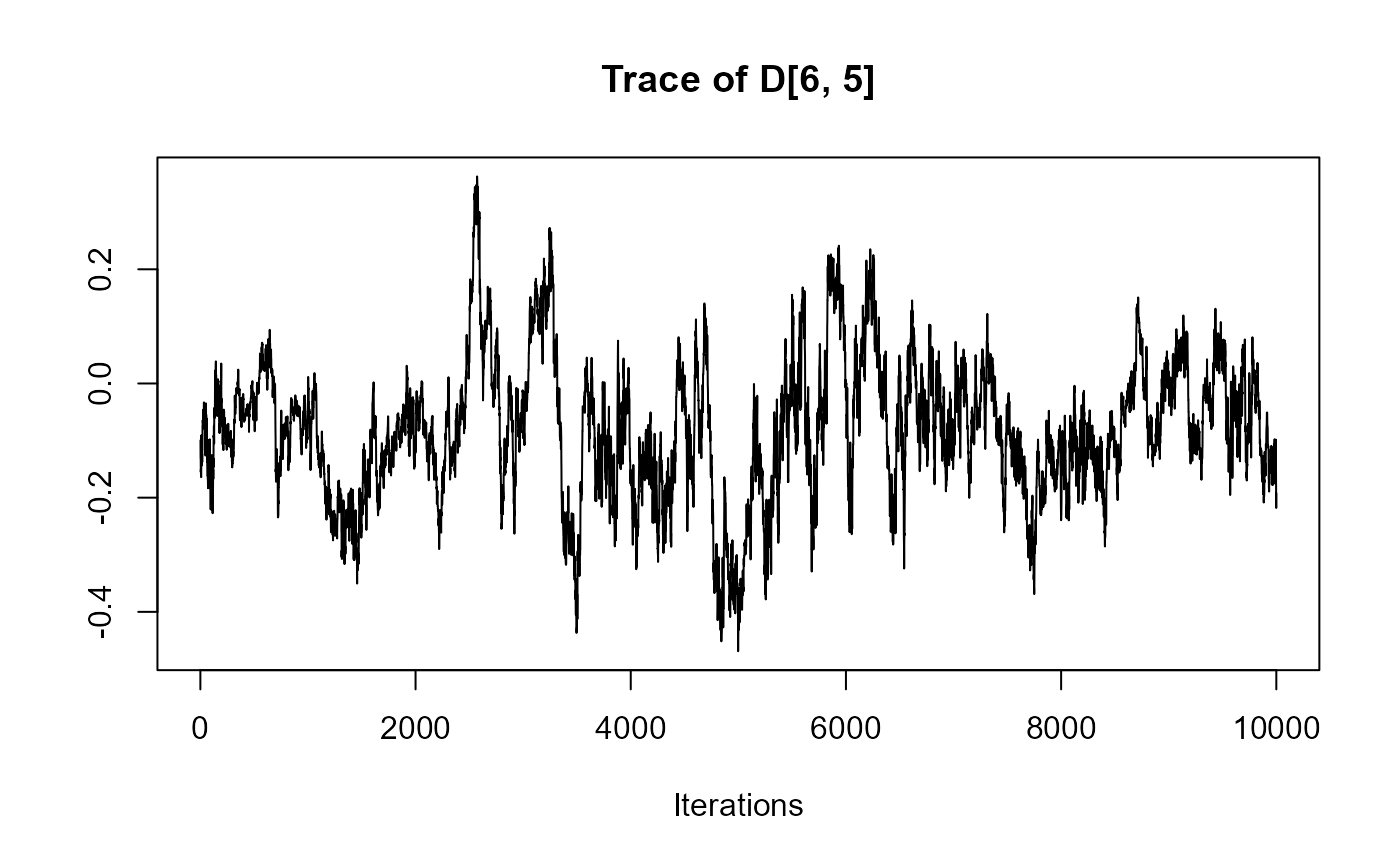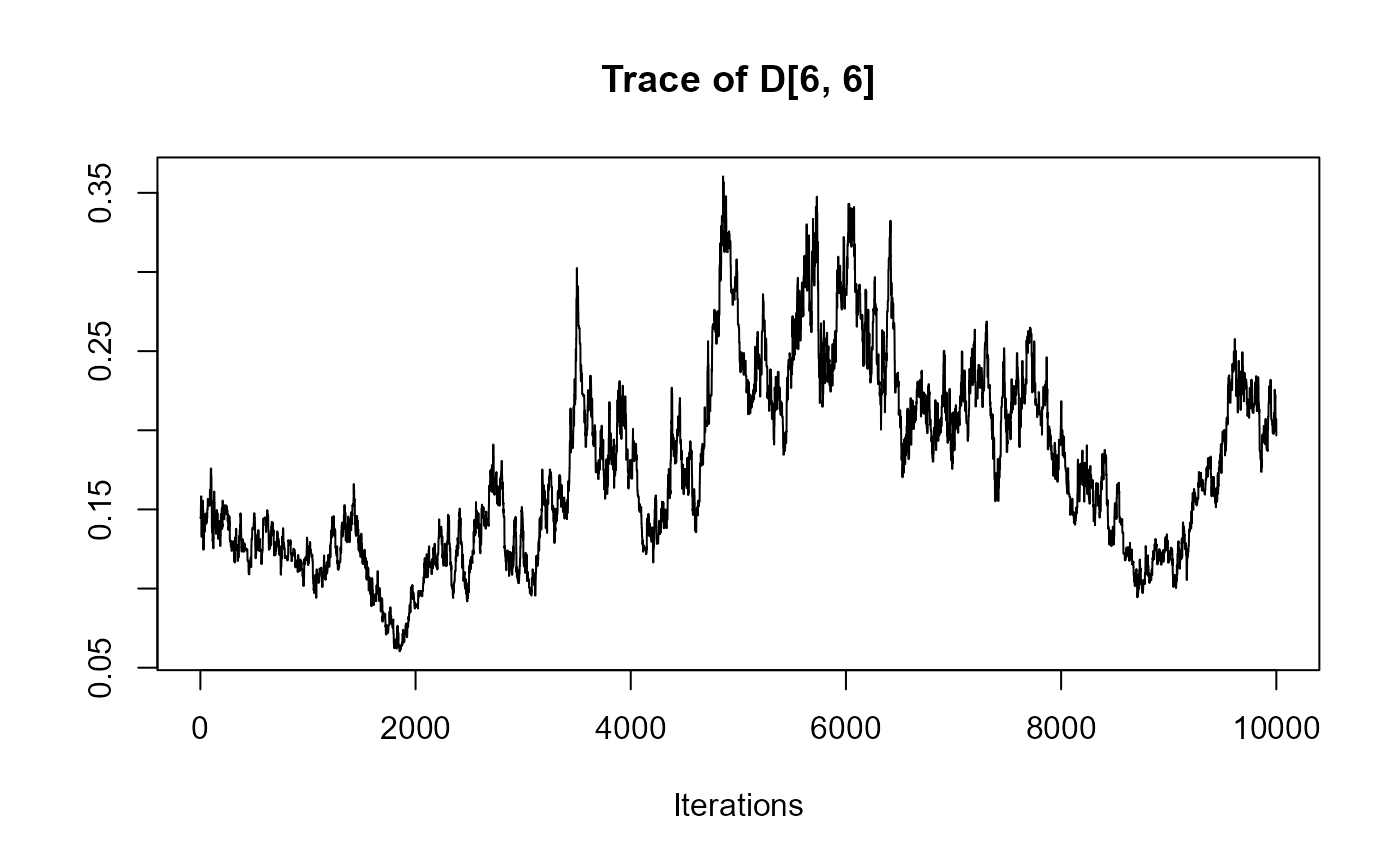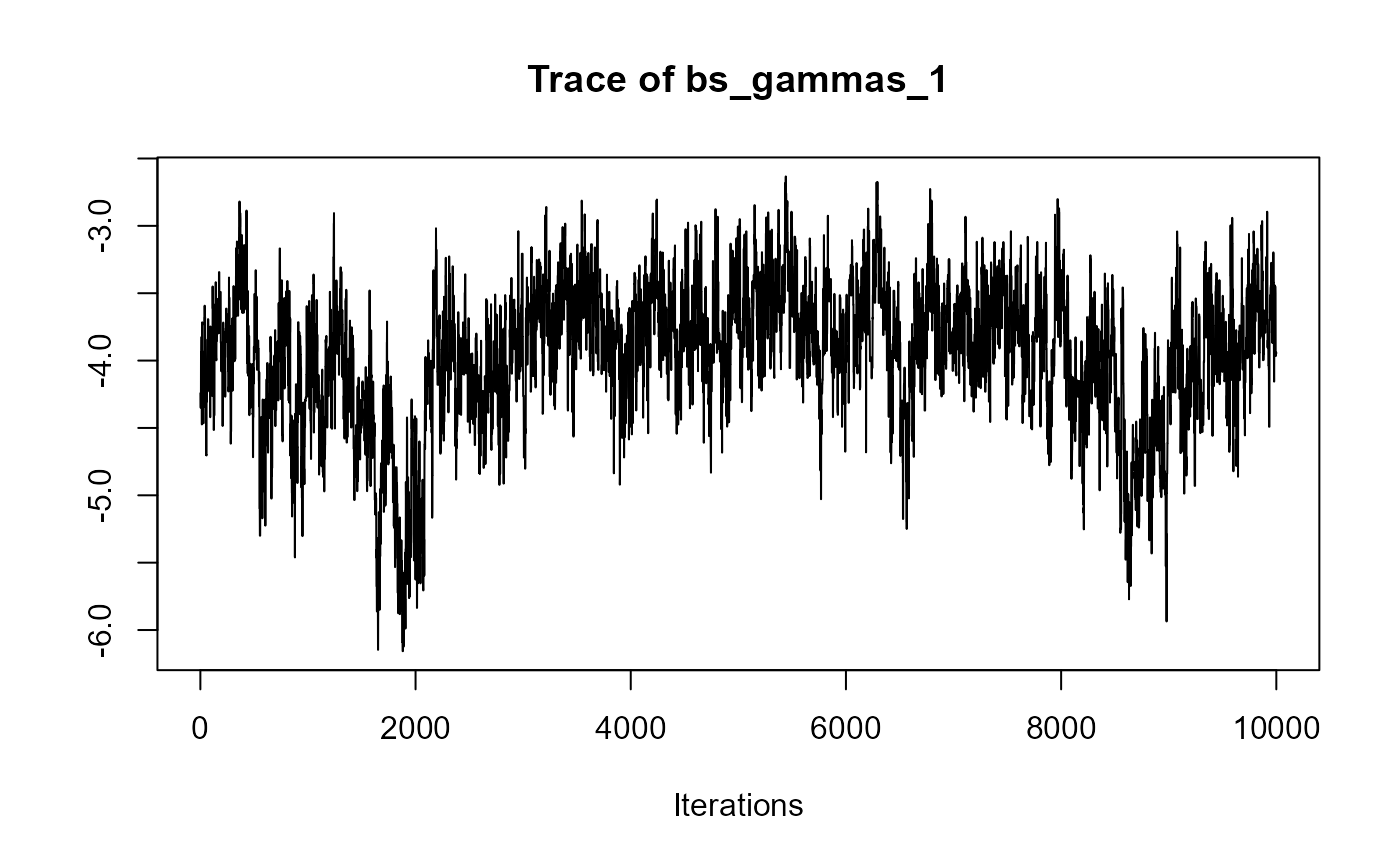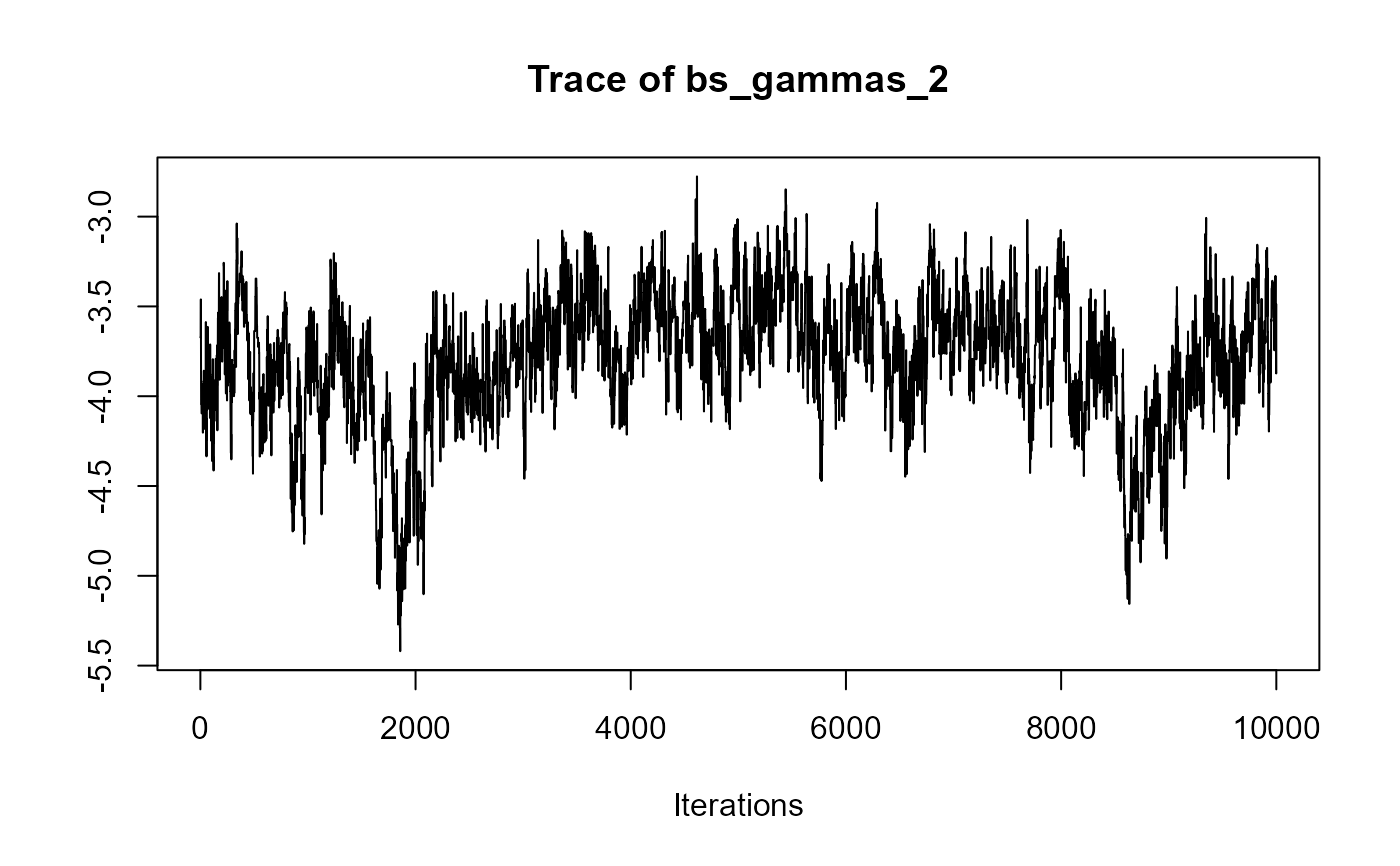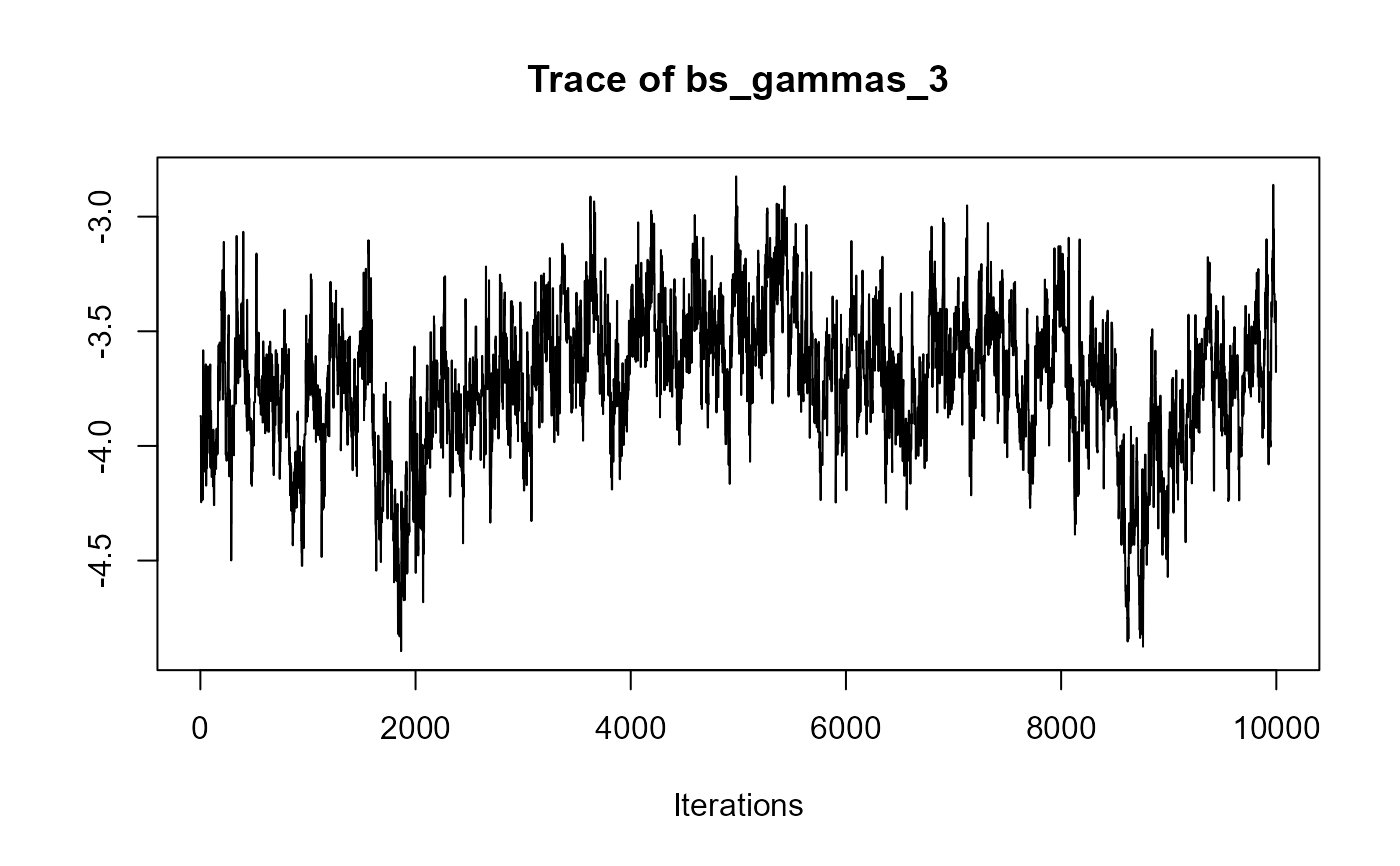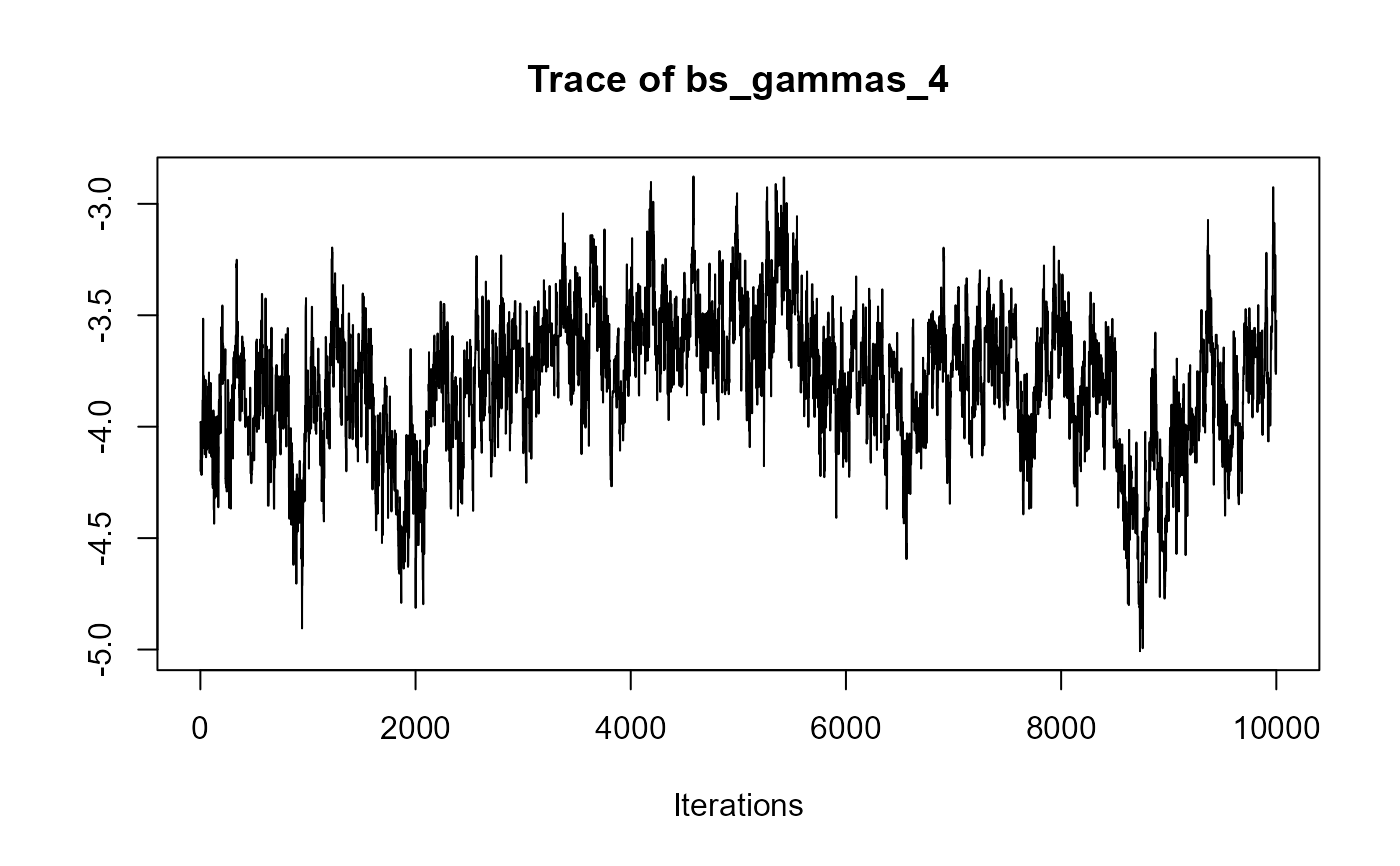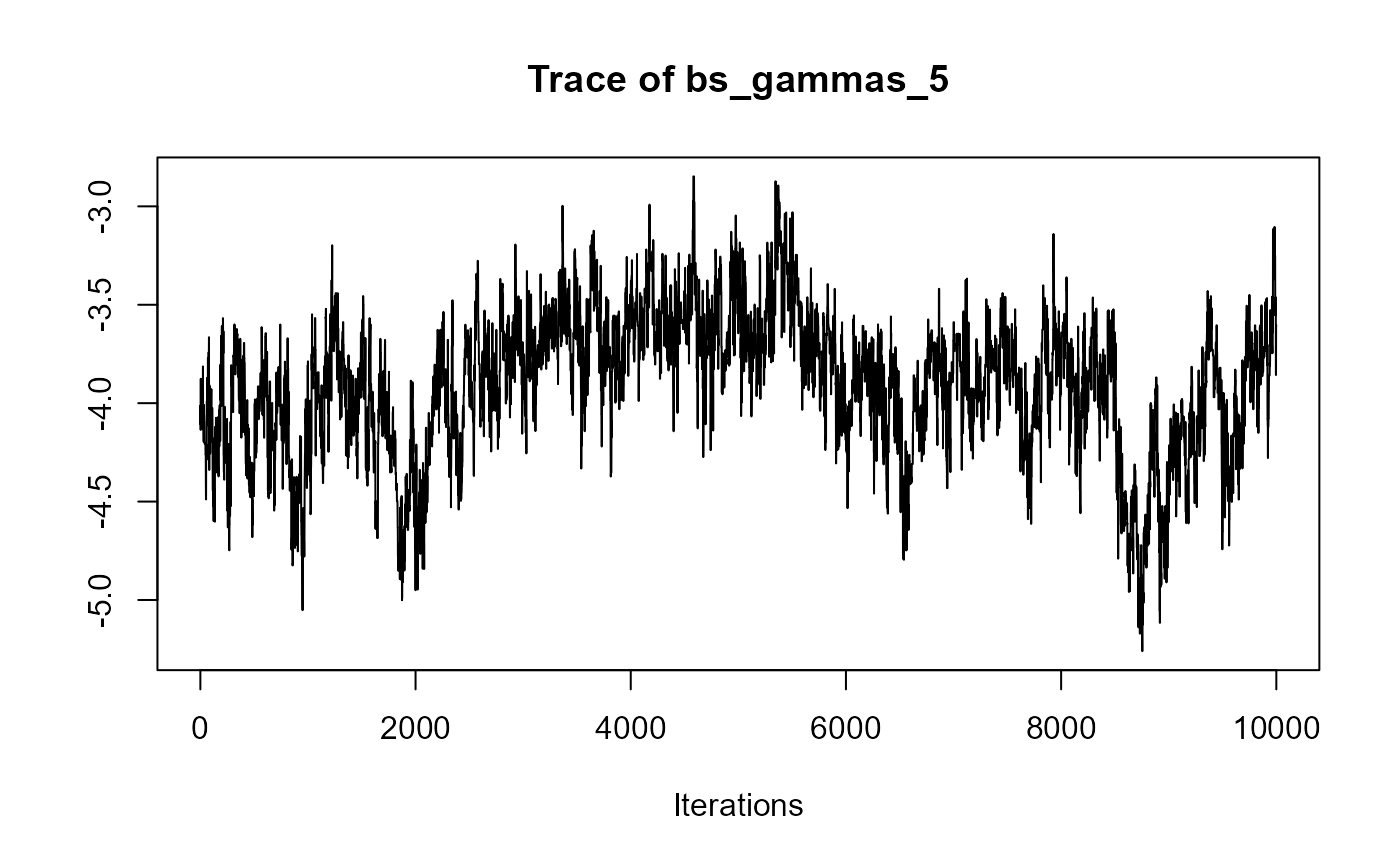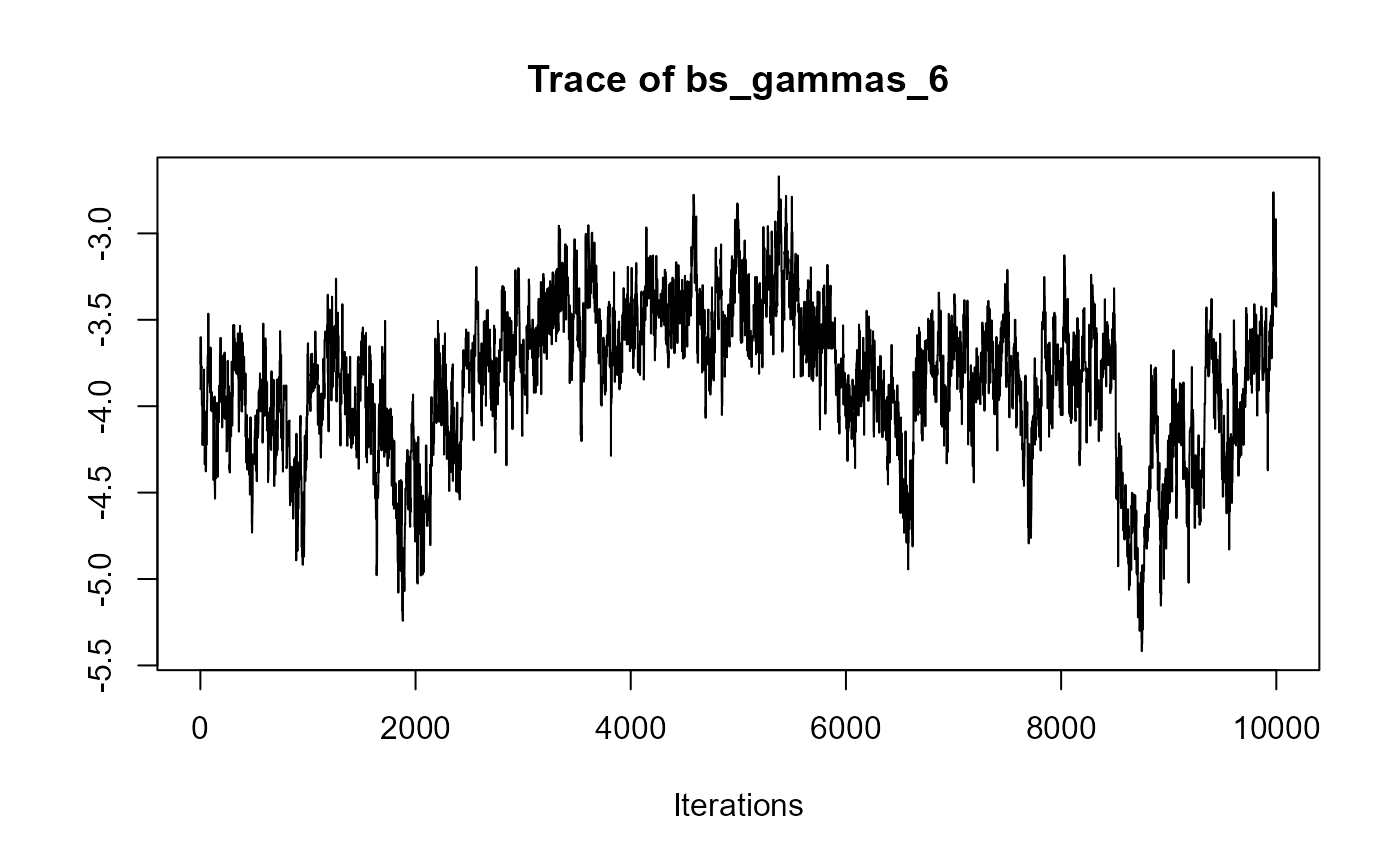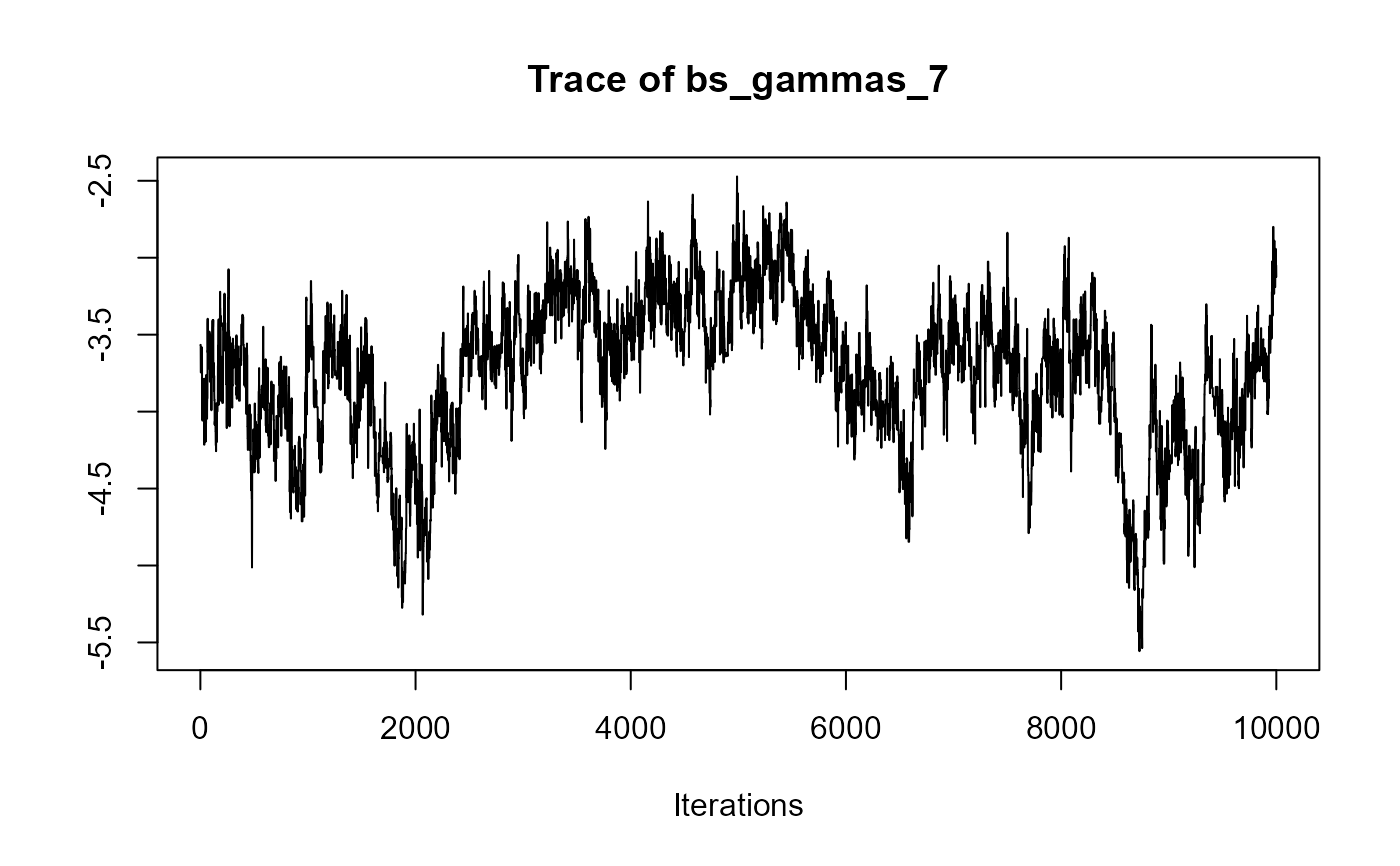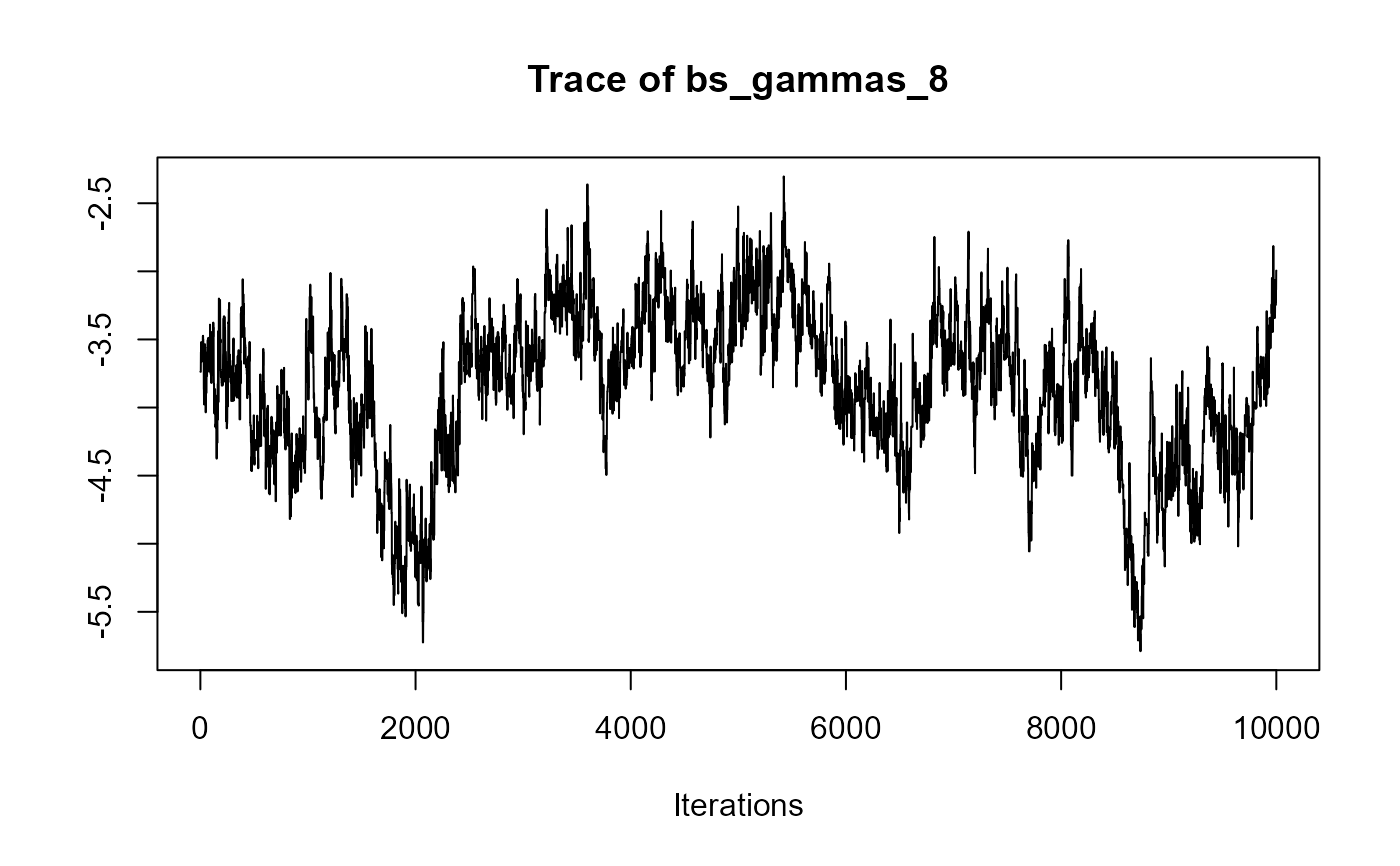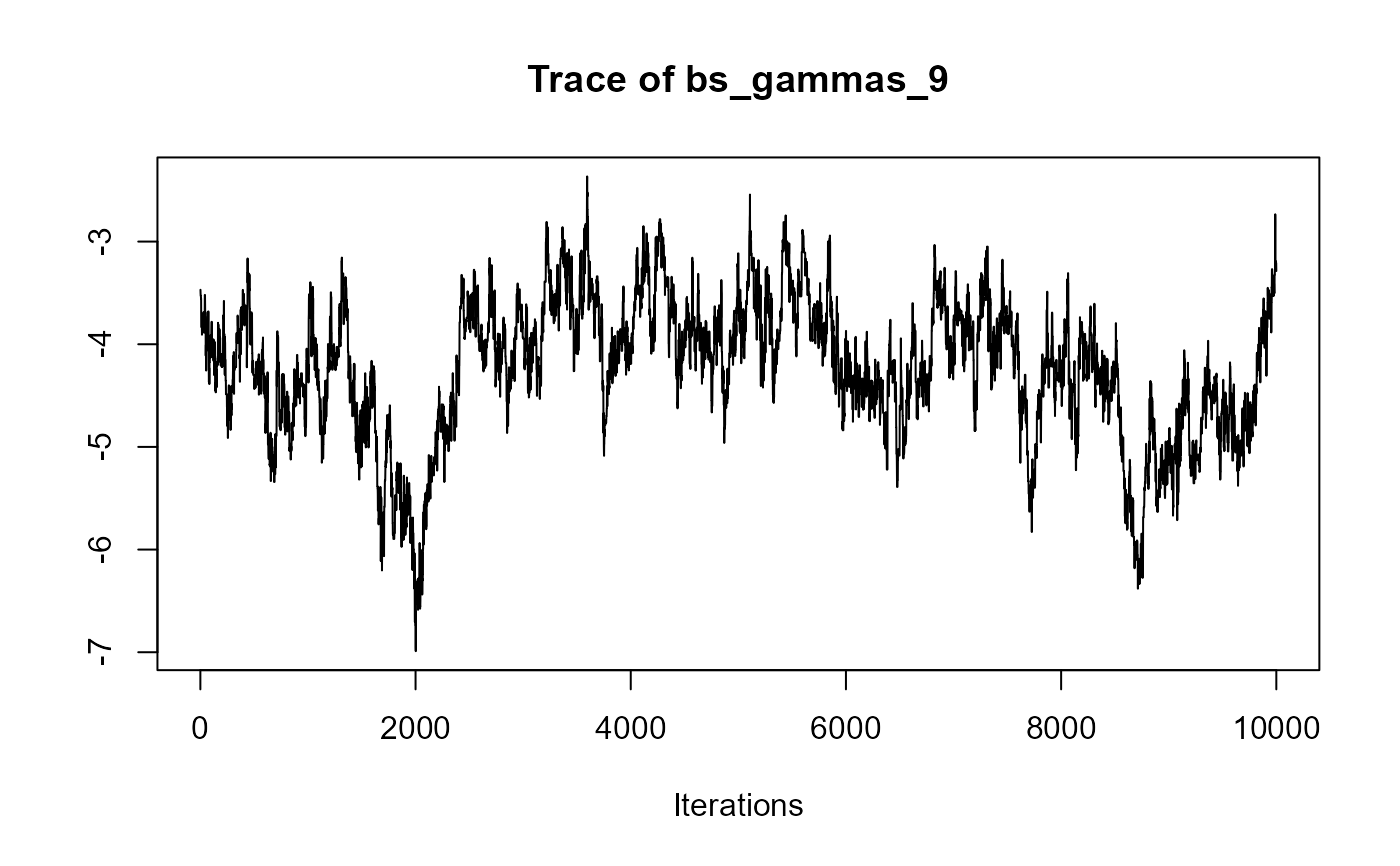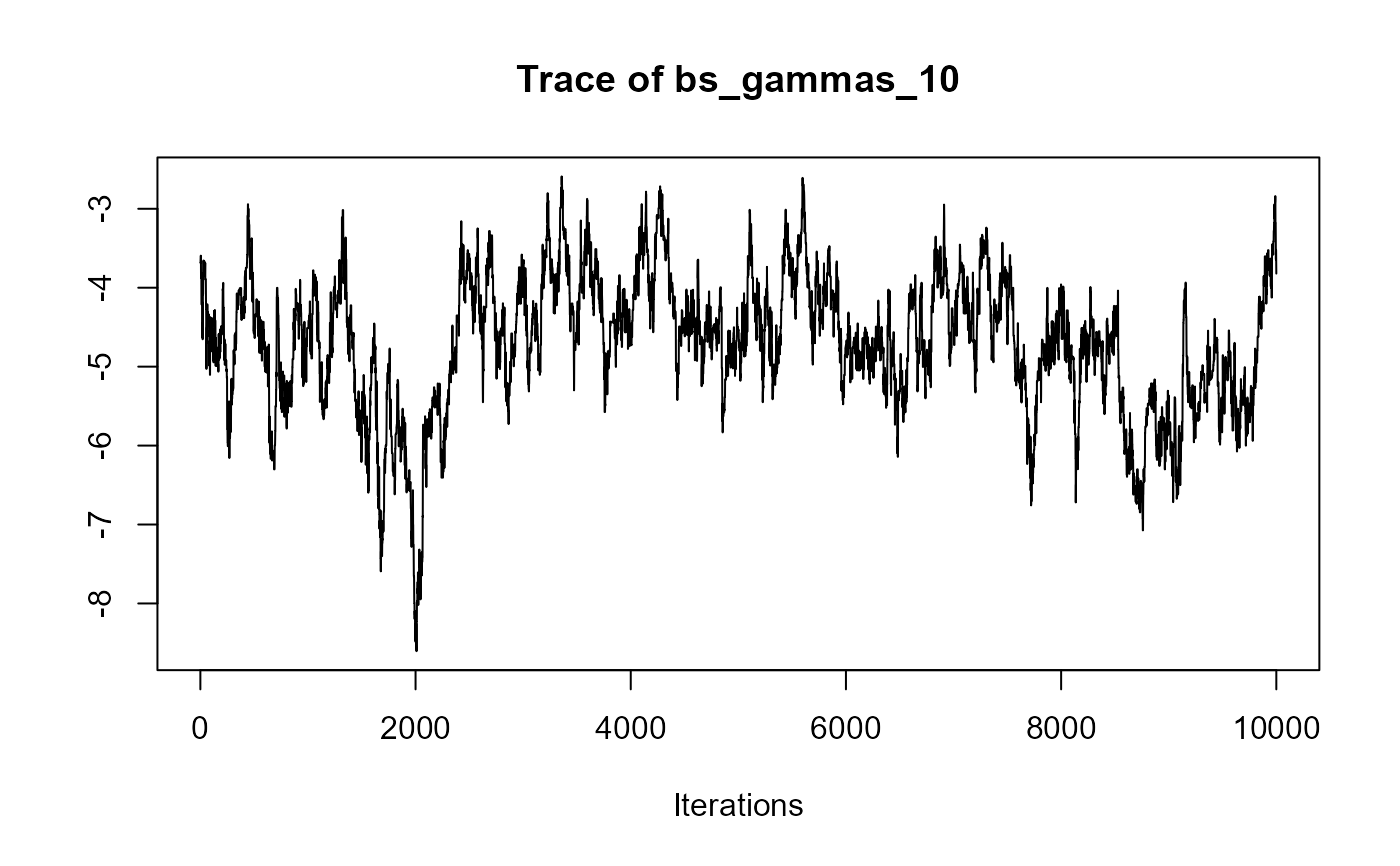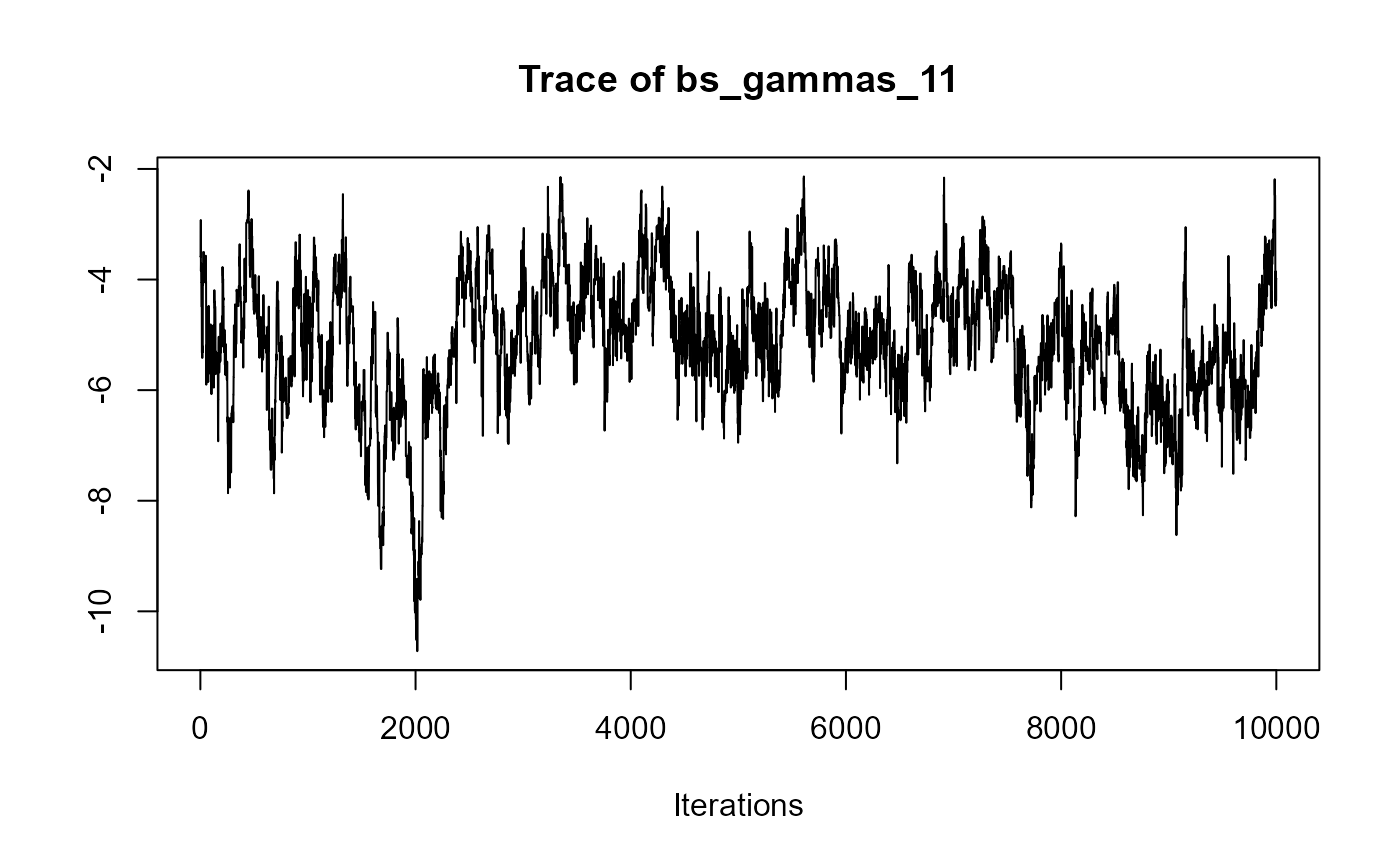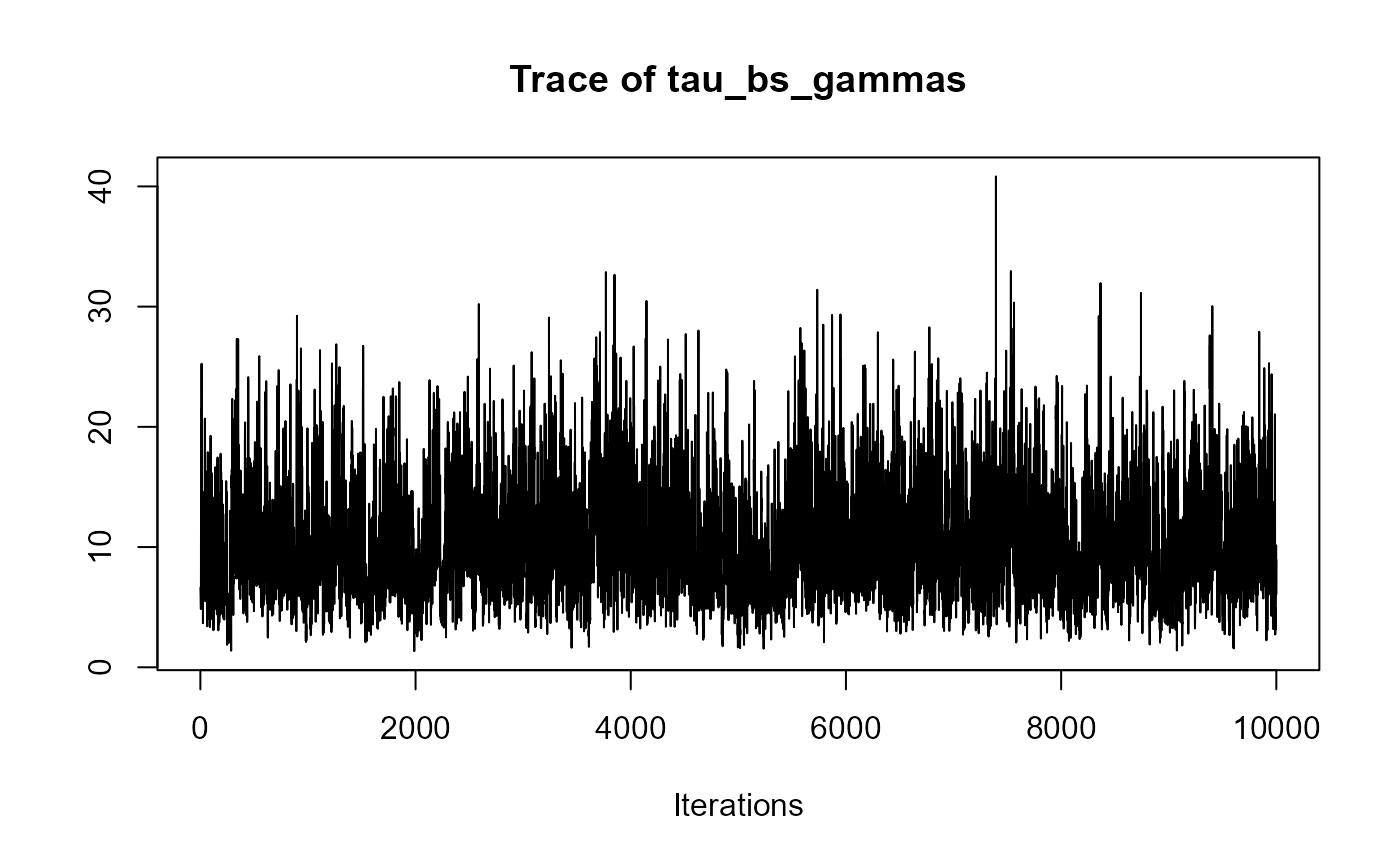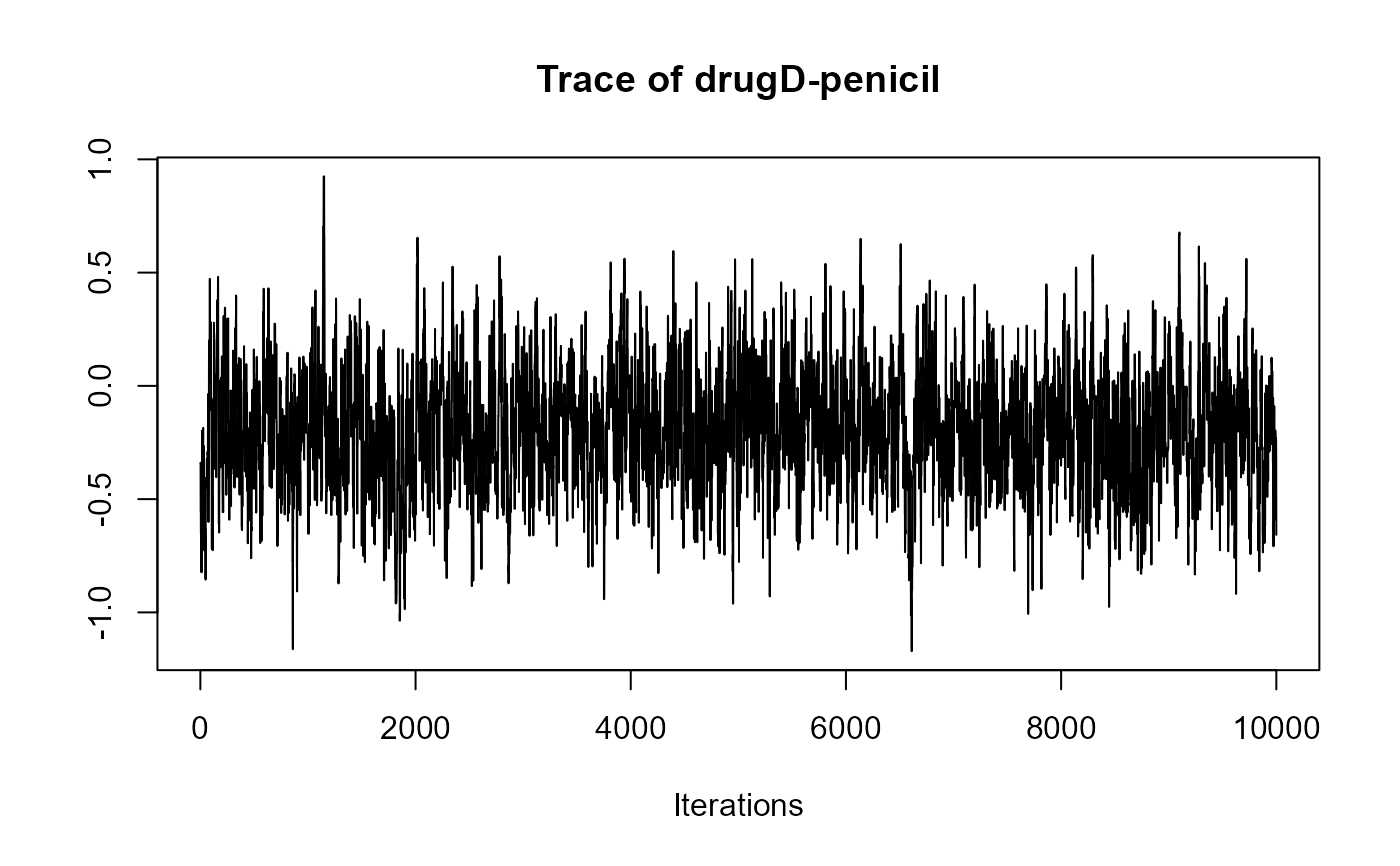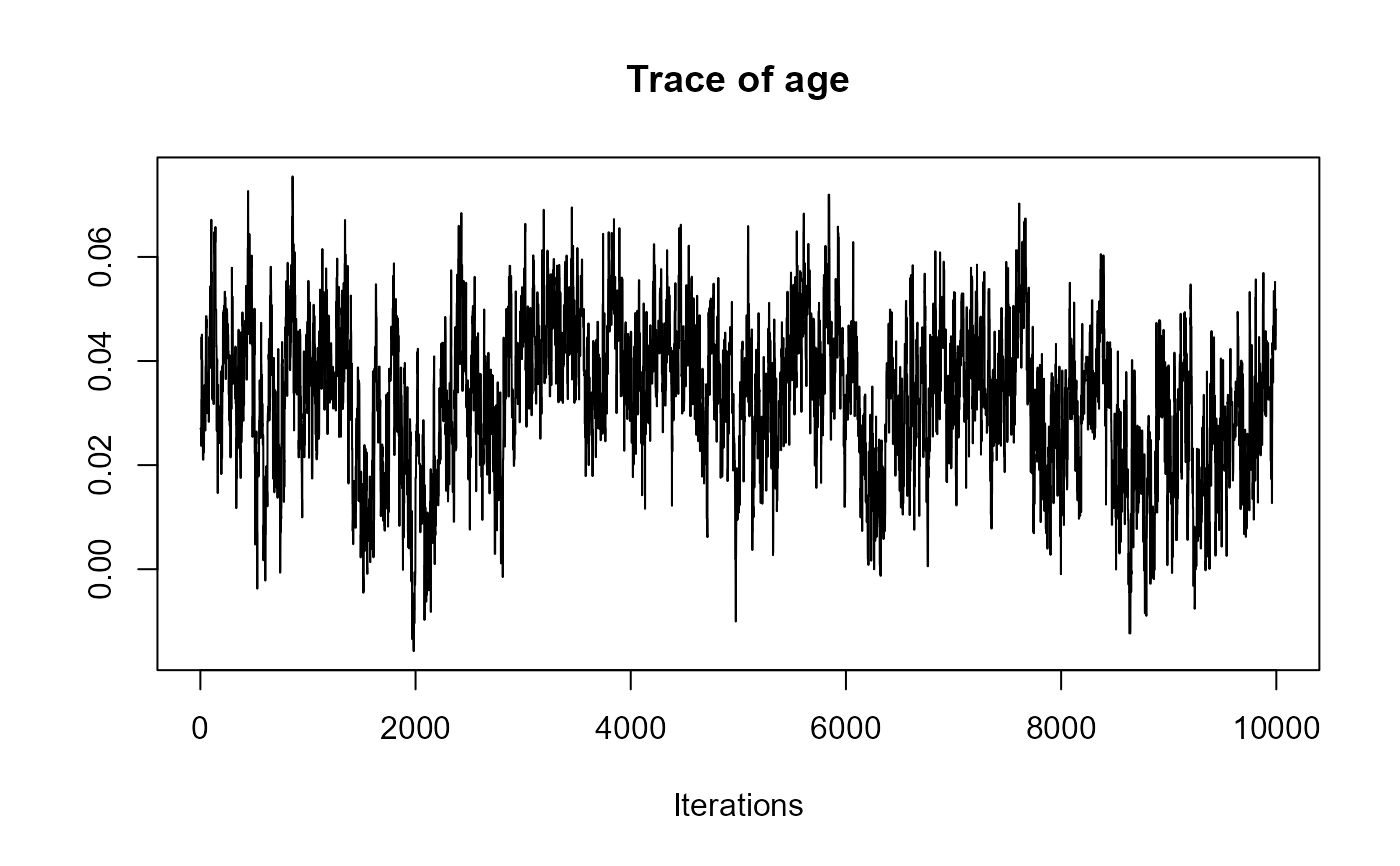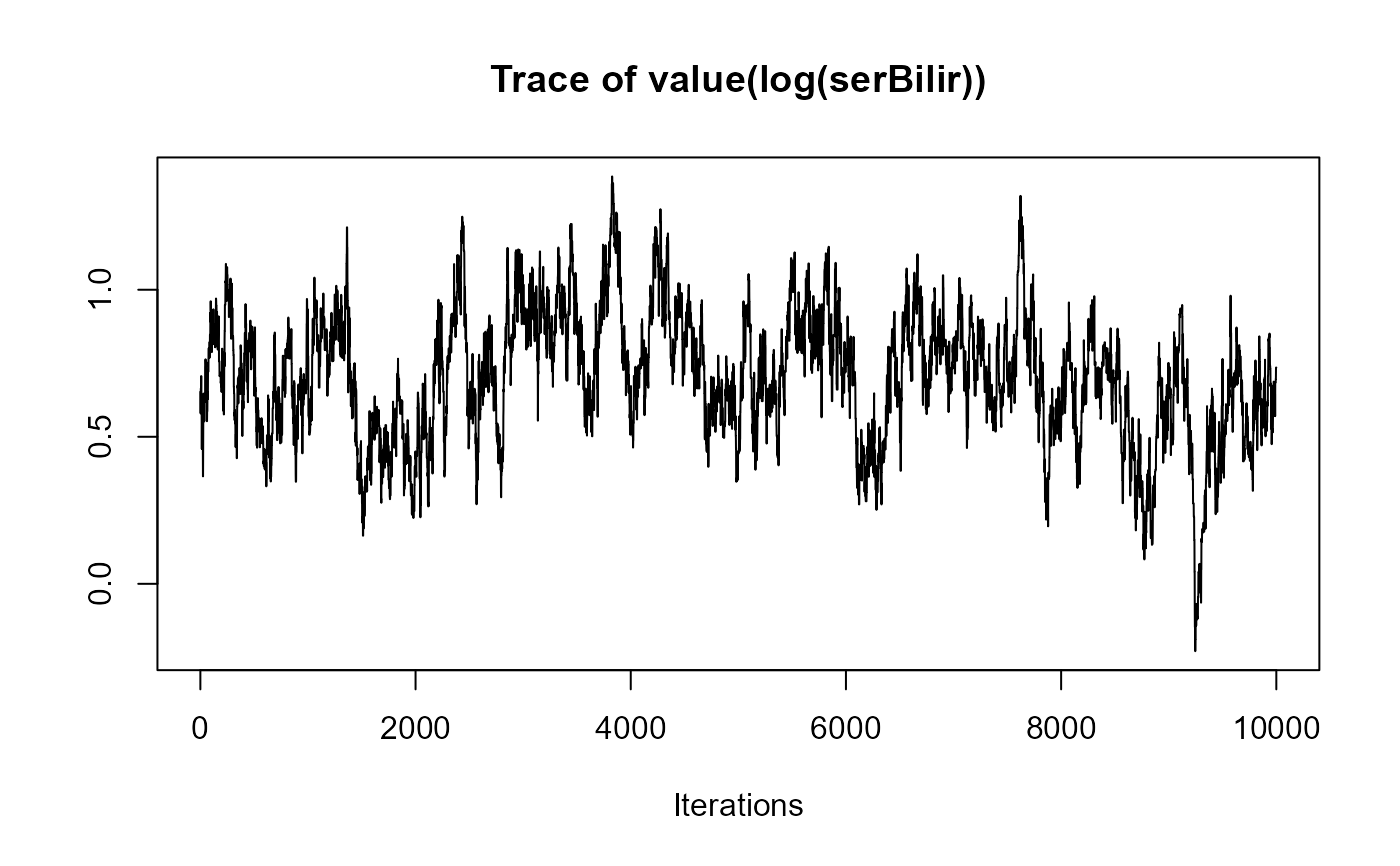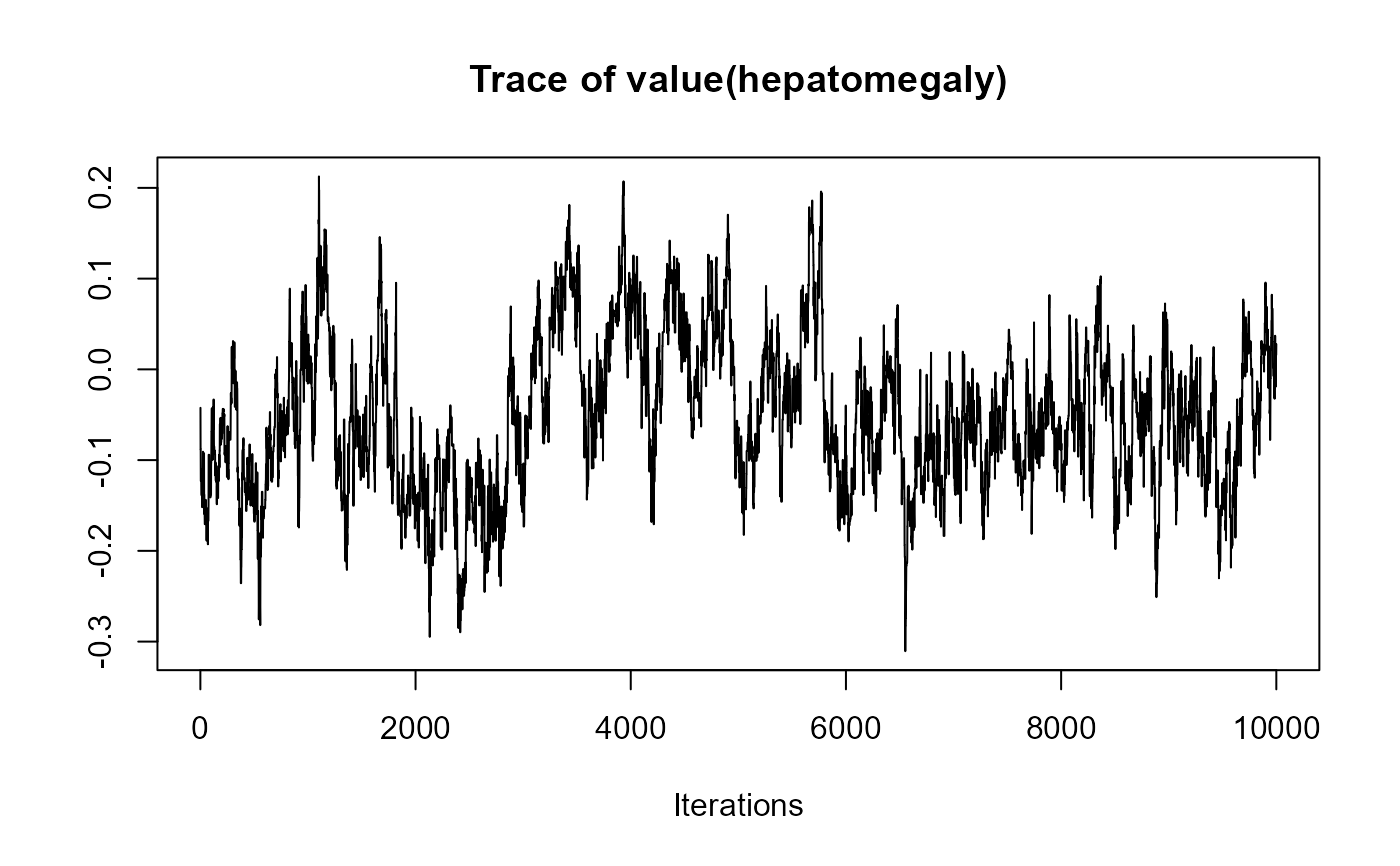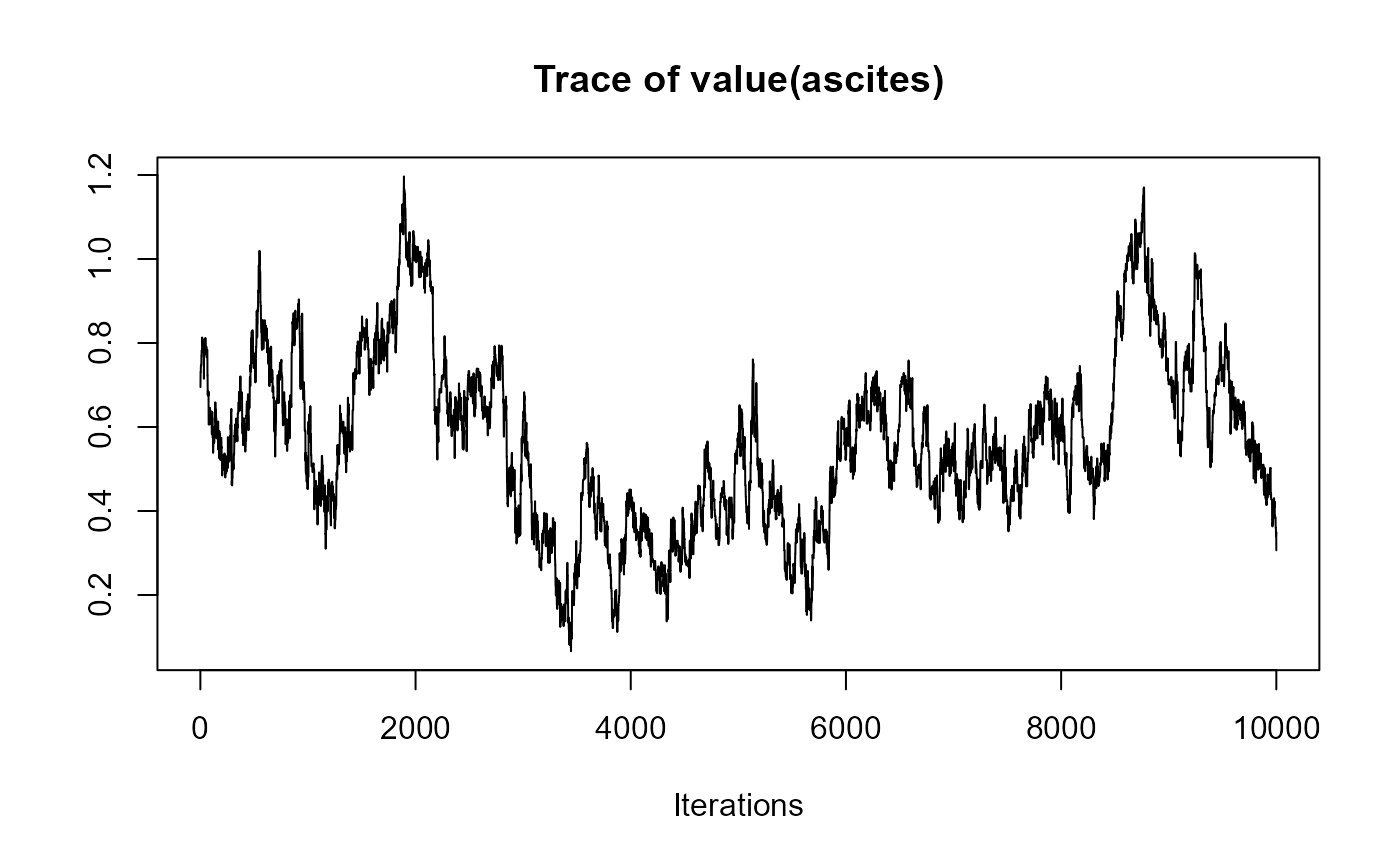################################################################################

######################
# Slope & Area Terms #
######################

# We extend model 'joint_model_fit_2' by including the value and slope term for
# bilirubin, the area term for hepatomegaly (in the log-odds scale), and the
# value and area term for spiders (in the log-odds scale).
# To include these terms into the model, we specify the 'functional_forms'
# argument. This should be a list of right side formulas. Each component of the
# list should have as name the name of the corresponding outcome variable. In
# the right side formula we specify the functional form of the association using
# functions 'value()', 'slope()' and 'area()'.
# Notes: (1) For terms not specified in the 'functional_forms' list, the default
# value functional form is used.

#  Fit the mixed-effects models using lme() for continuous outcomes
# and mixed_model() for categorical outcomes.
fm1 <- lme(fixed = log(serBilir) ~ year * sex, random = ~ year | id, data = pbc2)

fm2 <- mixed_model(hepatomegaly ~ sex + age + year, data = pbc2,
random = ~ year | id, family = binomial())

fm3 <- mixed_model(ascites ~ year + age, data = pbc2,
random = ~ year | id, family = binomial())

#  Save all the fitted mixed-effects models in a list.
Mixed <- list(fm1, fm2, fm3)

#  Fit a Cox model, specifying the baseline covariates to be included in the
# joint model.
fCox1 <- coxph(Surv(years, status2) ~ drug + age, data = pbc2.id)

#  Specify the list of formulas to be passed to the functional_forms argument
# of jm().
fForms <- list("log(serBilir)" = ~ value(log(serBilir)) + slope(log(serBilir)),
"hepatomegaly" = ~ area(hepatomegaly),
"ascites" = ~ value(ascites) + area(ascites))

#  The joint model is fitted using a call to jm() and passing the list
# to the functional_forms argument.
joint_model_fit_2 <- jm(fCox1, Mixed, time_var = "year",
functional_forms = fForms, n_chains = 1L,
n_iter = 11000L, n_burnin = 1000L)
summary(joint_model_fit_2)
#>
#> Call:
#> jm(Surv_object = fCox1, Mixed_objects = Mixed, time_var = "year",
#>     functional_forms = fForms, n_chains = 1L, n_iter = 11000L,
#>     n_burnin = 1000L)
#>
#> Data Descriptives:
#> Number of Groups: 312		Number of events: 140 (44.9%)
#> Number of Observations:
#>   log(serBilir): 1945
#>   hepatomegaly: 1884
#>   ascites: 1885
#>
#>                  DIC     WAIC      LPML
#> marginal    6680.424 6994.006 -3801.697
#> conditional 9039.934 8776.530 -4831.470
#>
#> Random-effects covariance matrix:
#>
#>        StdDev   Corr
#> (Intr) 0.9857 (Intr)   year (Intr)    year  (Intr)
#> year   0.1799 0.4257
#> (Intr) 3.3851 0.5322 0.3484
#> year   0.5863 0.0266 0.3537 -0.3737
#> (Intr) 2.2445 0.6481 0.5906 0.5852  -0.0912
#> year   0.4131 0.4851 0.6751 0.4516  0.3161  0.3191
#>
#> Survival Outcome:
#>                         Mean  StDev    2.5%   97.5%      P
#> drugD-penicil        -0.1293 0.2733 -0.6865  0.3860 0.6384
#> age                   0.0474 0.0136  0.0191  0.0733 0.0002
#> value(log(serBilir))  0.9243 0.2136  0.4952  1.3245 0.0000
#> slope(log(serBilir))  3.7558 1.4494  1.2804  7.0090 0.0034
#> area(hepatomegaly)    0.1215 0.0857 -0.0423  0.3037 0.1398
#> value(ascites)       -0.6878 0.2019 -1.0706 -0.1801 0.0014
#> area(ascites)         1.0615 0.2707  0.3868  1.5535 0.0000
#>
#> Longitudinal Outcome: log(serBilir) (family = gaussian, link = identity)
#>                   Mean  StDev    2.5%   97.5%     P
#> (Intercept)     0.7023 0.1439  0.4211  0.9849 0.000
#> year            0.2585 0.0295  0.2017  0.3157 0.000
#> sexfemale      -0.2412 0.1487 -0.5358  0.0494 0.110
#> year:sexfemale -0.0698 0.0298 -0.1285 -0.0128 0.017
#> sigma           0.3485 0.0067  0.3359  0.3621 0.000
#>
#> Longitudinal Outcome: hepatomegaly (family = binomial, link = logit)
#>                Mean  StDev    2.5%  97.5%      P
#> (Intercept)  0.2974 1.0446 -1.7417 2.3491 0.7678
#> sexfemale   -0.9228 0.5450 -2.0021 0.1650 0.0942
#> age          0.0135 0.0165 -0.0185 0.0457 0.4100
#> year         0.2508 0.0731  0.1050 0.3933 0.0002
#>
#> Longitudinal Outcome: ascites (family = binomial, link = logit)
#>                Mean  StDev    2.5%   97.5% P
#> (Intercept) -8.1994 0.8914 -9.8780 -6.4460 0
#> year         0.4519 0.0722  0.3084  0.5835 0
#> age          0.0760 0.0156  0.0449  0.1054 0
#>
#> MCMC summary:
#> chains: 1
#> iterations per chain: 11000
#> burn-in per chain: 1000
#> thinning: 1
#> time: 1.6 min

# }